# KR101081000B1 - Image processing apparatus and image processing method, and recording medium - Google Patents

Image processing apparatus and image processing method, and recording medium Download PDF

## Info

Publication number
KR101081000B1
KR101081000B1 KR1020040084721A KR20040084721A KR101081000B1 KR 101081000 B1 KR101081000 B1 KR 101081000B1 KR 1020040084721 A KR1020040084721 A KR 1020040084721A KR 20040084721 A KR20040084721 A KR 20040084721A KR 101081000 B1 KR101081000 B1 KR 101081000B1
Authority
KR
South Korea
Prior art keywords
color
pixel
intensity
interest
value
Prior art date
Application number
KR1020040084721A
Other languages
Korean (ko)
Other versions
KR20050039637A (en
Inventor
미츠나가토모오
노무라요시쿠니
Original Assignee
소니 가부시키가이샤
Priority date (The priority date is an assumption and is not a legal conclusion. Google has not performed a legal analysis and makes no representation as to the accuracy of the date listed.)
Filing date
Publication date
Priority to JPJP-P-2003-00363714 priority Critical
Priority to JP2003363714 priority
Application filed by 소니 가부시키가이샤 filed Critical 소니 가부시키가이샤
Publication of KR20050039637A publication Critical patent/KR20050039637A/en
Application granted granted Critical
Publication of KR101081000B1 publication Critical patent/KR101081000B1/en

## Images

•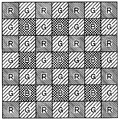•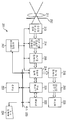•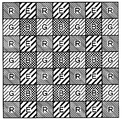•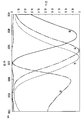•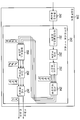•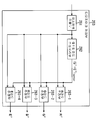•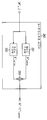••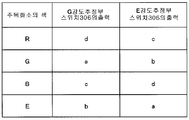•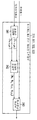••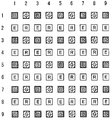••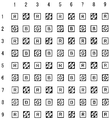•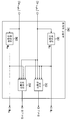••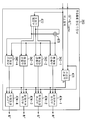•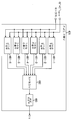••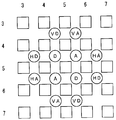•••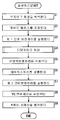•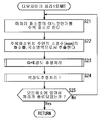•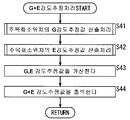•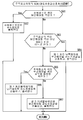•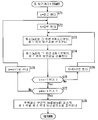••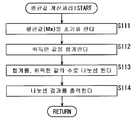•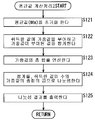•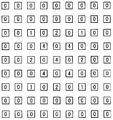••••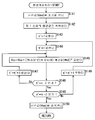•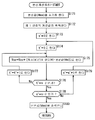•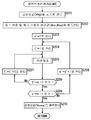•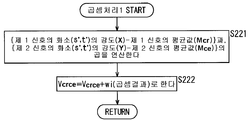•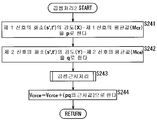•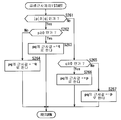•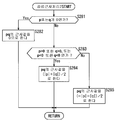•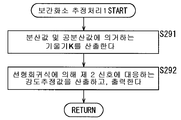•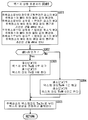•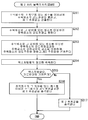•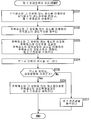•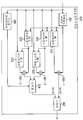•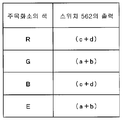•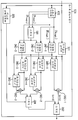•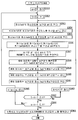•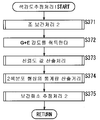•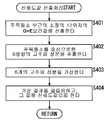•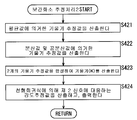•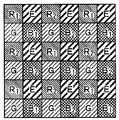•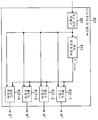•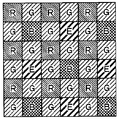••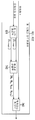•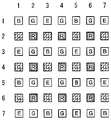•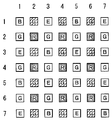•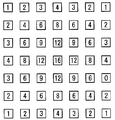••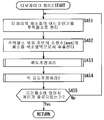•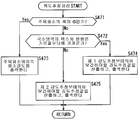•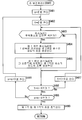•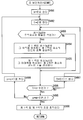•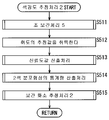•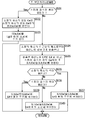••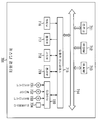## Classifications

• HELECTRICITY
• H04ELECTRIC COMMUNICATION TECHNIQUE
• H04NPICTORIAL COMMUNICATION, e.g. TELEVISION
• H04N9/00Details of colour television systems
• H04N9/04Picture signal generators
• H04N9/045Picture signal generators using solid-state devices

## Abstract

Suppress the occurrence of jagginess or noise.
The G intensity estimation unit 291 calculates an estimated value of the G intensity at the pixel position of interest by using the pixel of the local area. The E intensity estimation unit 292 calculates an estimated value of the E intensity at the pixel position of interest by using the pixel in the local area. The addition processing unit 293 adds the calculated G intensity estimate value and the E intensity estimate value to calculate the (G + E) intensity estimate value of the pixel position of interest. In the interpolation processing of G or E only in the mosaic image by the color filter of the primary color four-color array in which G and E are only present at intervals of one pixel, high frequency image signals cannot be reproduced. By reproducing a signal such as the signals of G and E arranged at a position shifted by one pixel vertically at all pixel positions, it is possible to reproduce a high-frequency image signal higher than each limit, thereby outputting an image. It is possible to suppress the occurrence of jagness and noise. The present invention can be applied to a digital camera.

## Description

Image processing apparatus and image processing method and recording medium {IMAGE PROCESSING APPARATUS AND IMAGE PROCESSING METHOD, AND RECORDING MEDIUM}

1 is a diagram for explaining a Bayer arrangement.

2 is a block diagram showing the configuration of a digital still camera to which the present invention is applied.

FIG. 3 is a diagram for explaining a color filter used for the CCD image sensor of FIG. 2. FIG.

4 is a diagram illustrating an example of spectral characteristics of the color filter of FIG. 3.

FIG. 5 is a block diagram showing the structure of the DSP block of FIG. 2; FIG.

FIG. 6 is a block diagram showing the configuration of the demosaicing processor of FIG. 5; FIG.

FIG. 7 is a block diagram showing the configuration of the G + E intensity estimation processing unit in FIG. 6; FIG.

FIG. 8 is a block diagram showing the configuration of the G intensity estimating unit and the E intensity estimating unit in FIG. 7. FIG.

FIG. 9 is a diagram for explaining processing of the switch of FIG. 8. FIG.

FIG. 10 is a block diagram showing the configuration of the first to fourth intensity estimating units in FIG. 8. FIG.

FIG. 11 shows the interpolation interpolation of FIG. 10; FIG. rough interpolation} A diagram for explaining a first color and a second color selected by a processing unit.

FIG. 12 is a diagram for explaining a first color and a second color selected by the interpolation interpolation processor of FIG. 10; FIG.

FIG. 13 is a diagram for explaining a first color and a second color selected by the interpolation interpolation processor of FIG. 10; FIG.

FIG. 14 is a diagram for explaining a first color and a second color selected by the interpolation interpolation processor of FIG. 10; FIG.

FIG. 15 is a block diagram showing a configuration of a statistical calculation unit in FIG. 10. FIG.

FIG. 16 is a block diagram showing the configuration of the regression calculation processing unit in FIG. 10; FIG.

FIG. 17 is a block diagram showing the configuration of an RGBE intensity estimation unit in FIG. 6; FIG.

18 is a block diagram showing the configuration of a reliability calculator of FIG. 17;

19 is a diagram for explaining an extraction position of pixel intensity in a reliability calculator.

20 is a diagram for explaining a set of pixel intensities for extracting high frequency components in a reliability calculation unit;

FIG. 21 is a diagram for explaining processing of the interpolation interpolation unit in FIG. 17. FIG.

FIG. 22 is a block diagram showing the configuration of the regression calculation processing unit in FIG. 17. FIG.

FIG. 23 is a flowchart for explaining image processing executed by the DSP block in FIG. 5; FIG.

24 is a flowchart for explaining demosaicing processing 1. FIG.

25 is a flowchart for explaining a G + E intensity estimation process.

Fig. 26 is a flowchart for explaining a G (E) intensity estimation value calculation processing at the pixel position of interest;

27 is a flowchart for explaining the interpolation interpolation process 1. FIG.

Fig. 28 is a flowchart for explaining a statistical calculation calculation process of a two-color distribution shape.

29 is a flowchart for explaining an average value calculation process 1. FIG.

30 is a flowchart for explaining an average value calculation process 2. FIG.

31 is a diagram for explaining a weight value.

32 is a diagram for explaining a weight value.

33 is a diagram for explaining a weight value.

34 is a diagram for explaining a weight value.

35 is a flowchart for explaining the dispersion calculation process 1. FIG.

36 is a flowchart for explaining the distribution calculation process 2. FIG.

37 shows covariance; covariance} Flowchart for explaining the calculation process.

38 shows multiplication; multiplication} Flowchart for explaining process 1.

39 is a flowchart for explaining a multiplication process 2. FIG.

40 is a flowchart for explaining a multiplication approximation process 1. FIG.

41 is a flowchart for explaining a multiplication approximation process 2. FIG.

42 is a flowchart for explaining interpolation pixel estimation processing 1. FIG.

43 is a flowchart for explaining texture direction determination processing.

44 is a flowchart for explaining the processing of the second processing block.

45 is a flowchart for explaining processing of the third processing block.

46 is a block diagram showing another configuration of the G + E intensity estimation processing unit.

FIG. 47 is a diagram for explaining processing of the switch in FIG. 46; FIG.

48 is a block diagram showing another configuration of the G + E intensity estimation processing unit.

49 is a flowchart for explaining the interpolation interpolation processing 2. FIG.

50 is a flowchart for explaining the color intensity estimation process 1. FIG.

51 is a flowchart for explaining a reliability value calculation process.

52 is a flowchart for explaining interpolation pixel estimation processing 2. FIG.

FIG. 53 is a diagram for explaining another example of the color filter used in the CCD image sensor of FIG. 2; FIG.

FIG. 54 is a block diagram illustrating another configuration example of the demosaicing processor of FIG. 5; FIG.

FIG. 55 is a view for explaining an example of a color filter used for the CCD image sensor of FIG. 2 when the demosaicing processor of FIG. 54 is used;

FIG. 56 is a block diagram showing a configuration of the brightness estimation processing unit in FIG. 54;

FIG. 57 is a block diagram showing the configuration of the first and second intensity estimation units in FIG. 56; FIG.

FIG. 58 is a diagram for explaining a first color and a second color selected by the interpolation interpolation processor of FIG. 57; FIG.

FIG. 59 is a diagram for explaining a first color and a second color selected by the interpolation interpolation processor of FIG. 57; FIG.

60 is a diagram for explaining a weight value.

FIG. 61 is a block diagram showing a configuration of an RGBE intensity estimation unit in FIG. 54; FIG.

Fig. 62 is a flowchart for explaining demosaicing processing 2;

63 is a flowchart for explaining a luminance estimation process.

64 is a flowchart for explaining the interpolation interpolation processing 3. FIG.

65 is a flowchart for explaining the interpolation interpolation process 4. FIG.

66 is a flowchart for explaining the color intensity estimation process 2. FIG.

67 is a flowchart for explaining the interpolation interpolation process 5. FIG.

FIG. 68 is a view for explaining another example of the color filter used for the CCD image sensor of FIG. 2 when the demosaicing processor of FIG. 54 is used;

69 is a block diagram showing the structure of a personal computer;

<Explanation of symbols for the main parts of the drawings>

201: Digital Still Camera 216: DSP Block

242: processor for signal processing 253: demosaicing processor

281: local region extraction unit 282: G + E intensity estimation processing unit

283: RGBE intensity estimation unit 291: G intensity estimation unit

292: E intensity estimating unit 293: Addition processing unit

301: Attention pixel selection unit 305: Texture direction determination unit

306: switch 311: first intensity estimation unit

321: second intensity estimating unit 322: third intensity estimating unit

323: fourth intensity estimating unit 324: switch

341: interpolation processing unit 342: statistics calculation unit

343: regression processing unit 361: average value calculation unit

362: dispersion value calculation unit 363: average value calculation unit

364: covariance calculator 381: gradient calculator

382: pixel intensity estimator 471: reliability calculator

472: interpolation processing unit 473: regression processing unit

481: high frequency extraction unit 482: addition processing unit

483: clip processing unit 501,502: slope calculation unit

503: gradient synthesis unit 504: pixel intensity estimation unit

551: G + E intensity estimation processing unit 562: switch

571: G + E intensity estimation processing unit 581: switch

601: demosaicing processor 611: luminance estimation processor

612: RGBE intensity estimation unit 621: first intensity estimation unit

622: second intensity estimating unit 623: switch

624 switch 631: jaw interpolation processing unit

641: Joe interpolation processing unit

BACKGROUND OF THE INVENTION 1. Field of the Invention The present invention relates to an image processing apparatus, an image processing method, and a program, and more particularly, to obtain a color image signal by interpolating a plurality of colors to all pixels from a mosaic image signal obtained by using a color solid-state imaging element. The present invention relates to an image processing apparatus, an image processing method, and a program suitable for use in the case where demosaicing processing (color interpolation processing or synchronization processing) is performed.

Solid-state imaging devices such as CCD image sensors and CMOS image sensors usually have a structure in which light-receiving elements are arranged in a lattice shape and can sequentially read out the amount of charges generated by photoelectric conversion of each light-receiving element. Usually, since these light receiving elements have a single spectral characteristic, the image signal obtained from a solid-state image sensor is one channel (monochrome) with respect to color. Therefore, when it is desired to obtain a color image (for example, a three-channel image such as RGB) in one solid-state image pickup device, a solid-state image pickup device equipped with a filter having a different spectral characteristic (color) for each light receiving element is used. The imaging device using one such color solid-state imaging device is generally called a single-plate color imaging device.

Since the image signal obtained from the color solid-state image sensor is a one-channel image, only the intensity of the color of the filter of the corresponding light receiving element is obtained for each pixel. That is, the image signal obtained from a color solid-state image sensor becomes a mosaic image regarding color. In order to obtain a multichannel image from the mosaic image which is the output image of the color solid-state image sensor of a single-plate color imaging device, it is necessary to interpolate the color information of each pixel of a mosaic image to the pixel position of its periphery by appropriate image processing. Such image processing is generally called color interpolation processing, demosaicing processing, or synchronization processing. As described above, demosaicing is an essential process for single-sheet color imaging devices using color solid-state imaging devices, and various technologies have been developed in the past.

A problem in such demosaicing is the reproduction characteristic of the high frequency image signal. In a solid-state imaging device, different on-chip color filters are attached to light receiving devices arranged in a two-dimensional lattice shape. Because of sticking, pixels having the same color, that is, the same spectral sensitivity, are arranged only at a pitch larger than that of the original light receiving element. For this reason, aliasing is more likely to occur in the picked-up image as compared with the monochrome solid-state imaging device without the on-chip color filter. When aliasing occurs in the luminance component of an image, it is often observed as jagginess in the object contour. In demosaicing, how to suppress jagness is an important problem.

A primary color Bayer array, which is the most widely used color array, is shown in FIG. 1. In the Bayer arrangement, three primary color filters of R, G, and B are used, and G is a checkered shape. checkered manner}, where R and B are lined up; line-sequential} (hereinafter, the primary color-based Bayer array is simply referred to as Bayer array). In the Bayer arrangement, since G is arranged in a checkered shape, G signals exist in all phases of horizontal and vertical, but since R and B are line sequential, corresponding signals are horizontal and vertical. There is only one out of every other line.

Since Bayer arrays have the most densely present G having the spectral characteristics closest to human visibility in RGB, according to the technique used in the prior art, only the luminance component is obtained from the pixel information of G. The way to make it is mainstream. In this method, the threshold frequency of the luminance component to be reproduced is determined by the sampling frequency of G in the Bayer array, which is 0.5 cycles / pixel in the horizontal and vertical directions and 1 / {2 × sqrt (2 in the 45 degree tilt direction). )} cycle / pixel. Theoretically, the limit is the highest horizontally or vertically, but in reality, G exists only every other pixel in the horizontal and vertical directions, so to reproduce horizontal or vertical waves of O.5 cycles / pixel, It is necessary to apply an optimal interpolation filter to each vertical wave. For this problem, an optimal interpolation filter is prepared for each of the horizontal and vertical waves, the direction of the wave in each local region on the image is determined, and the results of the horizontal interpolation filter are determined according to the discriminated result. There exists a technique which makes the value which synthesize | combined the result of the interpolation filter of a vertical direction into an interpolation value (for example, patent document 1 and nonpatent literature 1). By using these techniques, it is possible to reproduce high frequency close to the theoretical limit of 0.5 cycles / pixel in the horizontal and vertical directions using G pixel information of the Bayer array.

Patent Document 1 : Japanese Patent Laid-Open No. 7-236147

[Non-Patent Document 1] Murata, Mori, Maenaka, Okada, and Chihara, "Correlation Discrimination Color Separation Method in PS-CCD," Journal of the Korea Information and Media Society, Vol. 55, No. 1, pp. 120 -132. 2001

On the other hand, since it is impossible in principle to match the spectral characteristics of the on-chip color filter used in the color solid-state image pickup device to the isotonic function of human vision, for example, one color of the three primary colors of the Bayer array can be used. By using an image pickup device that obtains the added four colors of intensity, the liner matrix is applied to the intensity values of four colors obtained by such an image pickup device to obtain three primary colors of intensity values. There is a way to improve color reproduction. For example, there is a technique capable of improving color reproducibility by using a color solid-state imaging device having a four-color array in which a yellow on-chip filter is added to the conventional three primary colors in order to obtain four colors of intensity (for example, Patent document 2).

Patent Document 2 : Japanese Patent Laid-Open No. 2002-271804

However, in the prior art using a color filter of four color arrays, it has not been specifically shown what kind of demosaicing is performed to obtain four color intensities synchronized to each pixel from the four color arrays.

The four-color arrangement of the complementary color system (cyan, magenta, yellow, green) has conventionally been used in imaging devices such as video cameras, but similarly to this, color filters having a four-color primary color arrangement are also used. In the conventional four-color color arrangement, half of all pixels cannot be assigned to G like the Bayer arrangement, and therefore, the conventional technique of reproducing high frequency of the luminance component using the checkered G arrangement cannot be used. do. Therefore, when the conventional primary color four-color array is used, the color reproduction is improved, but the high frequency component of luminance is not reproduced, and the jagness is remarkably displayed, or it becomes an image without coloration such as smoothing. There was a problem.

SUMMARY OF THE INVENTION The present invention has been made in view of such a situation, and in a single-plate color imaging device using an arrangement composed of four or more colors of the primary color system, the reproduction of high-frequency components of luminance is improved to reduce jagness. At the same time, it is possible to improve the feeling of resolution.

The image processing apparatus of the present invention has at least four types of filters having different spectral sensitivity, and image acquisition means for acquiring a mosaic image by an image sensor in which any of a plurality of types of filters is used for each pixel, and image acquisition means. And image processing means for generating a color image from each mosaic pixel corresponding to the plurality of colors determined by the spectral sensitivity of the filter, from the mosaic image acquired by the image. The color filter arrangement of the image sensor of the means indicates that the filter of the pixel arranged in a checkered shape in half of all pixels is strong in the spectral characteristics of luminance (for example, relative luminosity curve). Having a spectral characteristic having a correlation, the image processing means has a pixel arranged in a checkered shape An intensity estimation value of the color is calculated at each pixel, and the luminance value of each pixel is calculated based on those intensity estimation values.

The color filter arrangement of the image sensor of the image acquisition means is such that the first filter corresponding to the first color having the spectral characteristic having a strong correlation with the spectral characteristic (for example, non-visibility curve) of the luminance in the plural types of filters is horizontal. And a second filter corresponding to a second color disposed every other line vertically and having a spectral characteristic different from the first color having a strong correlation to the spectral characteristic of the luminance (for example, non-visibility curve). It is arranged in a line different from the first filter by filtering one line vertically, and the image processing means includes first calculating means for calculating a first intensity estimation value, which is an intensity estimation value of the first color at the pixel position of interest in the mosaic image; 2nd calculating means which calculates the 2nd intensity estimation value which is the intensity estimation value of the 2nd color in a pixel position of interest, the 1st intensity estimation value computed by the 1st calculating means, and 2nd calculation means It can be set as the synthesis means which synthesize | combines the 2nd intensity estimation value computed by this.

The first calculation means includes a correlation between an intensity value corresponding to a known color already obtained at the pixel position of interest and a known color calculated from a pixel near the pixel of interest and a target color to be estimated. And third calculating means for calculating an intensity estimation value corresponding to the target color at the pixel position of interest based on the reference color. If the pixel of interest is not the first color, the color of the filter at the pixel position of interest is known. By setting the first color as the target color, the first intensity estimation value can be calculated by the third calculating means.

The third calculating means includes generating means for generating a plurality of pairs of pixel intensities of pixels corresponding to the known colors in the vicinity of the pixel of interest and pixel intensities of pixels corresponding to the target color, and generating means. Fourth calculating means for calculating the center of gravity and the slope of the color distribution between the known color and the target color, from a plurality of sets of pixel intensity of the pixel corresponding to the known matrix color and pixel intensity of the pixel corresponding to the target color; And an intensity estimation value corresponding to the target color at the pixel position of interest based on the center of gravity and inclination of the color distribution calculated by the fourth calculating means and the intensity value corresponding to the known color at the pixel position of interest. The fifth calculation means for calculating can be provided.

The fifth calculating means can be made to calculate the intensity estimation value corresponding to the target color at the pixel position of interest by using a regression operation.

The second calculation means focuses on the basis of the correlation between the intensity value corresponding to the already known color at the pixel position of interest and the known color calculated from the pixel near the pixel of interest and the target color to be estimated. It is possible to include third calculating means for calculating the intensity estimation value corresponding to the target color at the pixel position, and when the pixel of interest is not the second color, the color of the filter at the pixel position of interest is the known color. By using the second color as a target color, the second intensity estimation value can be calculated by the third calculating means.

The third calculating means includes: generating means for generating a plurality of pairs of pixel intensities of the pixels corresponding to the known colors in the vicinity of the pixel of interest and pixel intensities of the pixels corresponding to the target color, and the known colors generated by the generating means. Fourth calculating means for calculating a center of gravity and inclination of the color distribution between the base color and the target color from a plurality of sets of pixel intensity of the pixel corresponding to the target pixel and pixel intensity of the pixel corresponding to the target color; A fifth calculating means for calculating an intensity estimation value corresponding to the target color at the pixel position of interest, based on the center of gravity of the color distribution and the slope and the intensity value corresponding to the known color at the pixel position of interest calculated by It can be provided.

The fifth calculating means can be made to calculate the intensity estimation value corresponding to the target color at the pixel position of interest by using a regression operation.

The first calculating means has a correlation between the intensity value corresponding to the already known color obtained at the pixel position of interest and the known color calculated from the pixel near the pixel of interest and the target color to be estimated. It is possible to include two or more third calculating means for calculating an intensity estimation value corresponding to the target color at the target pixel position, and when the pixel of interest is not the first color, the first pixel is included in the first third calculating means. The intensity value corresponding to the second color in the pixel position of interest can be calculated by setting the color of the filter at the position as the known color and the second color as the target color, and the second third calculating means. The first intensity estimation value can be calculated by setting the second color as the known color and the first color as the target color.

The third calculating means includes generating means for generating a plurality of pairs of pixel intensities of pixels corresponding to the known colors and pixel intensities of pixels corresponding to the target colors, and pixels of pixels corresponding to the known colors generated by the generating means. Fourth calculating means for calculating the center of gravity and inclination of the color distribution between the known color and the target color, from the plurality of sets of pixel intensities of the pixels corresponding to the intensity and the target color, and the color distribution calculated by the fourth calculating means. And a fifth calculating means for calculating an intensity estimation value corresponding to the target color at the pixel position of interest based on the center of gravity and the slope of the pixel and the intensity value corresponding to the known color at the pixel position of interest. .

The fifth calculating means can be made to calculate the intensity estimation value corresponding to the target color at the pixel position of interest by using a regression operation.

The second calculation means focuses on the basis of the correlation between the intensity value corresponding to the already known color at the pixel position of interest and the known color calculated from the pixel near the pixel of interest and the target color to be estimated. It is possible to include two or more third calculating means for calculating an intensity estimation value corresponding to the target color at the pixel position, and when the pixel of interest is not the second color, the first third calculating means includes the pixel of interest. The intensity value corresponding to the first color in the pixel position of interest can be calculated by setting the color of the filter at the position as the known color and the first color as the target color, and the second third calculating means. The second intensity estimation value can be calculated by using the first color as the known color and the second color as the target color.

The third calculating means includes generating means for generating a plurality of pairs of pixel intensities of pixels corresponding to the known colors and pixel intensities of pixels corresponding to the target colors, and pixels of pixels corresponding to the known colors generated by the generating means. Fourth calculating means for calculating the center of gravity and inclination of the color distribution between the known color and the target color, from the plurality of sets of pixel intensities of the pixels corresponding to the intensity and the target color, and the color distribution calculated by the fourth calculating means. And a fifth calculating means for calculating an intensity estimation value corresponding to the target color at the pixel position of interest based on the center of gravity and the slope of the pixel and the intensity value corresponding to the known color at the pixel position of interest. .

The fifth calculating means can be made to calculate the intensity estimation value corresponding to the target color at the pixel position of interest by using a regression operation.

The first calculating means includes first intensity estimating means for calculating a first estimated value of the first intensity estimate, second intensity estimating means for calculating a second estimated value of the first intensity estimate, and a texture direction in the vicinity of the pixel of interest. A suitable estimation value is selected from a discriminating means for discriminating the difference between the first estimated value estimated by the first intensity estimating means and the second estimated value estimated by the second intensity estimating means, based on the discriminating means for discriminating The first intensity estimating means includes an intensity value corresponding to a known color obtained at the pixel position of interest, a known color calculated from a pixel near the pixel of interest, and a first intensity estimation means. Based on the correlation between the target colors, it is possible to include third calculating means for calculating an intensity estimation value corresponding to the target color at the pixel position of interest. If the pixel of interest is not the first color, the color of the filter at the pixel position of interest is the known color and the first color is the target color. And the second intensity estimating means may include two or more third calculating means. When the pixel of interest is not the first color, the first third calculating means is located at the pixel position of interest. The intensity of the intensity corresponding to the second color at the pixel position of interest can be calculated using the color of the filter as the known color and the target color as the target color. It is possible to calculate the second estimated value of the first intensity estimation value by using the known color as the first color and the target color as the target color, and the selecting means includes the first color with respect to the result of discrimination by the discriminating means and the pixel position of interest.Based on whether the corresponding filter is located in the horizontal direction or the vertical direction, a suitable estimated value is selected from the first estimated value estimated by the first intensity estimating means and the second estimated value estimated by the second intensity estimating means. You can make a selection.

The second calculating means includes: first intensity estimating means for calculating a first estimated value of the second intensity estimated value, second intensity estimating means for calculating a second estimated value of the second intensity estimated value, and a texture direction in the vicinity of the pixel of interest; A suitable estimation value is selected from a discriminating means for discriminating the difference between the first estimated value estimated by the first intensity estimating means and the second estimated value estimated by the second intensity estimating means, based on the discriminating means for discriminating The first intensity estimating means includes an intensity value corresponding to a known color obtained at the pixel position of interest, a known color calculated from a pixel near the pixel of interest, and a first intensity estimation means. Based on the correlation between the target colors, it is possible to include third calculating means for calculating an intensity estimation value corresponding to the target color at the pixel position of interest. If the pixel of interest is not the second color, the color of the filter at the pixel position of interest is the known color and the second color is the target color, and the first estimation value of the second intensity estimation value is determined by the third calculation means. And the second intensity estimating means may include two or more third calculating means. When the pixel of interest is not the second color, the first third calculating means is located at the pixel position of interest. It is possible to calculate intensity values corresponding to the first color at the pixel position of interest by setting the color of the filter as a known color and the first color as a target color, and the second third calculating means includes the first color. It is possible to calculate the second estimated value of the second intensity estimation value by using the known color as the target color and the second color as the target color, and the selecting means includes the second color with respect to the result of discrimination by the discriminating means and the pixel position of interest. Based on whether the corresponding filter is located in the horizontal direction or the vertical direction, a suitable estimated value is selected from the first estimated value estimated by the first intensity estimating means and the second estimated value estimated by the second intensity estimating means. You can make a selection.

The filter arrangement of the plural kinds of filters of the image sensor of the image acquisition means includes a filter of a third color different from any of the first and second colors in the filter, horizontally and vertically, Is arranged on a different horizontal line, and the filter of the fourth color, which is different from any of the first, second and third colors in the filter, filters one line horizontally and vertically, and is also different from the second filter. It can be arranged in.

In the filter arrangement of the plurality of types of filters of the image sensor of the image acquisition means, the filters of the third and fourth colors different from any of the first and second colors in the filter are filtered one line horizontally and vertically. Alternately in a horizontal line different from the first filter; alternately}, and the filters of the fifth and sixth colors different from any of the first, second, third and fourth colors in the filter are filtered one line horizontally and vertically, It can also be arranged alternately in a horizontal line different from the second filter.

The third and fourth colors may have spectral characteristics having high correlation with each other, and the fifth and sixth colors may have spectral characteristics with high correlation with each other.

The third color and the fourth color can be the same index.

The fifth and sixth colors can be the same index.

The color filter arrangement of the image sensor of the image acquisition means is such that the first filter corresponding to the first color having the spectral characteristic having a strong correlation with the spectral characteristic (for example, non-visibility curve) of the luminance in the plurality of filters is checked. The image processing means may be arranged in a shape, and the image processing means includes an intensity value corresponding to a known color already obtained at the pixel position of interest, and a known color calculated from a pixel near the pixel of interest and a target color to be estimated. Based on the correlation, it is possible to include the first calculating means for calculating the intensity estimation value corresponding to the target color at the pixel position of interest, wherein the color of the filter at the pixel position of interest is the known color. With the color as the target color, the first intensity estimation value can be calculated by the first calculating means.

The first calculating means includes generating means for generating a plurality of pairs of pixel intensity of a pixel corresponding to a known color and pixel intensity of a pixel corresponding to a target color, and pixels of a pixel corresponding to a known color generated by the generating means. Second calculating means for calculating the center of gravity and inclination of the color distribution between the known color and the target color, from the plurality of sets of pixel intensities of the pixels corresponding to the intensity and the target color, and the color distribution calculated by the second calculating means. And third calculating means for calculating the intensity estimation value corresponding to the target color at the pixel position of interest based on the center of gravity and the slope of the pixel and the intensity value corresponding to the known color at the pixel position of interest. have.

The third calculating means can be made to calculate the intensity estimation value corresponding to the target color at the pixel position of interest by using a regression operation.

The filter arrangement of the plural types of filters of the image sensor of the image acquisition means may be such that filters of a second color different from the first color in the filter are arranged every other line horizontally and vertically. In the filter position where the filter of the second color is not arranged, the inclined lattice shape in which the filters of the third and fourth colors different from any of the first and second colors in the filter are filtered by one pixel in the inclination direction { oblique lattice}.

The second color may be a filter color having a spectroscopic characteristic such that the sensitivity is longer at the longer wavelength side than the first color, and at least one of the third color and the second color has a sensitivity at the shorter wavelength side than the first color. It can be set as the filter color of the same spectral characteristic.

In the filter arrangement of the plurality of types of filters of the image sensor of the image acquisition means, the filters of the second color, the third color, the fourth color, and the fifth color different from the first color in the filter are filtered three horizontally and vertically. In addition, it becomes inclined lattice shape every other pixel in the inclination direction, and the 2nd and 3rd color, and the 4th and 5th color can be arrange | positioned so that it may be located on a different horizontal and vertical line.

The second color and the third color may have spectral characteristics having high correlation with each other, and the fourth and fifth colors may have spectral characteristics with high correlation with each other.

The second color and the third color can be the same index.

The fourth color and the fifth color can be the same index.

The image processing method of the present invention has at least four types of filters having different spectral sensitivity, and any one of the plurality of types of filters is used for acquiring a mosaic image by an image sensor used for each pixel, and for the processing of the acquiring step. And an image processing step of generating, from the mosaic image obtained by the pixel image, intensity information for each pixel position corresponding to a plurality of colors determined by the spectral sensitivity of the filter, such that a color image is provided in all pixels. In the processing, a filter of a pixel arranged in a checkered shape in half of all pixels has a mosaic image having a color array such that it has a spectral characteristic having a strong correlation with the spectral characteristic of the luminance (for example, a non-visibility curve). The image processing step is acquired, and the image processing step of the color And a calculation step of calculating the intensity estimation value at each pixel and calculating the luminance value of each pixel based on those intensity estimation values.

The program of the present invention has at least four types of filters having different spectral sensitivities, and any of the plurality of types of filters is obtained by an acquisition step of acquiring a mosaic image by an image sensor used for each pixel, and by the processing of the acquisition step. An image processing step of generating a color image such that the intensity information for each pixel position corresponding to the plurality of colors determined by the spectral sensitivity of the filter is provided from all the mosaic images. A mosaic image having a color array such that the filter of a pixel arranged in a checkered shape at half of the pixels has a spectral characteristic having a strong correlation with the spectral characteristic of the luminance (for example, non-visibility curve) is obtained. The image processing step is the intensity of the color of the pixels arranged in the checkered shape Calculating a setpoint at each pixel, on the basis of their intensity estimation value to execute the processing comprising a calculation step of calculating the luminance value of each pixel in the computer.

In the image processing apparatus, the image processing method, and the program of the present invention, a mosaic image is obtained by an image sensor having at least four types of filters having different spectral sensitivities, and which of the plurality of types of filters is used for each pixel, From the obtained mosaic image, an intensity estimate corresponding to the color of the filter having a strong correlation to the spectral characteristics (e.g., non-visibility curve) of luminance arranged in the checkered shape in the mosaic image is calculated, and the calculated intensity estimate is calculated. The luminance value is calculated on the basis, and furthermore, a color image is generated in which all the pixels are provided with intensity information for each pixel position corresponding to a plurality of colors determined by the spectral sensitivity of the filter by an operation based on the luminance value. do.

(Embodiments)

EMBODIMENT OF THE INVENTION Although embodiment of this invention is described below, the correspondence relationship between invention described in this specification and embodiment of invention is illustrated as follows. This description is for confirming that the embodiment which supports the invention described in this specification is described in this specification. Therefore, although the embodiment of invention is described, although it corresponds to invention and there is embodiment which is not described here, it does not mean that the embodiment does not correspond to the invention. On the contrary, even if an embodiment is described herein as corresponding to the invention, it does not mean that the embodiment does not correspond to an invention other than the invention.

Moreover, this description does not mean all of the inventions described in this specification. In other words, this description is the invention described in this specification, and does not deny the existence of the invention which is not claimed in this application, that is, the invention which is divided and filed in the future or added and added by correction.

According to the present invention, an image processing apparatus can be provided. This image processing apparatus (for example, the digital camera 201 of FIG. 2) has at least four types of filters (e.g., color filters corresponding to respective colors of RGBE) having different spectral sensitivity. The spectroscopy of the filter is determined from the image acquisition means (for example, CCD image sensor 213 of FIG. 2) and the mosaic image acquired by the image acquisition means, either of which acquire a mosaic image by an image sensor used for each pixel. Image processing means (e.g., demosaicing processing section 253 in FIG. 5) for generating a color image in which intensity information for each pixel position corresponding to a plurality of colors determined by the sensitivity is provided in all pixels. In addition, the color filter arrangement of the image sensor of the image capturing means is such that the filter of the pixel arranged in a checkered shape in half of all pixels has a spectral characteristic (eg, non-sensitivity) of luminance. And a spectral characteristic having a strong correlation to the curve), the image processing means calculates, at each pixel, an intensity estimate of the color of the pixel arranged in a checkered shape, and calculates the luminance value of each pixel based on the intensity estimate. The intensity information for each pixel position corresponding to the plurality of colors determined by the spectral sensitivity of the filter can be generated using the luminance information, thereby generating a color image in which all pixels are provided.

The color filter arrangement of the image sensor of the image acquisition means corresponds to the first color (for example, G) having spectral characteristics (for example, non-visibility curve) having a strong correlation to the spectral characteristics of luminance in the plural types of filters. The first filter is arranged every other line horizontally and vertically, and has a second color (e.g., a visual sensitivity curve) having a strong correlation to the spectral characteristic of luminance different from the first color (e.g., For example, the second filter corresponding to E) is horizontally and vertically filtered out one line, and is arranged in a line different from the first filter, and the image processing means is an intensity estimation value of the first color at the pixel position of interest in the mosaic image. A first calculating means (eg, the G intensity estimating unit 291 in FIG. 7) for calculating the first intensity estimated value and a second intensity estimated value that is an intensity estimated value of the second color at the pixel position of interest; 2 calculation means {for example Surface E strength estimating unit 292 in FIG. 7, the first intensity estimation value calculated by the first calculation means, and the synthesis means for combining the second intensity estimation value calculated by the second calculation means { And the addition processing unit 293 in FIG. 7 can be provided.

The first calculating means pays attention based on the correlation between the intensity value corresponding to the already known color at the pixel position of interest and the known color calculated from the pixel near the pixel of interest and the target color to be estimated. Third calculation means (for example, the first intensity estimating unit 311, the second intensity estimating unit 321, and the third intensity estimating unit) for calculating an intensity estimation value corresponding to the target color at the pixel position. If the pixel of interest is not the first color, the color of the filter at the pixel position of interest is the known color and the first color is the target color. One intensity estimate can be calculated.

The third calculating means is a generating means for generating a plurality of pairs of the pixel intensity of the pixel corresponding to the known color in the vicinity of the pixel of interest and the pixel intensity of the pixel corresponding to the target color (for example, the interpolation of FIG. 10). {粗 補 間; rough interpolation} processor 341} and a color distribution between the known color and the target color from a plurality of sets of pixel intensities of the pixels corresponding to the known colors generated by the generating means and pixel intensity of the pixels corresponding to the target colors. Fourth calculation means (for example, the statistical calculation unit 342 of FIG. 10 and the slope calculation unit 381 of FIG. 16) and the color distribution calculated by the fourth calculation means. Fifth calculation means for calculating an intensity estimation value corresponding to the target color at the pixel position of interest based on the center of gravity and the slope and the intensity value corresponding to the known color at the pixel position of interest (for example, FIG. Pixel intensity estimating unit 382}.

The fifth calculating means can calculate the intensity estimation value corresponding to the target color at the pixel position of interest by using a regression calculation (for example, the calculation by Equation (10), (11) or (12).

The second calculating means pays attention based on the correlation between the intensity value corresponding to the already known color at the pixel position of interest and the known color calculated from the pixel near the pixel of interest and the target color to be estimated. Third calculation means (for example, the first intensity estimating unit 311, the second intensity estimating unit 321 of FIG. 8, and the third intensity estimating unit) for calculating an intensity estimation value corresponding to the target color at the pixel position. If the pixel of interest is not the second color, the color of the filter at the pixel position of interest is the known color and the second color is the target color. Estimates can be calculated.

The third calculating means is a generating means for generating a plurality of pairs of the pixel intensity of the pixel corresponding to the known color in the vicinity of the pixel of interest and the pixel intensity of the pixel corresponding to the target color (for example, the interpolation of FIG. 10). Processor 341} and the center of gravity of the color distribution between the base color and the target color from a plurality of sets of pixel intensity of the pixel corresponding to the known color generated by the generating means and pixel intensity of the pixel corresponding to the target color And fourth calculating means (for example, the statistical calculation unit 342 of FIG. 10 and the slope calculating unit 381 of FIG. 16) and the center of gravity of the color distribution calculated by the fourth calculating means. Fifth calculation means for calculating the intensity estimation value corresponding to the target color at the pixel position of interest based on the slope and the intensity value corresponding to the known color at the pixel position of interest (for example, the pixel intensity of FIG. 16). Estimator 382} There.

The fifth calculating means can calculate the intensity estimation value corresponding to the target color at the pixel position of interest by using a regression calculation (for example, the calculation by Equation (10), (11) or (12).

The first calculating means pays attention based on the correlation between the intensity value corresponding to the already known color at the pixel position of interest and the known color calculated from the pixel near the pixel of interest and the target color to be estimated. Two or more third calculating means for calculating an intensity estimation value corresponding to the target color at the pixel position, and when the pixel of interest is not the first color, the first third calculating means (for example, in FIG. The second intensity estimating unit 321 and the third intensity estimating unit 322} set the color of the filter at the pixel position of interest as the known color and the second color as the target color. The intensity value corresponding to the color is calculated, and the second third calculating means (for example, the fourth intensity estimating unit 323 in FIG. 8) sets the second color as the known color and the first color as the target color. Thus, the first intensity estimated value can be calculated.

The third calculating means includes generating means (eg, the interpolation interpolation processor 341 in FIG. 10) for generating a plurality of pairs of pixel intensities of pixels corresponding to the known colors and pixel intensities of pixels corresponding to the target colors. Calculating the center of gravity and the slope of the color distribution between the known color and the target color from a plurality of sets of pixel intensity of the pixel corresponding to the known color generated by the generating means and pixel intensity of the pixel corresponding to the target color. 4 calculation means (for example, the statistical calculation part 342 of FIG. 10 and the slope calculation part 381 of FIG. 16), the center of gravity and inclination of the color distribution calculated by the 4th calculation means, and the pixel position of interest. Fifth calculating means (for example, the pixel intensity estimating unit 382 in FIG. 16) for calculating an intensity estimation value corresponding to the target color at the pixel position of interest based on the intensity value corresponding to the known color in. It can be provided.

The fifth calculating means can calculate the intensity estimation value corresponding to the target color at the pixel position of interest by using a regression calculation (for example, the calculation by Equation (10), (11) or (12).

The second calculating means pays attention based on the correlation between the intensity value corresponding to the already known color at the pixel position of interest and the known color calculated from the pixel near the pixel of interest and the target color to be estimated. Two or more third calculating means for calculating an intensity estimation value corresponding to the target color at the pixel position, and when the pixel of interest is not the second color, the first third calculating means (for example, in FIG. The second intensity estimating unit 321 and the third intensity estimating unit 322} set the color of the filter at the pixel position of interest as the known color and the first color as the target color. The intensity value corresponding to the color is calculated, and the second third calculating means (for example, the fourth intensity estimating unit 323 in FIG. 8) sets the first color as the known color and the second color as the target color. The second intensity estimate can be calculated.

The third calculating means includes: generating means for generating a plurality of pairs of pixel intensities of the pixels corresponding to the known color and pixel intensities of the pixels corresponding to the target color (for example, the interpolation processing unit 341 in FIG. 10). } And the weight of the color distribution between the base color and the target color, from a plurality of sets of pixel intensity of the pixel corresponding to the base color generated by the generating means and pixel intensity of the pixel corresponding to the target color. Fourth calculation means (for example, the statistical calculation part 342 of FIG. 10 and the slope calculation part 381 of FIG. 16) of calculating the center and the slope, and the color distribution calculated by the fourth calculation means. Fifth calculation means for calculating the intensity estimation value corresponding to the target color at the pixel position of interest based on the center of gravity and the slope and the intensity value corresponding to the known color at the pixel position of interest {Example For 16 pixel intensity estimator 382}.

The fifth calculating means can calculate the intensity estimation value corresponding to the target color at the pixel position of interest by using a regression calculation (for example, the calculation by Equation (10), (11) or (12).

The first calculating means is the first intensity estimating means (for example, the second intensity estimating unit 321 or the first in the second processing block 303 of FIG. 8) for calculating the first estimated value of the first intensity estimated value. 3rd intensity estimation part 322 in the 3rd processing block 304, and 2nd intensity estimation means (for example, 2nd processing block 303 of FIG. 8) which calculates the 2nd estimated value of a 1st intensity estimation value. 3rd intensity estimating part 322 and 4th intensity estimating part 323 or the 3rd intensity estimating part 321 and 4th intensity estimating part 323 in the 3rd process block 304} And the first intensity estimating means based on the discriminating means for determining the texture direction in the vicinity of the pixel of interest (for example, the texture direction determining unit 305 in FIG. 8) and the discrimination result by the discriminating means. Selecting means for selecting an appropriate estimated value from among the estimated first estimated value and the second estimated value estimated by the second intensity estimating means (for example, For example, the switch 324 of FIG. 8 can be provided, and the first intensity estimation means includes an intensity value corresponding to a known color already obtained at the pixel position of interest and a matrix calculated from a pixel near the pixel of interest. Third calculation means for calculating an intensity estimation value corresponding to the target color at the pixel position of interest based on the correlation between the color and the target color to be estimated (for example, the second intensity estimation unit 321 of FIG. 8). And the third intensity estimating unit 322}, and when the pixel of interest is not the first color, the color of the filter at the pixel position of interest is known color and the first color is the target color. The first calculation value of the first intensity estimation value is calculated by the third calculation means, and the second intensity estimation means includes two or more third calculation means, and if the pixel of interest is not the first color, the first third calculation is performed. Means {e.g., the second strength weight of FIG. The step 321 and the third intensity estimator 322} correspond to the second color at the pixel position of interest by setting the color of the filter at the pixel position of interest as the known color and the second color as the target color. The intensity value is calculated, and the second third calculating means (for example, the fourth intensity estimating unit 323 in FIG. 8) sets the second color as the known color and the first color as the target color. The second estimation value of the intensity estimation value is calculated, and the selection means is based on the determination result by the discriminating means and whether the filter corresponding to the first color is located in the horizontal direction or the vertical direction, based on the determination result. A suitable estimated value can be selected from the first estimated value estimated by the first intensity estimating means and the second estimated value estimated by the second intensity estimating means.

The second calculating means is the first intensity estimating means (for example, the second intensity estimating unit 321 or the second in the second processing block 303 of FIG. 8) for calculating the first estimated value of the second intensity estimating value. Third intensity estimating unit 322 in third processing block 304 and second intensity estimating means (for example, second processing block 303 in FIG. 8) for calculating a second estimated value of second intensity estimated value; 3rd intensity estimating part 322 and 4th intensity estimating part 323 or the 3rd intensity estimating part 321 and 4th intensity estimating part 323 in the 3rd process block 304} And the first intensity estimating means based on the discriminating means for determining the texture direction in the vicinity of the pixel of interest (for example, the texture direction determining unit 305 in FIG. 8) and the discrimination result by the discriminating means. Selection means for selecting an appropriate estimated value from among the estimated first estimated value and the second estimated value estimated by the second intensity estimating means (for example, And the switch 324 of FIG. 8, and the first intensity estimation means includes an intensity value corresponding to a known color already obtained at the pixel position of interest and a matrix calculated from a pixel near the pixel of interest. Third calculation means for calculating an intensity estimation value corresponding to the target color at the pixel position of interest based on the correlation between the color and the target color to be estimated (for example, the second intensity estimation unit 321 of FIG. 8). And the third intensity estimating unit 322}, and when the pixel of interest is not the second color, the color of the filter at the pixel position of interest is known color and the second color is the target color. A third estimation means calculates a first estimated value of the second intensity estimation value, the second intensity estimation means including two or more of the third calculating means, and if the pixel of interest is not the second color, the first third Calculation means {for example, the second steel of FIG. The estimator 321 and the third intensity estimator 322 correspond to the first color at the pixel position of interest with the color of the filter at the pixel position of interest as the known color and the first color as the target color. The intensity value is calculated, and the second third calculating means (for example, the fourth intensity estimating unit 323 of FIG. 8) sets the first color as the known color and the second color as the target color, thereby obtaining the second color. The second estimation value of the intensity estimation value is calculated, and the selection means is based on the determination result by the discriminating means and the pixel position of interest, based on whether the filter corresponding to the second color is located in the horizontal direction or in the vertical direction. A suitable estimated value can be selected from the first estimated value estimated by the first intensity estimating means and the second estimated value estimated by the second intensity estimating means.

In the filter arrangement of the plurality of types of filters of the image sensor of the image acquisition means, a filter of a third color (for example, R) different from any of the first color and the second color in the filter is filtered one line horizontally and vertically, In addition, it is arranged in a horizontal line different from the first filter, and the filter of the fourth color (for example, B) different from any of the first, second and third colors in the filter is filtered horizontally and vertically. It can also be arranged in a horizontal line different from the second filter.

The filter arrangement of the plural types of filters of the image sensor of the image acquisition means includes a third color (for example, R1 in FIG. 53) and a fourth color (for example, different from any of the first and second colors in the filter). For example, the filter of R2) in FIG. 53 is alternately arranged horizontally and vertically, and is alternately arranged in a horizontal line different from the first filter, so that the first, second, third, and fourth colors in the filter are alternately arranged. The filters of the fifth color (e.g., B1 in Fig. 53) and the sixth color (e.g., B2 in Fig. 53) which are different from any other are horizontally and vertically filtered out one line, and also different from the second filter. Can be alternately placed on the line.

The third and fourth colors may have spectral characteristics having high correlation with each other, and the fifth and sixth colors may have spectral characteristics with high correlation with each other.

The third color and the fourth color can be the same color.

The fifth color and the sixth color can be the same color.

The color filter arrangement of the image sensor of the image acquisition means corresponds to the first color (for example, G) having spectral characteristics having a strong correlation with the spectral characteristics (for example, non-visibility curve) of the luminance in the plurality of types of filters. The first filter is arranged in a checkered shape, and the image processing means includes first calculating means (for example, the first intensity estimating unit 621 and the second intensity estimating unit 622 in FIG. 56), The first intensity estimation value can be calculated by the third calculating means with the color of the filter at the pixel position of interest being the known color and the first color being the target color.

The first calculating means includes generating means (for example, the interpolation processing unit 631 in FIG. 57) for generating a plurality of pairs of pixel intensities of pixels corresponding to the known colors and pixel intensities of pixels corresponding to the target colors. Calculating the center of gravity and the slope of the color distribution between the known color and the target color from a plurality of sets of pixel intensity of the pixel corresponding to the known color generated by the generating means and pixel intensity of the pixel corresponding to the target color. 2 calculation means (for example, the statistical calculation part 342 of FIG. 57 and the tilt calculation part 381 of FIG. 16), the center of gravity and inclination of the color distribution calculated by the 2nd calculation means, and the pixel position of interest. Third calculating means (for example, the pixel intensity estimating unit 382 in FIG. 16) for calculating an intensity estimation value corresponding to the target color at the pixel position of interest based on the intensity value corresponding to the known color in the pixel of interest. Can be provided.

The second calculating means can calculate the intensity estimation value corresponding to the target color at the pixel position of interest by using a regression operation (for example, the calculation by Equation (10), (11) or (12).

In the filter arrangement of the plurality of types of filters of the image sensor of the image acquiring means, filters of a second color (for example, R) different from the first color in the filter are arranged every other line horizontally and vertically. In the filter position where the filter of the color and the second color is not arranged, the third color (for example, B) and the fourth color (for example, E) different from any of the first and second colors in the filter. It is possible to arrange the filter so as to have an inclined lattice shape every other pixel in the inclination direction.

The second color is a filter color of spectral characteristics as having a sensitivity on the longer wavelength side than the first color, and at least one of the third and fourth colors is a spectral characteristic as having a sensitivity on the short wavelength side than the first color. Can be a filter color of.

The filter arrangement of the plural types of filters of the image sensor of the image acquisition means includes a second color (for example, R1 in FIG. 68) and a third color (for example, R2 in FIG. 68) different from the first color in the filter. ), The filters of the fourth color (e.g., B1 in Fig. 68) and the fifth color (e.g., B2 in Fig. 68) are each filtered three lines horizontally and vertically, and each other by one pixel in the tilting direction. It becomes a true grid shape, and can arrange | position so that a 2nd color and a 3rd color and a 4th color and a 5th color may be located on a different horizontal and vertical line.

The second color and the third color may have spectral characteristics having high correlation with each other, and the fourth and fifth colors may have spectral characteristics with high correlation with each other.

The second color and the third color can be the same index.

The fourth color and the fifth color can be the same index.

Moreover, according to this invention, the image processing method is provided. This image processing method has at least four types of filters (for example, color filters corresponding to respective colors of RGBE) having different spectral sensitivity, and any one of a plurality of types of filters is mosaic by an image sensor used for each pixel. For each pixel position corresponding to a plurality of colors determined by the spectral sensitivity of the filter, from the acquisition step (for example, the process of step S1 in FIG. 23) for acquiring the image and the mosaic image acquired by the process of the acquisition step. Intensity information includes an image processing step (e.g., processing in step S4 of FIG. 23) for generating a color image as provided in all pixels, and in the processing of the acquiring step, half of all the pixels have a checkered shape. The filters of the arranged pixels have a color array such that they have a spectral characteristic having a strong correlation with the spectral characteristic of the luminance (for example, non-visibility curve). A mosaic image to be obtained is obtained, and the image processing step calculates, by each pixel, an intensity estimate of the color of the pixel arranged in the checkered shape, and calculates a luminance value of each pixel based on those intensity estimates ( For example, the process of step S23 of FIG. 24 or step S453 of FIG. 62) is included.

Moreover, according to this invention, a program is provided. This program has at least four types of filters (for example, color filters corresponding to respective colors of RGBE) having different spectral sensitivity, and any one of the plurality of types of filters is a mosaic image by an image sensor used for each pixel. From the acquisition step (e.g., processing in step S1 in FIG. 23) for acquiring the symbol and the mosaic image acquired by the processing in the acquiring step, for each pixel position corresponding to a plurality of colors determined by the spectral sensitivity of the filter The intensity information includes an image processing step (e.g., processing in step S4 in FIG. 23) for generating a color image as provided in all pixels, and in the processing of the acquiring step, half of all pixels have a checkered shape. The filters of the arranged pixels have a color arrangement such that they have a spectral characteristic having a strong correlation with the spectral characteristic of the luminance (for example, non-visibility curve). A mosaic image is obtained, and the image processing step calculates, by each pixel, an intensity estimate of the color of the pixels arranged in the checkered shape, and calculates a luminance value of each pixel based on those intensity estimates (example For example, the computer may execute a process comprising the step S23 of FIG. 24 or the process of step S453 of FIG. 62).

EMBODIMENT OF THE INVENTION Hereinafter, embodiment of this invention is described with reference to drawings.

2 is a block diagram showing the configuration of a digital still camera 201 that executes arithmetic processing to which the present invention is applied.

As shown in FIG. 2, the digital camera 201 includes a lens 211, an aperture 212, a charge coupled devices (CCD) image sensor 213, a correlated double sampling (CDS) circuit 214. ), A / D converter 215, Digital Signal Processor (DSP) block 216, timing generator 217, D / A converter 218, video encoder 219, display unit 220, codec COmpression / DECompression) processing unit 221, memory 222, CPU 223 and the operation input unit 224.

A CCD is a semiconductor element that converts light information into an electrical signal (photoelectric conversion). The CCD image sensor 213 arranges a plurality of light receiving elements (pixels) for converting light into electricity, and independently changes the light for each pixel. To convert it into a signal. The correlated double sampling circuit 214 is a signal obtained by sampling a reference period from a signal sampled of a video signal period among the pixel signals of the output of the reset noise which is a main component of the noise included in the output signal of the CCD image sensor 213. It is a circuit to remove by subtracting from. The A / D converter 215 converts the supplied analog signal after removing noise into a digital signal.

The DSP block 216 is a block having a signal processing processor and an image RAM, and the signal processing processor is stored in the image RAM. The stored image data is subjected to image processing configured as pre-programmed image processing or arithmetic processing by hardware. The timing generator 217 is a logic (logical) circuit that generates various horizontal and vertical driving pulses necessary for driving the CCD and pulses used in the analog front processing in synchronization with the reference clock. The timing clock generated by the timing generator 217 is also supplied to the codec processing unit 221, the memory 222, and the CPU 223 via the bus 225.

The D / A converter 218 converts the supplied digital signal into an analog signal and outputs the analog signal. The video encoder 219 encodes the supplied analog signal into video data in a format that can be displayed on the display unit 220. The display unit 220 is configured of, for example, a liquid crystal display (LCD) or the like, and displays a video signal supplied from the video encoder 219.

The codec processing unit 221 executes processing by a compression or decompression algorithm of digital image data such as, for example, a Joint Picture Experts Group (JPEG). The memory 222 is constituted by, for example, a semiconductor memory, a magnetic disk, a magneto-optical disk, an optical disk, or the like, and stores or supplies data stored under the control of the CPU 223. The memory 222 may be detachable from the digital still camera 201.

The CPU 223 controls each unit of the digital camera 201 based on the user's operation input supplied from the operation input unit 224 via the bus 225. The operation input unit 224 is configured by, for example, a jog dial, a key, a lever, a button, a touch panel, and the like, in addition to a button for commanding recording, and receives an operation input by a user.

The light input through the lens 211 and the aperture 212 is incident on the CCD image sensor 213, converted into an electrical signal by photoelectric conversion in the light receiving element, and supplied to the correlated double sampling circuit 214. . The correlated double sampling circuit 214 removes noise by subtracting a sample of the video signal period from the pixel signal of the output of the CCD image sensor 213 and the sample of the reference period, and then removes the A / D converter. 215 is supplied. The A / D converter 215 converts the supplied analog signal after noise removal into a digital signal and temporarily stores it in the image RAM of the DSP block 216.

The timing generator 217 captures images at a constant frame rate in a state during imaging. The CCD image sensor 213, the correlated double sampling circuit 214, the A / D converter 215 and the DSP block 216 are controlled to maintain image capture.

The DSP block 216 receives the supply of the stream data of the pixels at a constant rate and temporarily stores it in the image RAM, and the processor for signal processing executes image processing described later on the temporarily stored image data. After the end of the image processing, the DSP block 216 controls the D / A converter 218 to the memory 222 to display the image data on the display unit 220 under the control of the CPU 223. In the case of storing, the image data is supplied to the codec processing unit 221.

The D / A converter 218 converts the digital image data supplied from the DSP block 216 into an analog signal and supplies it to the video encoder 219. The video encoder 219 converts the supplied analog image signal into a video signal, and outputs it to the display unit 220 for display. That is, the display unit 220 serves as a finder of the camera in the digital camera 201. The codec processing unit 221 encodes the image data supplied from the DSP block 216 by a predetermined method, and supplies the encoded image data to the memory 222 for storage.

In addition, the codec processing unit 221 reads the data designated by the user among the data stored in the memory 222 under the control of the CPU 223 which has received the user's operation input from the operation input unit 224.入; read}, decoded by a predetermined decoding method, and output the decoded signal to the DSP block 216. As a result, the decoded signal is supplied to the D / A converter 218 via the DSP block 216, is analog converted, and then encoded by the video encoder 219 and displayed on the display unit 220.

By the way, three types or four kinds of colors are normally used for the on-chip color filter of the CCD image sensor 213 of FIG. 2, and these on-chip color filters are arranged in a mosaic so as to alternate colors for each light receiving element. . For example, FIG. 3 shows an example of an arrangement of four primary colors in which four colors (hereinafter, referred to as E) are added to three colors of RGB (Red, Green, Blue: red, green, blue).

In the arrangement shown in FIG. 3, one of the G filters that transmit only the green (G) light in the Bayer array is a filter that transmits only the E light, and the R filter that transmits only the red (R) light is A total of four filters each having one B that transmits only blue (B) light is configured as a minimum unit. G of RGB has spectral characteristics closest to that of human visibility. G and E of the arrangement shown in FIG. 3 have spectral characteristics having a strong correlation with the spectral characteristics (for example, non-visibility curve) of luminance.

Here, the four-color arrangement of the on-chip color filter of the CCD image sensor 213 will be further described. The intensity of each pixel of the mosaic image obtained by the on-chip color filter of the CCD image sensor 213 is proportional to the product of the spectral characteristics of the corresponding on-chip filter and the spectral characteristics of the incident light. An example of the spectral characteristics of each color of the primary color four-color array shown in FIG. 3 is shown in FIG. The spectral characteristics of the filter need to be carefully designed while considering the spectral characteristics and noise characteristics of the sensor itself, coefficients of the linear matrix described later, physical constraints of the material used for the filter, and the like. When the present invention is applied, the effect is not particularly influenced by the spectral sensitivity characteristics of these filters. Therefore, the spectral sensitivity may be designed in consideration of the above-described color reproducibility and noise characteristics. For example, although FIG. 4 shows an example in which the spectral characteristics of the fourth color E have a peak at a shorter wavelength side than G, E has a peak at a longer wavelength side than G (a slightly yellow side). } May be a spectral characteristic as shown).

Thus, when the color filter of the color array shown in FIG. 3 is used for the on-chip color filter of the CCD image sensor 213, the image temporarily stored in the image RAM of the DSP block 216 is each All of the pixels have only one color of any of R, G, B, and E. Therefore, the signal processing processor of the DSP block 216 processes this image by a pre-built image processing program or hardware, and generates image data having data of all colors in all the pixels.

FIG. 5 is a block diagram showing a more detailed configuration of the DSP block 216 of FIG.

The DSP block 216 is composed of an image RAM 241 and a signal processing processor 242 as described above, and the processor 242 for signal processing includes a white balance adjustment unit 251 and a gamma correction unit ( 252, demosaicing processing unit 253, gamma reverse correction unit 254, matrix processing unit 255, gamma correction unit 256 and YC conversion unit 257.

The mosaic image converted into the digital signal by the A / D converter 215 is temporarily stored in the image RAM 241. The mosaic image is arranged in each pixel by an intensity signal corresponding to the color of any of R, G, B, or E, that is, a color filter used in the CCD image sensor 213 (for example, FIG. And the intensity signal of the periodic pattern of the primary color four-color array demonstrated using.

The white balance adjusting unit 251 performs processing (white balance adjustment processing) on the mosaic image by multiplying an appropriate coefficient according to the color of each pixel intensity so that the color balance of the achromatic subject area becomes achromatic. The gamma correction unit 252 performs gamma correction on each pixel intensity of the mosaic image whose white balance is adjusted. In order to express the response characteristic of the gradation of an image, a numerical value called "gamma (γ)" is used. Gamma correction refers to a correction process for correctly displaying the brightness of the image displayed on the display unit 220 or the chroma of the color. The signal output to the display unit 220 applies a specific voltage for each pixel to reproduce the brightness and color of the image. However, since the brightness or color of the image actually displayed is not multiplied by the characteristic (gamma value) of the display unit 220 (even if the input voltage is multiplied), the brightness of the CRT is non-linear (having nonlinearity). In the gamma correction unit 252, a process of correcting so that the brightness of the image displayed on the display unit 220 or the saturation of the color is displayed correctly is performed.

The demosaicing processing unit 253 statistically calculates the color distribution shape to perform demosaicing processing of having all the intensity (strength information) of R, G, B, and E at each pixel position of the gamma-corrected mosaic image. do. Therefore, the output signal from demosaicing processing section 253 becomes four image signals corresponding to four colors of R, G, B, and E. The gamma reverse compensator 254 applies the inverse of the gamma characteristic applied to the four-channel image after demosaicing to perform matrix processing in the matrix processing unit 255 at the next stage, and performs a process of correcting the linear characteristic.

The matrix processing unit 255 applies the linear matrix of three rows and four columns by preset coefficients to each of the supplied pixels [R, G, B, E], thereby intensities [Rm, Gm, Bm] of three primary colors. Convert to This matrix process needs to be applied to the image intensity of the linear characteristic. The coefficient of the linear matrix is an important design item for achieving optimal color reproduction. However, since the matrix process is applied after the demosaicing process, the specific value of the linear matrix coefficient can be designed irrespective of the demosaicing process. The output of the matrix processing unit 255 is three images corresponding to three colors of color corrected Rm, Gm, and Bm.

After the matrix process, the gamma correction unit 256 again performs gamma correction on the color-corrected three-channel image. The YC converter 257 performs matrix processing and band limitation for chroma components on the three-channel images R, G, and B to generate and output Y images and C images (YCbCr image signals).

In the processor 242 for signal processing of the DSP block 216, the gamma correction unit 252 performs gamma correction before demosaicing processing by the demosaicing processing unit 253. This is because the demosaicing operation of the demosaicing processing unit 253 can be further improved by performing demosaicing operations in the gamma-corrected nonlinear pixel intensity space.

For example, when the input image is a high contrast outline region, the color distribution spreads over a very bright intensity region and a very dark intensity region. Physically, the reflected light of the object is a variation of the surface of the object. Since the change is multiplied by the incident light intensity from the illumination, in a linear pixel intensity space proportional to the incident light intensity to the camera, the distribution of the object color in the bright intensity range is sparsely sparse. The distribution of the object color in the spread, dark pixel intensity region tends to be compact and not so much spread.

In the demosaicing processing unit 253, demosaicing processing is executed by statistically calculating the color distribution shape. However, in the high contrast outline region, the scattering of the pixel intensity in the bright region and the scattering of the pixel intensity in the dark region are greatly different, so that statistical linear regression becomes difficult to apply. Therefore, the input data is subjected to nonlinear pixel intensity conversion such as gamma correction before the demosaicing in the demosaicing processing unit 253, and lifts the dark pixel intensity region (close to the bright pixel intensity region). By suppressing the dispersion of the pixel intensity to some extent, the reliability of the linear regression processing performed in the demosaicing processing unit 253 can be improved.

The nonlinear transformation applied for this purpose is preferably an exponentiation transformation with an exponent of less than 1, such as gamma correction, but a combination of a power unit and a linear unit, such as sRGB gamma, which is usually used in color profiles, etc. Any nonlinear transformation may be used as long as it can be regarded as approximately equal to a power function. It goes without saying that even if nonlinear conversion is omitted, nonlinear conversion processing such as gamma correction may be performed after demosaicing.

In addition, the gamma characteristic of the gamma correction unit 252 applied before the demosaicing process and the gamma characteristic of the gamma correction unit 256 applied before the YC conversion need not be the same. In addition, of course, in applying the present invention, gamma correction before demosaicing is not essential.

Next, FIG. 6 shows the demosaicing process of FIG. 5, which executes demosaicing processing, which is a process of interpolating or estimating the intensity of colors that are not there, sequentially at each pixel position so that all the colors of RGBE exist at every pixel position. A block diagram showing a more detailed configuration of the processing unit 253.

The demosaicing processor 253 includes a local region extraction unit 281, a G + E intensity estimation unit 282, an R intensity estimation unit 283-1, a G intensity estimation unit 283-2, and a B intensity estimation unit ( 283-3) and E intensity estimating unit 283-4.

The local region extraction unit 281 cuts the pixels of the local region of a predetermined size around the pixel position of interest from the gamma corrected mosaic image. Here, the local region to be cut out is a rectangular region of 9x9 pixels centered on the pixel position of interest. The G + E intensity estimation processing unit 282 calculates the G + E intensity at the pixel position of interest by using the pixel existing in the local area. The R intensity estimator 283-1, the G intensity estimator 283-2, the B intensity estimator 283-3, and the E intensity estimator 283-4 include pixels present in the local area, and G. The estimated value of each intensity of RGBE is calculated using the G + E intensity of the pixel position of interest calculated by the + E intensity estimation processing unit 282. Since the R intensity estimator 283-1, the G intensity estimator 283-2, the B intensity estimator 283-3 and the E intensity estimator 283-4 basically have the same configuration, When it is not necessary to separately distinguish the R intensity estimator 283-1, the G intensity estimator 283-2, the B intensity estimator 283-3, and the E intensity estimator 283-4, Called R (G, B, or E) intensity estimator 283, or R intensity estimator 283-1, G intensity estimator 283-2, B intensity estimator 283-3, and All of the E intensity estimation units 283-4 are referred to as RGBE intensity estimation units 283.

FIG. 7 is a block diagram showing the configuration of the G + E intensity estimation processing unit 282 of FIG.

The G + E intensity estimation processing unit 282 is configured by the G intensity estimation unit 291, the E intensity estimation unit 292, and the addition processing unit 293.

The G intensity estimation unit 291 calculates an estimated value of the G intensity at the pixel position of interest by using the pixel of the local area. The E intensity estimation unit 292 calculates an estimated value of the E intensity at the pixel position of interest by using the pixel in the local area. The addition processing unit 293 calculates the (G + E) intensity estimation value of the pixel position of interest by adding the calculated G intensity estimation value and the E intensity estimation value. In the mosaic image obtained by the color filter of the primary color four-color array demonstrated using FIG. 3, since both G and E have a signal only at the interval of every other pixel, only G or E only interpolation process is a high frequency. It is not possible to reproduce an image signal, and it is easy to generate zigzag or zipper noise. However, by using the ones which are disposed at positions displaced by one pixel horizontally and vertically with each other, a signal as obtained by adding the signals of G and E is reproduced at all pixel positions, thereby achieving a high frequency higher than the respective limits. It is possible to reproduce an image signal, which can suppress generation of noise and noise in the output image.

8 is a block diagram showing the configuration of the G intensity estimating unit 291 and the E intensity estimating unit 292. First, the commonality between the G intensity estimating unit 291 and the E intensity estimating unit 292 will be described, and the difference between the G intensity estimating unit 291 and the E intensity estimating unit 292 will be described.

The G intensity estimator 291 and the E intensity estimator 292 are the pixel selection unit 301, the first processing block 302, the second processing block 303, the third processing block 304, The texture direction determination unit 305 and the switch 306 are configured. In addition, the first processing block 302 includes a first intensity estimating unit 311, and the second processing block 303 and the third processing block 304 include a second intensity estimating unit 321 and a third intensity. The estimator 322, the fourth intensity estimator 323, and the switch 324 are configured.

The pixel selection unit 301 selects the pixel position of the pixel of interest, namely, the center pixel from the local region of the 9x9 pixel, and outputs the pixel intensity. The texture direction determination unit 305 examines the texture of the image in the local area and outputs a result of determining whether it is mainly vertical stripes or horizontal stripes. The first intensity estimating unit 311, the second intensity estimating unit 321, the third intensity estimating unit 322, and the fourth intensity estimating unit 323 have a predetermined color at the pixel and target pixel positions in the local area. The intensity of the color to be estimated is calculated based on the intensity estimation value of. Here, the color to be estimated refers to G for the G intensity estimating unit 291 and E for the E intensity estimating unit 292. The four kinds of intensity estimating units exist for the reason that the color of the given pixel position is one of four colors and the reason for the four kinds of processing depending on the arrangement of the colors of the local area. The switch 324 selects and outputs which of the two input intensity estimates to use based on the texture direction determination result output from the texture direction determination unit 305. The switch 306 selects and outputs which of the four input intensity estimates should be used based on what color is the color of the pixel of interest in the original mosaic image. The output of the switch 306 becomes the output of the G intensity estimating unit 291 and the E intensity estimating unit 292.

As described above, the operations of the G intensity estimating unit 291 and the E intensity estimating unit 292 must be changed by what index originally the color of the pixel position of interest. In the configuration shown in FIG. 8, for the four types of operations based on the color of the pixel of interest, the selected pixel of interest is output as it is, or the first processing block 302, the second processing block 303, and the third processing are performed. In block 304, the estimated value is calculated, and finally, which value is output by the switch 306 is selected and output.

Four types of operation will be described. First, if the pixel position of interest of the mosaic image is the color to be interpolated itself, no special estimation process is necessary, and the intensity of the pixel of interest of the mosaic image selected by the pixel selection unit 301 should be output as it is. The output of the pixel selector 301 is output from the switch 306 (input a in the figure is output). Second, when the pixel position of interest in the mosaic image is G or E but is not a color to be interpolated, the pixel information of the G and E of the local area by the first intensity estimation unit 311 of the first processing block 302. The intensity estimation value calculated on the basis of this is output from the switch 306 (input b in the figure is output).

Third, if the pixel position of interest in the mosaic image is 1 pixel horizontally shifted from the pixel of the color to be interpolated, that is, if the color to be interpolated is G, the pixel position of interest is B and if the color to be interpolated is E, When the pixel position is R, the processing of the second intensity estimator 321, the third intensity estimator 322, the fourth intensity estimator 323, and the switch 324 of the second processing block 303 is performed. The intensity estimation value calculated by this is output from the switch 306 (input c in the figure is output). Fourth, when the pixel position of interest of the mosaic image is 1 pixel perpendicular to the pixel of the color to be interpolated, that is, if the color to be interpolated is G, the pixel position of interest is R and if the color to be interpolated is E, When the pixel position is B, it is calculated by the third intensity estimator 322, the second intensity estimator 321, the fourth intensity estimator 323, and the switch 324 of the third processing block 304. The obtained intensity estimation value is output from the switch 306 (input d in the figure is output).

Here, the operation of the second processing block 303 will be described. The second intensity estimating unit 321 calculates the intensity estimation value of the color to be interpolated using the color intensity of the pixel position of interest and the pixel information of the local region. The third intensity estimator 322 calculates the intensity estimation value of the color opposite to the color to be interpolated among G or E by using the color intensity at the pixel position of interest and the pixel information of the local region. And based on the intensity estimation value by the 3rd intensity estimation part 322, the 4th intensity estimation part 323 calculates the intensity estimation value of the color to interpolate. Although two intensity estimation values are calculated in this way, they are either selected and output from the switch 324 based on the texture direction determined by the texture direction determination unit 305.

As described above, the output of the second processing block 303 is selected by the switch 306 when the pixel position of interest in the mosaic image is in a state where one pixel is horizontally shifted from the pixel of the color to be interpolated. At this time, if the image has a high correlation texture in the horizontal direction such as horizontal stripes, it can be expected that the color of the pixel position of interest has a strong correlation with the color to be interpolated. On the other hand, if the image has a texture having a high correlation in the vertical direction such as vertical stripes, it cannot be expected that the color of the pixel position of interest has a strong correlation with the color to be interpolated. Therefore, when the texture is close to the horizontal stripes, the second intensity estimating unit 321 estimates the intensity of the color to be interpolated directly from the color of the pixel of interest, and conversely, when the texture is close to the vertical stripes, The third intensity estimator 322 estimates the intensity of a color different from the color to be interpolated from the color of the pixel of interest (E for G or G for E), and then the fourth intensity estimator 323 The intensity estimation value of the color to be interpolated calculated by () is selected.

Next, the operation of the third processing block 304 will be described. The third intensity estimator 322 calculates the intensity estimate of the color to be interpolated using the color intensity at the pixel position of interest and the pixel information of the local region. The second intensity estimator 321 calculates an intensity estimation value of a color opposite to the color to be interpolated among G or E by using the color intensity at the pixel position of interest and the pixel information of the local region. And based on the intensity estimation value by the 2nd intensity estimation part 321, the 4th intensity estimation part 323 calculates the intensity estimation value of the color to interpolate. Although two intensity estimation values are calculated in this way, either of them is selected and output based on the texture direction determined by the texture direction determination unit 305 in the switch 324.

As described above, the output of the third processing block 304 is selected by the switch 306 when the pixel position of interest in the mosaic image is in a state where the pixel is shifted by one pixel perpendicular to the pixel of the color to be interpolated. At this time, it can be expected that the image has a high correlation texture in the vertical direction such as vertical stripes, and the color of the pixel position of interest has a strong correlation with the color to be interpolated. On the other hand, if the image has a texture having high correlation in the horizontal direction such as horizontal stripes, it cannot be expected that the color of the pixel position of interest has a strong correlation with the color to be interpolated. Therefore, when the texture is close to the vertical stripes, the third intensity estimation unit 322 estimates the intensity of the color to be interpolated directly from the color of the pixel of interest, and conversely, when the texture is close to the horizontal stripes, The second intensity estimator 321 estimates the intensity of a color different from the color to be interpolated from the color of the pixel of interest (E for G or G for E), and then the fourth intensity estimator 323 The estimated value of the intensity of the color to be interpolated, which is calculated by P, is selected.

By doing in this way, a thin stripe pattern {縞 模樣; Like the stripe pattern}, the reproducibility of an image signal having a high frequency component can be improved.

As mentioned above, although the common part of the operation | movement of the G intensity estimation part 291 and the E intensity estimation part 292 was demonstrated, the difference between the G intensity estimation part 291 and the E intensity estimation part 292 is the color which should be interpolated. The difference between this G and E, and also the horizontally adjacent color and the vertically adjacent color between R and B are only differences in the behavior of the switch 306. 9, the output of each switch 306 of the G intensity estimation unit 291 and the E intensity estimation unit 292 will be described with respect to the color of the pixel of interest.

As shown in FIG. 9, the switch 306 of the G intensity estimation unit 291 outputs the intensity estimation value calculated in the third processing block 304 from the switch 306 when the pixel of interest is R (in the drawing). Output d), and output the output of the pixel selection unit 301 from the switch 306 (output the input a in the drawing) when the pixel of interest is G, and the second process when the pixel of interest is B. The intensity estimate calculated at block 303 is outputted from the switch 306 (output c in the figure), and when the pixel of interest is E, the intensity estimate calculated at the first processing block 302 is switched 306. Output (output b in the drawing). As shown in FIG. 9, the switch 306 of the E intensity estimation unit 292 outputs the intensity estimation value calculated in the second processing block 303 from the switch 306 when the pixel of interest is R ( Output c in the figure), when the pixel of interest is G, output the intensity estimation value calculated in the first processing block 302 from the switch 306 (output the input b in the figure), and the pixel of interest is B When the intensity estimation value calculated in the third processing block 304 is output from the switch 306 (output the input d in the drawing), and when the pixel of interest is E, the output of the pixel selection unit 301 of the pixel is switched. Output from 306 (output a in the drawing).

FIG. 10 is a block diagram showing the configuration of the first intensity estimator 311, the second intensity estimator 321, the third intensity estimator 322, or the fourth intensity estimator 323 of FIG. 8. . The first intensity estimator 311, the second intensity estimator 321, the third intensity estimator 322, and the fourth intensity estimator 323 of FIG. 8 perform the interpolation in the interpolation processing unit 341. It basically has the same structure except the method of selecting the 2nd color acquired at the time of execution.

The interpolation interpolation processing unit 341 uses a predetermined pixel position and eight pixels around the pixel to adjust the intensity of the intensity of the first color Cr and the second color Ce at a plurality of pixel positions in the local area. ) Is calculated using an interpolation method described later to estimate the estimated values of the pixel intensities of the first and second colors (hereinafter, referred to as rough estimates) by simple calculation. In order to estimate the color distribution shape in the present invention, it is necessary to calculate the secondary statistics or a value corresponding thereto, and therefore, the intensity of each color at the same pixel position needs to be obtained as a pair. have. In order to generate such a pair of intensities, the group interpolation processing unit 341 comprises a plurality of pixels in the local area (here, the pixel of interest is centered on the local area of n × n pixels (n-2)). The rough estimated value of a 1st color and a 2nd color in x (n-2) pixel} is computed.

The statistical calculation unit 342 calculates a statistical value between the two colors by using the group of the intensity of the first color and the second color in each pixel in the local area calculated by the interpolation interpolation processing unit 341. Specifically, the statistical calculation unit 342 calculates and outputs an average value of the first color, an average value of the second color, a dispersion value of the first color, and a covariance value of the first color and the second color.

The regression calculating unit 343 performs linear regression calculation of the color distribution shape on the basis of the pixel intensity of the pixel of interest and the statistics calculated by the statistical calculation unit 342, and calculates an intensity estimation value at the pixel position of interest.

As described above, the first intensity estimator 311, the second intensity estimator 321, the third intensity estimator 322, and the fourth intensity estimator 323 of FIG. 8 are statistically represented in two colors. Based on the relationship, the intensity estimate of the second color is calculated from the intensity estimate of the first color. The difference between the four kinds of intensity estimating units is a difference in how to select these two colors, which means that the interpolation processing unit 341 uses two colors as the first color Cr and the second color Ce. It is a difference of the selection method, and the process of the statistical calculation part 342 and the regression calculation process part 343 is common. Regarding the processing of the respective interpolation processing unit 341 of the first intensity estimating unit 311, the second intensity estimating unit 321, the third intensity estimating unit 322, and the fourth intensity estimating unit 323, FIG. It demonstrates using 11-14.

The interpolation processing unit 341 of the first intensity estimation unit 311 selects two colors, a color at the pixel position of interest and a color obliquely adjacent thereto. Fig. 11 shows a local area when the pixel of interest is E. Figs. When the pixel of interest is E, it is G that is present at an angle, so the interpolation processing unit 341 of the first intensity estimating unit 311 obtains a pair of G and E interpolation values.

The coarse interpolation processing unit 341 of the second intensity estimating unit 321 selects the color at the pixel position of interest and the color adjacent to the left and right as two colors. 12 shows a local area when the pixel of interest is B. FIG. In the case where the pixel of interest is B, it is G present at the left and right, so the interpolation processing unit 341 of the second intensity estimating unit 321 obtains a pair of G and B interpolation values.

The interpolation processing unit 341 of the third intensity estimating unit 322 selects the color at the pixel position of interest and the color adjacent to the upper and lower sides as two colors. FIG. 13 shows a local area when the pixel of interest is R. As shown in FIG. When the pixel of interest is R, since it is G present above and below, the interpolation processing unit 341 of the third intensity estimating unit 322 obtains a pair of G and R interpolation values.

The interpolation processing unit 341 of the fourth intensity estimating unit 323 selects two colors adjacent to the left and right of the pixel position of interest and two adjacent colors up and down as the two colors. 14 shows a local region when the pixel of interest is R. In FIG. In the case where the pixel of interest is R, E and G exist in the left and right and up and down, and therefore, the interpolation processing unit 341 of the fourth intensity estimation unit 323 obtains a pair of G and E interpolation values.

FIG. 15 is a block diagram showing the configuration of the statistical calculation unit 342 in FIG.

The statistical calculation part 342 is comprised by the average value calculation part 361, the average value calculation part 363, the variance calculation part 362, and the covariance calculation part 364. The average value calculator 361 calculates an average value of the values of the first color in the rough two-color interpolation values in the local area calculated by the coarse interpolation processor 341. Similarly, the average value calculation unit 363 calculates an average value of the values of the second color in the rough two-color interpolation values in the local region calculated by the coarse interpolation processing unit 341. The dispersion calculator 362 calculates a dispersion value of the first color from the average value of the first color calculated by the average value calculator 361 and the rough interpolation value of the first color in the local region. The covariance calculator 364 calculates the average value of the first color calculated by the average value calculator 361 and the rough interpolation value of the first color, and the average value of the second color calculated by the average value calculator 363 and the second color. From the rough interpolation value, the covariance value of the first color and the second color is calculated.

The average value calculator 361 and the average value calculator 363 calculate the average value Mc using, for example, the following equation (1).

Equation 1

In Equation 1, C roughi is the i (i = 1 to N) th data of the rough intensity interpolation value of the supplied color C (R, G, B or E). Color C is G in the average value calculating part 361 and R in the average value calculating part 363. Wi is a weighting value for the i &lt; th &gt;data; weight value} (weighting coefficient).

The weight value wi is a value set in advance using the distance from the position of the i-th data to the pixel position of interest as an index. It is more preferable that the weight value wi is set such that the weight value becomes larger as the pixel position of interest becomes closer.

The variance calculation unit 362 calculates the variance value V C1C1 of the first color intensity by, for example, a calculation formula expressed by the following equation (2).

Equation 2

In this equation, C 1roughi is the i-th data of the rough intensity interpolation value of the color C1, M C1 is the average value of the color C1, and wi is the weighting value for the i-th data. The weight wi may be the same as that used by the average value calculator 361 and the average value calculator 363.

The covariance calculation unit 364 calculates the covariance value V C1C2 of the first signal (here G) and the second signal (here R), for example, by a calculation expression expressed by the following equation (3).

In this equation, C 2roughi is the i-th data of the rough intensity interpolation value of color C2 (here, R), and M C2 is an average value of color C2.

Equation 3

When the calculation of the variance described using Equation 2 and the calculation of the covariance explained using Equation 3 are performed, accurate statistics as defined are obtained. However, when the calculations of the equations (2) and (3) are executed, the calculation time is long because many multiplications appear, and the circuit scale when this calculation is realized by hardware increases. Therefore, the dispersion and the covariance may be calculated by a simpler calculation.

For example, the following equation (4) may be used to calculate the covariance.

Equation 4

Here, the function sgn (a, b) examines the coincidence of the sign of the two variables a and b. Since the output of sgn (a, b) is any one of {1, 0, -1}, the operation of sgn {a, b} does not actually require multiplication. The calculation of the expression (4) is to replace the product of the deviations of each i-th G and R of the calculation of the expression (3) by adding the absolute deviation of the G and R. Even if multiplication is substituted by addition using Equation 4, an approximation value having sufficient precision can be calculated for estimating the color distribution shape of the local area for the purpose of the present invention.

For example, the following equation (5) may be used to calculate the covariance.

Equation 5

Here, the calculation of expression (5) replaces the multiplication of the deviation between the i-th G and R in the calculation of the expression (3) by the selection process of the minimum value of G and R. In addition, the calculation of the equation (5) is better than the calculation of the equation (4) in the approximation precision to the covariance operation in the equation (3).

Using Equation 4 or Equation 5, an approximation operation to the operation of the covariance shown in Equation 3 has been described. However, the variance operation described using Equation 2 is equivalent to the covariance when two inputs are the same. Since it is equivalent, it goes without saying that the dispersion operation described using Equation 2 can also be approximated by using Equation 4 or Equation 5 as well. Specifically, when the stunning operation, the dispersion is approximated to be employed for any one of Equation 4 or Equation 5 (C 1roughi -M C1) 2 is (C 1roughi -M C1).

Next, FIG. 16 is a block diagram showing the configuration of the regression calculation processing unit 343. The regression calculating unit 343 estimates the predetermined pixel intensity by obtaining a regression line shown in Equation 6 below.

Equation 6

The regression calculation processor 343 is composed of a gradient calculator 381 and a pixel intensity estimator 382.

The slope calculation unit 381 calculates the slope Km of the GR color distribution based on the average value M R of R and the average value M G of G. Specifically, the regression calculation processing unit 343 calculates the ratio of the two average values as shown in the equation (7). Here, C1 is G and C2 is R.

Equation 7

The M threshold is an integer for avoiding divergence of values by division by zero, and a sufficiently small positive value is set in advance.

Alternatively, the slope calculation unit 381 calculates a slope (Ks) of the color distribution of the C1-C2 based on covariance values (V C1, C2) and the variance value of C1 (V C1, C1) of the C1 and C2. Where C1 is G and C2 is R. Specifically, the slope calculation unit 381 calculates the ratio of the variance and the covariance value as shown in equation (8).

Equation 8

V threshold is an integer to avoid radiating a value by dividing by zero, and a sufficiently small positive value is preset. Although division by zero can be avoided by clipping the variance of C1 of the denominator using the V threshold in Equation 8, clipping using the V threshold further reduces noise in the flat portion of the image. Can be used for

In other words, the variance of the denominator C1 is a value reflecting the degree of dispersion of the luminance of the local region, and the small value is the same as that of the local region is flat. Since the noise of the solid-state image pickup device is more noticeable as the image is flat, the noise reduction process is limited to the flat portion of the image, and thus the visible noise can be effectively reduced without reducing the quality of the entire image. Do. In addition, the denominator of the slope Ks of the color distribution is clipped and the denominator is not reduced any further, so that the absolute value of Ks can be suppressed to be smaller than the original value. By suppressing the absolute value of the slope Ks small, the rate of change of C2 with respect to C1 becomes small, resulting in the effect that the amplitude of C2 in the local region can be suppressed.

As described above, similarly to the case where both the determination of whether the image is flat and the process of suppressing the output amplitude are performed by the clipping process for the denominator of the slope Ks, the noise reduction action is performed. In this manner, when the color estimation method of the present invention is used, noise that is noticeable in flat portions of the image can be suppressed without additional noise reduction processing.

In other words, the slope calculator 381 supplies either Ks or Km to the pixel intensity estimator 382 as the slope K of the regression line. Here, while Km is simpler than Ks, when a strong correlation between the first color and the second color cannot be expected very much, a more accurate estimate is obtained using Ks. On the contrary, when a strong correlation may be expected between the first color and the second color, a sufficient estimation value can be obtained even when Km is used. That is, as described with reference to FIG. 4, when there is a strong correlation in the spectral characteristics of G and E, since a strong correlation may also be expected in the image signals of G and E obtained, G and E may be formed using the first color and the first color. In the first intensity estimating unit 311 and the fourth intensity estimating unit 323 used as two colors, the intensity estimation can be performed by simpler calculation using Km for the slope K. FIG. On the contrary, it is better to use Ks for the slope K in the second intensity estimating unit 321 and the third intensity estimating unit 322 which always use either R or B.

FIG. 17 is a block diagram showing the configuration of the R intensity estimator 283-1 to the E intensity estimator 283-4 in FIG. 6. In FIG. 17, the reliability calculation unit 471, the coarse interpolation unit 472, and the synthesis processing unit 473 are shown one by one and are shown as the RGBE intensity estimation unit 283, but the R intensity estimation unit 283-1 is used. Needless to say, the reliability calculation unit 471, the coarse interpolation unit 472, and the synthesis processing unit 473 may be provided in each of the E to E intensity estimating units 283-4.

The reliability calculator 471 calculates a pixel of a rectangular local area of n × n (for example, 9 × 9) pixels extracted by the local area extractor 281 and the G + E intensity estimation processor 282. The reliability of the color distribution shape estimation at the pixel position of interest is calculated using the G + E intensity of the pixel position of interest. In the color distribution shape estimation in the demosaicing process to which the present invention is applied, it is assumed that there are regions of two different colors in the extracted local region. Regarding the normal object contour area, this assumption often fits, and this assumption enables sufficiently accurate color reproduction of the contour. However, in the image, the assumption does not fit, and there may be a case where three different colors exist in the local area. Typically, this is the case for thin stripe-like textures, where many colors are likely to be included in the local area. Therefore, the reliability calculator 471 detects such a thin striped texture and outputs a reliability value that is a value that predicts the accuracy of the estimation of the color distribution shape in this local area based on the strength of the texture. . How to calculate the reliability will be described later.

The interpolation interpolation unit 472 estimates the pixel intensities of R, G, B, and E by a simple calculation so that a combination of the intensity of each of the colors R, G, B, and E is created at a plurality of pixel positions in the local region. Hereinafter, the rough estimated value) is calculated using an interpolation method described later. In the present invention, in order to estimate the color distribution shape, it is necessary to calculate the secondary statistics or a value corresponding thereto, and therefore, the intensity of each color at the same pixel position needs to be obtained as a pair. In order to generate a group of this intensity, the group interpolation processing unit 472 performs a plurality of pixels in the local region (here, (n-2) × (n centering on the pixel of interest with respect to the local region of n × n pixels). -2) Rough estimated values of R, G, B, and E in the pixel} are calculated.

The synthesis processing unit 473 synthesizes the rough estimates of G and E among the rough estimates of R, G, B, and E calculated by the interpolation interpolation unit 472, and calculates the synthesized rough estimates of GE. .

The statistical calculation unit 342-1 to 342-4 basically have the same configuration as the statistical calculation unit 342 described with reference to FIG. 15. The statistical calculation unit 342-1 calculates the statistics based on the rough estimated value of GE supplied from the synthesis processing unit 473 and the rough estimated value of R calculated by the interpolation processing unit 472, and regresses. Supply to arithmetic processing unit 474-1. The statistical calculation unit 342-2 calculates the statistics based on the rough estimated value of GE supplied from the synthesis processing unit 473 and the rough estimated value of G calculated by the interpolation processing unit 472, and regresses. Supply to arithmetic processing unit 474-2. The statistical calculator 342-3 calculates the statistics based on the rough estimated values of GE supplied from the synthesis processor 473 and the rough estimated values of B calculated by the interpolation processor 472, and regresses. Supply to arithmetic processing unit 474-3. The statistical calculation unit 342-4 calculates the statistics based on the rough estimated value of GE supplied from the synthesis processing unit 473 and the rough estimated value of E calculated by the interpolation processing unit 472, Supply to regression processing unit 474-4.

The regression arithmetic processing units 474-1 to 474-4 include the G + E intensity of the pixel position of interest calculated by the G + E intensity estimation processing unit 282, the reliability information calculated by the reliability calculation unit 471, and the statistical calculation unit ( 342-1) to a regression calculation unit 474-4 based on the statistics calculated by either of the statistics calculation unit 342-4 and the regression calculation unit 474-1. -2) calculates and outputs the estimated value of G, the regression processing unit 474-3 calculates the estimated value of B, and the regression processing unit 474-4 calculates the estimated value of E, respectively.

18 is a block diagram showing the configuration of the reliability calculator 471. The reliability calculator 471 specifically calculates a low-frequency filter for each direction with respect to the (G + E) intensity around the pixel position of interest, in order to detect a thin stripe texture, and calculates the (G) of the pixel position of the pixel of interest. + E) When high frequency extraction is performed by performing a difference calculation process with intensity, and there is a luminance change such as "light-dark-light" or "dark-light-dark" around the pixel position of interest. This is detected for each direction and reliability is calculated by integrating the detection results for each direction. The reliability calculation unit 471 is constituted by the high frequency extraction units 481-1 to 481-6, the addition processing unit 482 and the clip processing unit 483. The six high frequency extraction units 481-1 to 481-6 perform high frequency extraction in six different directions centering on the pixel of interest and output the intensity thereof.

A specific example of the direction-specific high frequency extraction by the high frequency extraction units 481-1 to 481-6 of the reliability calculation unit 471 will be described with reference to FIG. 19. FIG. 19 shows a local region of 9x9 pixels extracted by the local region extraction unit 281. For the sake of explanation, numbers 1 to 9 are assigned to the rows and columns of pixels in the figure. FIG. 19A shows the case where the pixel of interest (the pixel at the position of the fifth row and the fifth column in the figure) is E, and FIG. 19B shows the case where the pixel of interest is R. In FIG. In addition, since only the pixels of G and E are used for the calculation of the reliability, the same processing as in the case of E is executed when the pixel of interest is G, and the same processing as in the case of R when the pixel of interest is B. Is executed.

First, a position shifted exactly 1/2 of the pixel pitch (i.e., surrounded by four pixels) horizontally and vertically from the pixel, and 12 positions from the position close to the pixel of interest (the portion shown in the circle in the figure). The (G + E) intensity of) is calculated. Specifically, in all of the 12 positions shown in Fig. 19, since the G pixels and the E pixels are obliquely adjacent to each other, each of the 12 locations (G + E) using the average value of the intensities of the two pixels. ) Strength. Here, in the case where the pixel of interest is G or E and when the pixel of interest is R or B, the directions of the adjacent Gs are different, but in either case, the direction of G and E at each position is the pixel of interest. Or E or R or B.

Thus, after (G + E) intensity is calculated in 12 places, the low frequency filter of 6 directions is computed using them. The direction of a filter is demonstrated using FIG. In Fig. 20, only 5x5 pixels in the local area, especially near the pixel of interest, are shown.

The calculation positions of the 12 (G + E) intensities are arranged in point symmetry around the pixel position of interest (the third row and the third column in the drawing in FIG. 20), and are located at the symmetrical positions of each other. E) A pair of intensities is represented by a pair of (G + E) intensities represented by A, a set of (G + E) intensities represented by D, a set of (G + E) intensities represented by VA, and expressed as VD. A total of six sets of (G + E) intensity pairs, a group of (G + E) intensity groups represented by HA, and a group of (G + E) units represented by HD are generated. In these six pairs of (G + E) intensities, a six-way low frequency filter is calculated using a filter that calculates an average value, respectively. Furthermore, by taking the difference between the output of these low frequency filters and the (G + E) intensity of the pixel position of interest, high frequency extraction for each direction can be performed.

The addition processing unit 482 then outputs a value obtained by adding six high frequency extraction results with a suitable balance. The clip processing unit 483 performs a clipping process on the addition processing result by the addition processing unit 482, and outputs the addition value of the clipped high frequency extraction as a reliability value.

Since the calculated reliability value has a higher reliability value as the intensity of the thin stripe-shaped texture is larger, the smaller the reliability value is, the more likely the estimation of the color distribution shape by the statistics is.

The interpolation interpolation processing section 472 then estimates the values of R, G, B, and E in a manner other than a complicated calculation method so that a combination of the intensity of each of the colors R, G, B, and E is made at a plurality of positions in the local region. (Rough estimated value) is calculated by interpolation. The interpolation interpolation by the interpolation interpolation processing unit 472 will be described with reference to FIG. 21.

FIG. 21 shows pixels of a local region of 9x9 pixels extracted by the local region extraction unit 281. The interpolation processing unit 472 uses rough interpolation on the pixel positions of the 7th to 9th pixel areas of the 9th to 9th pixel areas in the 2nd to 8th rows and the 2nd to 8th columns, using rough interpolation. Have strengths B, E. For example, paying attention to E, as shown in the pixels in the second row and the second column, the interpolation value of G can be calculated from the pixels in the surrounding oblique four directions, and the pixels in the second row and the fourth column are shown. As can be seen, the interpolation value of R can be calculated from the upper and lower two pixels, and the interpolation value of B can be calculated from the left and right two pixels as shown in the pixels in the second row and sixth column. When the color array of the color filter is a Bayer array, interpolation from such oblique four pixels, two pixels up and down, and two pixels left and right can be performed at any pixel position. It becomes an interpolation value of either B or E. That is, if the color of the signal at each pixel position (the color before correction) is included, each pixel must include one interpolation value of R, one interpolation value of G, one interpolation value of B, and one interpolation value of E. One of these is obtained. In this way, the coarse interpolation processing unit 284 can calculate rough estimated values of R, G, B, and E at each pixel position.

Here, the estimated values of R, G, B, and E obtained by the interpolation interpolation processing unit 472 may be obtained by obtaining a pair of the estimated values of R, G, B, and E at the same position, and may not necessarily be on the pixel position of the original sensor. Does not matter.

Next, FIG. 22 is a block diagram showing the configuration of the regression calculation processing unit 474. As shown in FIG. In FIG. 22, although the regression processing unit 474 (that is, the regression processing unit 474-1) relating to R is shown, the operations relating to G, B, and E are performed by substituting R for G, B, or E. It's nice, and basically the same processing is done.

The regression arithmetic unit 474 is composed of a gradient calculator 501, a gradient calculator 502, a gradient synthesizer 503, and a pixel intensity estimator 504.

The slope calculation unit 501 calculates the slope Km of the GR color distribution based on the average value M R of R and the average value M G of G. Specifically, the regression calculation processing unit 474 calculates the ratio of the two average values using the above equation (7). Here, C1 is G and C2 is R.

M threshold is an integer to avoid radiating a value by dividing by zero, and a sufficiently small positive value is preset.

Slope calculated 502 calculates a slope (Ks) of the color distribution of the C1-C2 based on covariance values (V C1, C2) and the variance value of C1 (V C1, C1) of the C1 and C2. Where C1 is G and C2 is R. Specifically, the slope calculation unit 502 calculates the ratio of variance and covariance values using the above equation (8).

V threshold is an integer to avoid diverging values by division by zero, and a sufficiently small positive value is preset. By dividing the variance of C1 of the denominator by using the V threshold in Equation 8, division by zero can be avoided, but clipping using the V threshold further reduces noise in the flat portion of the image. It can be used to

That is, the variance of the denominator C1 is a value reflecting the degree of dispersion of the luminance of the local region, and the small value means the same as that of the local region is flat. Since the noise of the solid-state image pickup device is more noticeable as the image is flat, the noise reduction processing can be performed only on the flat portion of the image, so that the visible noise can be effectively reduced without degrading the quality of the entire image. Is very suitable. In addition, the denominator of the slope Ks of the color distribution is clipped and the denominator is not reduced any further, so that the absolute value of Ks can be suppressed to be smaller than the original value. By suppressing the absolute value of the slope Ks small, the rate of change of C2 with respect to C1 becomes small, resulting in the effect that the amplitude of C2 in the local region can be suppressed.

As described above, the clipping process for the denominator of the gradient Ks has the effect of reducing noise as in the case of performing both the determination of whether the image is flat and the process of suppressing the output amplitude. In this manner, by using the color estimation method of the present invention, it is possible to suppress noise that is prominent in flat portions of the image without additional noise reduction processing.

The slope synthesis unit 503 combines the two slope estimates Km and Ks based on the reliability h to calculate the slope estimate K. As described above, the slope estimation value Ks based on the variance and the covariance cannot be estimated correctly in a region having a thin stripe-shaped texture. Thus, the tilt synthesis unit 503 synthesizes the slope estimate value Km based on the average value using the reliability h reflecting the strength of the thin stripe-shaped texture, for example, using Equation 9, The estimated value K is calculated.

Equation 9

The pixel intensity estimation unit 504 applies the obtained gradient estimation value K, two average values M G , M R , and the G intensity of the pixel position of interest to the following linear regression equation (10). By calculating the estimated value of the R intensity at the pixel position of interest.

Equation 10

Here, C 1center and C 2center respectively represent the intensity of the color C1 (ie, G) corresponding to the first signal at the pixel position of interest and the color C2 (ie, R) corresponding to the second signal at the pixel position of interest. ) Is an estimated intensity.

In addition, the pixel intensity estimation unit 504 may calculate the intensity estimation value by using a regression equation different from the equation (10). For example, as shown in Equation 11, an appropriate integer u may be multiplied by the slope estimation value K to perform linear regression calculation.

Equation 11

In Equation 11, the first term can be considered to be a high frequency component of color C2 (i.e., R) intensity corresponding to the second signal, and the second term is a low frequency component. In Equation (11), by augmenting the high frequency component to an appropriate constant u slightly, the same effect as in the case of performing the appropriate high pass correction for R can be obtained. By doing in this way, an image with high frequency correction can be obtained without adding a high frequency correction process separately.

In the above, the case where the slope K of the regression line is calculated in the regression processing unit 474 is explained based on the average value, the variance value, and the covariance value calculated in the statistical calculation unit 342. The slope of the regression line may be calculated using other methods.

For example, the defining equation for calculating the slope of the regression line using the standard deviation and the correlation coefficient is expressed by the following expression (12).

Equation 12

The standard deviations (Sx) and (Sy) are statistics indicating how much the data values are distributed around the mean, and the variation in the xy direction of the two variables {變 分; It is considered to be a value close to dx and dy representing variation}). The regression line may be obtained using this equation (12).

In particular, when data is distributed on a straight line of positive inclination, and Rxy is 1, Sx and Sy are equivalent to dx and dy. In other words, instead of using a complex standard deviation, the slope of the regression line shows a close behavior, even if other statistics that represent the data distribution width and simpler operations are substituted for Sx and Sy with other statistics representing the data distribution width. Can be expected.

Thus, as another statistic representing the data distribution width, the average deviation used to represent the distribution width of the data (in addition to the standard deviation) in parallel with the standard deviation is generally used. The definition of the mean deviation Ax of x is expressed by the following equation (13).

Equation 13

Similarly, the average deviation Ay of y is also represented by the following formula (14).

Equation 14

If Rxy is rewritten using average deviations Ax and Ay, the following equation (15) is obtained.

Equation 15

The average deviation can be calculated with a small amount of calculation as compared to the square root or multiplication required for the calculation using the standard deviation. Further, by approximating the multiplication used to calculate Vxy and the multiplication of Ax and Ay, the approximation of Rxy can be calculated at high speed.

In this way, based on the average value, the variance value, and the covariance value calculated in the statistical calculation unit 342, the standard deviation and correlation are used instead of the process of calculating the slope K of the regression line in the regression processing unit 474. The slope of the regression line in the two-dimensional distribution of the two-system data after calculating the slope of the regression line in the two-dimensional distribution of the two-system data or approximating the deviation and the correlation, respectively. May be calculated.

Next, the process of the DSP block 216 of FIG. 5 is demonstrated with reference to the flowchart of FIG.

In step S1, the image RAM 241 uses the intensity of a periodic pattern of an array (for example, the four-color array described with reference to FIG. 3) determined by the color filter used for the CCD image sensor 213. The mosaic image formed by the signal is acquired and temporarily stored.

In step S2, the white balance adjustment unit 251 of the processor 242 for signal processing multiplies the mosaic image with an appropriate coefficient according to the color of each pixel intensity so that the color balance of the achromatic subject region becomes achromatic. A white balance adjustment process is performed.

In step S3, the gamma correction unit 252 performs the first gamma correction so that the brightness and the saturation of the image displayed on the display unit 220 are correctly displayed for each pixel intensity of the mosaic image with white balance. .

In step S4, demosaicing processing described later using FIG. 24 is executed by demosaicing processing section 253. FIG.

In step S5, the gamma yeokbo government 254 gamma applying station (逆) of the gamma characteristic applied to a four-channel image of the demosaic processing unit R γ, G γ, the outputs from the (253) B γ, E γ A reverse correction process is performed to correct the gamma characteristic to a linear characteristic.

In step S6, the matrix processing unit 255 applies a linear matrix of three rows and four columns in which coefficients are set in advance to the four-channel image of R, G, B, and E supplied from the gamma reverse correction unit 254, and the intensity of the three primary colors. Convert to value [Rm, Gm, Bm]. Since this matrix process needs to be applied to the image intensity of the linear characteristic, the gamma reverse correction 254 needs to be performed by the gamma reverse compensator 254 in front of the process. The coefficient of the linear matrix is a design item that is important for achieving optimal color reproduction, and since the matrix process is applied after the demosaicing process, the linear matrix coefficient used by the matrix processing unit 255 may be a value that is appropriately designed. The output of the matrix processing unit 255 becomes three images corresponding to three colors of color corrected Rm, Gm, and Bm.

In step S7, the gamma correction unit 256 performs a second gamma correction on the color-corrected three-channel image.

In step S8, the YC conversion unit 257 performs YC conversion by performing YC conversion by performing YC matrix processing and band limitation on the chroma component on the three-channel image of R, G, and B supplied from the gamma correction unit 256. The image and the C image are generated, the Y image and the C image generated in step S9 are output, and the processing is completed.

By such processing, the DSP block 216 performs various processing on the supplied mosaic image signal to generate a Y image and a C image, and displays the image data under the control of the CPU 223. Is displayed on the D / A converter 218 and supplied to the codec processing unit 221 when the memory 222 is stored in the memory.

Next, with reference to the flowchart of FIG. 24, demosaicing process 1 performed in step S4 of FIG. 23 is demonstrated.

In step S21, the local area extraction unit 281 sets any of the unprocessed pixels as the pixel of interest, and in step S22, a predetermined number (n × n) of pixels around the pixel position of interest is extracted as the local area. To the G + E intensity estimation processing unit 282 and the RGBE intensity estimation unit 283.

In step S23, G + E intensity estimation process mentioned later is performed using the flowchart of FIG.

In step S24, the color intensity estimation process 1, described later using the flowchart in FIG. 50, is executed in parallel in each of the RGBE intensity estimation units 283 in FIG. 6.

In step S25, the local region extraction unit 281 determines whether or not the processing is finished for all the pixels. If it is determined in step S25 that the processing has not ended for all the pixels, the processing returns to step S21 and the subsequent processing is repeated. If it is determined in step S25 that the processing is finished for all the pixels, the processing proceeds to step S5 of FIG. 23.

In other words, each part constituting the demosaicing processing unit 253 executes respective processing at the pixel position of interest when a certain pixel position of interest is determined, and the processing of steps S21 to S25 ends in all the pixels. If so, the process ends.

By such a process, the mosaic image obtained based on the arrangement of the color filters of the CCD image sensor 213 is demosaiced (color interpolated or synchronized), and in each pixel, each color constituting the color filter is interpolated. Image data can be obtained.

Next, with reference to the flowchart of FIG. 25, the G + E intensity estimation process performed in step S23 of FIG. 24 is demonstrated.

In step S41, the G intensity estimation unit 291 executes the G intensity estimation value calculation process at the pixel position of interest, which will be described later, using FIG. 26.

In step S42, the E intensity estimation unit 292 executes an E intensity estimation value calculation process of the pixel position of interest, which will be described later, using FIG. 26.

In step S43, the addition processing unit 293 adds the G intensity estimate value calculated in step S41 and the E intensity estimate value calculated in step S42 to calculate the G + E intensity estimate value, and outputs the G + E intensity estimate value in step S44. The process then returns to step S24 in FIG. 24.

By such a process, since the G + E intensity estimation value which is a new intensity estimation value which consists of G and E arrange | positioned in a checkered shape can be calculated, it becomes possible to use for the calculation of the intensity of each color of RGBE mentioned later.

In addition, the process of step S41 and the process of step S42 may be performed in parallel, and the order of the process may be reversed.

Next, with reference to the flowchart of FIG. 26, the G intensity estimation value calculation process of the pixel position of interest performed in step S41 and S42 of the G + E intensity estimation process of FIG. 25, and the E intensity estimation value calculation process of the pixel position of interest are processed. Explain.

That is, the G intensity estimation value calculation process of the pixel position of interest performed by the G intensity estimation unit 291 and the E intensity estimate value calculation process of the pixel position of interest executed by the E intensity estimation unit 292 have G colors to be interpolated. The difference between E and E is basically the same.

In the description of the block diagram of the G intensity estimating unit 291 or the E intensity estimating unit 292 of FIG. 8, the estimated value is calculated in advance for all four other types of operations based on the color of the pixel position of interest. Finally, the switch 306 selects which one to output. However, in the case of realizing the same processing by software, the operation sequence such as first determining what color the pixel of interest is selected and selecting an operation to be performed accordingly is more efficient.

In step S61, the G intensity estimating unit 291 or the E intensity estimating unit 292 is the color to which the color of the pixel of interest should be interpolated (in the processing of the G intensity estimating unit 291, G and E intensity estimating units ( In the process of 292), it is determined whether or not it is E).

In step S61, when it is determined that the color of the pixel of interest is the color to be interpolated, in step S62, the G intensity estimation unit 291 or the E intensity estimation unit 292 outputs the pixel intensity at the pixel position of interest, The process proceeds to step S42 or step S43 in FIG. 25.

In step S61, when it is determined that the color of the pixel of interest is not a color to be interpolated, in step S63, the G intensity estimating unit 291 or the E intensity estimating unit 292 is the color to which the color of the pixel of interest should be interpolated. It is determined whether or not G or E (which is G) in the processing of the E or E intensity estimating unit 292 in the processing of the G intensity estimating unit 291.

In step S63, when it is determined that the color of the pixel of interest is any of G or E and not the color to be interpolated, in step S64, the G intensity estimation unit 291 or the E intensity estimation unit 292 performs the first process. The block 302 calculates and outputs an intensity estimate to be interpolated, and the process proceeds to step S42 or step S43 in FIG. 25.

In step S63, when it is determined that the color of the pixel of interest is not a color to be interpolated and is not either G or E, in step S65, the G intensity estimating unit 291 or the E intensity estimating unit 292 is performed. Is a color arranged to the left and right of the color to be interpolated (for example, when the four-color arrangement of FIG. 3 is used as a color filter, the B intensity estimation unit 291 processes B, In the processing of the E intensity estimating unit 292, it is determined whether or not R}.

In step S65, when it is determined that the color of the pixel of interest is a color arranged to the left and right of the color to be interpolated, in step S66, the G intensity estimating unit 291 or the E intensity estimating unit 292 is the second processing block. In step 303, the intensity estimation value to be interpolated is calculated and output, and the process proceeds to step S42 or step S43 in FIG.

In step S65, when it is determined that the color of the pixel of interest is not a color arranged on the left and right of the color to be interpolated, in step S67, the G intensity estimation unit 291 or the E intensity estimation unit 292 performs the third process. The block 304 calculates and outputs an intensity estimate to be interpolated, and the process proceeds to step S42 or step S43 in FIG. 25.

By such processing, the G intensity estimating unit 291 or the E intensity estimating unit 292 can estimate the intensity of G or E at the pixel position of interest.

In the processing of step S64, step S66 or step S67 of FIG. 26, that is, the processing executed by the first processing block 302 to the third processing block 304, respectively, the first intensity estimation unit described with reference to FIG. 10. 311, in the second intensity estimating unit 321, the third intensity estimating unit 322, or the fourth intensity estimating unit 323, the interpolation interpolation processing 1 by the interpolation interpolation processing unit 341, and the statistical calculation unit ( Statistical calculation processing of the two-color distribution shape by 342 and interpolation pixel estimation processing 1 by the regression calculation processing unit 343 are executed.

27 to 34, the interpolation interpolation processing 1 by the coarse interpolation processing unit 341, the statistics calculation processing of the two-color distribution shape by the statistical calculation unit 342, and the interpolation pixel estimation by the regression calculation processing unit 343. Processing 1 will be described.

First, the interpolation interpolation process 1 is demonstrated with reference to the flowchart of FIG.

Here, the interpolation interpolation unit 341 of the first intensity estimation unit 311 sets the color at the pixel position of interest as the first color, and selects the color obliquely adjacent thereto as the second color. The interpolation processing unit 341 of the second intensity estimating unit 321 sets the color at the pixel position of interest as the first color, and selects the color adjacent to the left and right as the second color. The interpolation processing unit 341 of the third intensity estimating unit 322 sets the color at the pixel position of interest as the first color, and selects the colors adjacent to the upper and lower sides as the second color. The interpolation processing unit 341 of the fourth intensity estimation unit 323 selects two of the color adjacent to the left and right of the pixel position of interest and the color adjacent to the upper and lower sides as the first color and the second color.

In step S71, the interpolation processing unit 341 initializes the value s of the first register, which indicates the pixel position at which the processing is to be performed, among the pixels of the supplied local region, to make s = 2, and in step S72, the supply is performed. Among the pixels of the localized region, the value t of the second register indicating the pixel position to execute the process is initialized to t = 2.

In step S73, the coarse interpolation processing unit 341 extracts the pixel value of the first color from the pixels s and t and the surrounding 8 pixels, and calculates the average value as the interpolation value of the first pixel.

In step S74, the coarse interpolation processing unit 341 extracts the pixel value of the second color from the pixels s and t and the surrounding 8 pixels, and calculates the average value as the interpolation value of the second pixel.

In step S75, the interpolation interpolation unit 341 determines whether t = n-1 with reference to the value t of the second register.

When it is determined in step S75 that t = n-1 is not satisfied, in step S76, the interpolation processing unit 341 sets the value t of the second register to t = t + 1, and the process returns to step S73. , And subsequent processing are repeated.

If it is determined in step S75 that t = n-1, in step S77, the interpolation processing unit 341 determines whether or not s = n-1 with reference to the value s of the first register.

When it is determined in step S77 that s = n-1 is not determined, in step S78, the coarse interpolation processing unit 341 sets the value s of the first register to s = s + 1, and the process returns to step S72. Subsequent processing is repeated.

In the case where it is determined in step S77 that s = n-1, in step S79, the coarse interpolation processing unit 341 determines whether the first and second colors of the (n-2) x (n-2) pixels in the vicinity of the pixel of interest are the same. Processing is terminated by outputting a pair of interpolation values.

By such processing, the coarse interpolation processing unit 341 can calculate the rough interpolation value of the first color and the rough interpolation value of the second color from the pixels near the pixel of interest.

Next, with reference to the flowchart of FIG. 28, the statistical calculation calculation process of the 2-color distribution shape performed by the statistics calculation part 342 is demonstrated.

In step S91, the average value calculating part 361 of the statistical calculation part 342 acquires the 1st signal among the interpolation interpolation values supplied from the interpolation interpolation value processing part 341. As shown in FIG.

In step S92, the average value calculator 361 executes an average value calculation process described later using the flowchart of FIG. 29 or FIG. 30.

In step S93, the average value calculator 363 acquires a second signal of the interpolation interpolation values supplied from the interpolation interpolation value processing unit 341.

In step S94, the average value calculation unit 363 executes an average value calculation process to be described later using the flowchart of FIG. 29 or FIG.

In step S95, the dispersion calculation unit 362 executes a dispersion calculation process described later using the flowchart of FIG. 35 or FIG. 36.

In step S96, the covariance calculating unit 364 executes a covariance approximation calculation process, which will be described later, using the flowchart of FIG. 37.

Next, with reference to the flowchart of FIG. 29, the average value calculation process 1 performed in step S92 or step S94 of FIG. 28 is demonstrated.

In Step S111, the average value calculation unit 361 or the average value calculation unit 363 refers to the average value Mx (the subscript x of the average value Mx as the calculation result is Cr when the average value of the first color Cr is calculated, Is replaced with Ce when the average value of the second colors Ce is calculated.

In step S112, the average value calculation unit 361 or the average value calculation unit 363 adds up the acquired interpolation values (here, (n-2) quadratic interpolation values).

In step S113, the average value calculator 361 or the average value calculator 363 divides the total value calculated in step S112 by the number of acquired values (here, (n-2) squared).

In step S114, the average value calculator 361 or the average value calculator 363 outputs the division result calculated by the process of step S113.

In addition, when obtaining an average value, each intensity of the first interpolated first color and the second color is weighted according to the distance from the pixel of interest. weighting}, and may calculate an average value based on the weighted RGB intensity.

With reference to the flowchart of FIG. 30, the average value calculation process 2 performed with respect to step S92 or step S94 of FIG. 28 is demonstrated.

In Step S121, the average value calculation unit 361 or the average value calculation unit 363 refers to the average value Mx (the subscript x of the average value Mx, which is a calculation result, is Cr when the average value of the first color Cr is calculated). Is substituted with Ce when the average value of the second colors Ce is calculated.

In step S122, the average value calculation unit 361 or the average value calculation unit 363 is the interpolation value obtained (here, (n-2) squared interpolation values) shown in, for example, FIGS. 31 to 34. The weighting as described above is performed and the weighted values are summed.

Weighting value weight value) (w i ) is set in advance as an index from the position of the i-th data to the pixel position of interest, and further includes a first intensity estimator 311, a second intensity estimator 321, Different weighting values may be set in each of the third intensity estimating unit 322 and the fourth intensity estimating unit 323. 31 to 34 show examples of weight values used in each of the first intensity estimator 311, the second intensity estimator 321, the third intensity estimator 322, and the fourth intensity estimator 323. Indicates. The rectangle in the figure indicates the pixel position in a 9 × 9 local region, and the numbers in the rectangle indicate the weighting values of the data at the corresponding positions.

In step S123, the average value calculator 361 or the average value calculator 363 calculates the sum of the weights.

In step S124, the average value calculator 361 or the average value calculator 363 calculates the above expression (1). That is, in step S124, the average value calculation unit 361 or the average value calculation unit 363 acquires the number of values obtained by obtaining the total value calculated in step S122 (here, (n-2) squared) and step S123 Divide by the product of the sum of the weights computed at.

In step S125, the average value calculator 361 or the average value calculator 363 outputs the division result calculated by the process of step S124.

By such processing, the calculation of the weighted average described using Equation 1 is executed.

Next, the dispersion calculation processing 1 executed in step S95 of FIG. 28 will be described with reference to the flowchart of FIG. 35.

In step S141, the dispersion calculating unit 362 of the statistical calculation unit 342 outputs the dispersion value Vxx (which is the dispersion value of the first color Cr in this case) as a calculation result and is replaced by V CrCr . Is omitted hereafter.

In step S142, the dispersion calculating unit 362 substitutes the average value M x of the first signal calculated by the average value calculating unit 361 (here, the subscript x of M x is replaced with Cr. )

In step S143, the dispersion calculating unit 362 receives the supply of the interpolation value of the pixel of a part of the local region (the range of (n-2) x (n-2) centered on the main pixel), among which The value s 'of the first register indicating the pixel position at which the processing is to be executed is initialized to s' = 1, and in step S144, a part of the local area ((n-2) x (n centering on the main pixel) Among the pixels in the range of -2), the value t 'of the second register indicating the pixel position at which the processing is to be executed is initialized to t' = 1.

In step S145, the dispersion calculator 362 subtracts the average value M Cr obtained in step S142 from the intensity X of the pixels s 'and t' (that is, CC rroughi , hereinafter omitted). And subtract the result of the subtraction to update the value obtained by adding the current variance value (V CrCr ) to the variance value (V CrCr ), that is, the variance calculation unit 362 makes the V CrCr = V CrCr + ( | pixel (s ', t'), the intensity (X) of the-mean value (M Cr) |.) and calculates a second dispersion calculating section 362, the intensity of the pixel (s ', t') in the calculation X For example, weighting as described with reference to FIG. 11 or FIG. 12 may be performed.

In step S146, the dispersion calculator 362 refers to the value t 'of the second register indicating the pixel position at which the processing is to be performed, and determines whether t' = n-2.

When it is determined in step S146 that t 'is not n-2, the dispersion calculator 362 updates the value t' of the second register to t '= t' + 1 in step S147, and the process proceeds to step S145. Returning to, the subsequent processing is repeated.

When it is determined in step S146 that t '= n-2, in step S148, the dispersion calculating unit 362 refers to the value s' of the first register indicating the pixel position to execute the process, and s' = n-2 Determine whether or not.

When it is determined in step S148 that s '= n-2 is not satisfied, in step S149, the dispersion calculating unit 362 updates the value s' of the first register to s '= s' + 1, and the process proceeds to step S144. Returning to, the subsequent processing is repeated.

When it is determined in step S148 that s' = n-2, in step S150, the dispersion calculating unit 362 determines a part of the local area (the range of (n-2) x (n-2) centered on the main pixel). The variance value V CrCr of the interpolation interpolation value is output, and the process returns to step S96 of FIG.

The calculation of the variance value is, by definition, required to divide {{pixel (s ', t') intensity (X) -average value (M Cr ) |} 2 by the number of pixels used for the calculation of the variance value. However, since the calculation result of the variance value is used for the division of the calculation result of the covariance value by the process described later, in the case of dividing by the same number also in the calculation of the covariance value, the division process is calculated and the covariance value. It is possible to omit both sides of the operation.

In addition, when weighting was performed in calculation of a dispersion value, as shown by the definition of the dispersion value demonstrated using Formula (2), the intensity | strength (X) -average value (M Cr of pixel | The variance value can be calculated by dividing 2 by the sum of the weighting coefficients. However, since the calculation result of the variance value is used for the division of the calculation result of the covariance value by the processing described later, when the weighting is also performed in the calculation of the covariance value, the division processing to the sum of the weighting coefficients is performed. It is possible to omit both the operation of and operation of the covariance value.

In addition, in the distributed calculation process 1 described with reference to FIG. 35, since there is a square power calculation, a problem arises that the calculation process takes time or the hardware becomes large. Thus, the square calculation may be omitted by the approximation calculation.

Next, with reference to the flowchart of FIG. 36, the dispersion calculation process 2 performed in step S95 of FIG. 28 in the case of omitting square calculation by approximation calculation is demonstrated.

In step S171-step S174, the process similar to step S141-step S144 demonstrated using FIG. 35 is performed.

In step S175, the dispersion calculator 362 subtracts the average value M Cr obtained in step S172 from the intensity X of the pixels s 'and t', and adds it to the current dispersion value V CrCr . The value is updated as the variance value V CrCr . That is, the dispersion calculator 362 calculates V CrCr = V CrCr + (| Intensity (X) -average value (M Cr ) |) of pixels (s 'and t')). In this calculation, the variance calculator 362 weights the intensity X of the pixels s 'and t' in the same manner as the calculation of the average value, for example, as shown in FIGS. 31 to 34. You may do so.

When the value | p | is normalized and O≤ | p | <1 is established, p 2 can be approximated to | p |. This is the same as the approximation operation for the operation of the covariance shown in Equation 3, using Equation 4 or Equation 5 being possible.

In step S176 to step S180, the same processes as in step S146 to step S150 described using FIG. 35 are executed, and the process returns to step S96 in FIG. 28.

In this manner, the square calculation required for the calculation of the variance value is omitted by the same approximation processing as in the case of performing an approximation operation for the covariance operation shown in Equation 3 using Equation 4 or Equation 5. One approximation operation makes it possible to obtain an approximation of the variance value.

Next, with reference to the flowchart of FIG. 37, the covariance calculation process performed in step S96 of FIG. 28 is demonstrated.

In step S201, the covariance calculation unit 364 is a covariance value V xy that is an output value (here, it is replaced by V CrCe because it is a covariance value of the first color Cr and the second color Ce). Is omitted hereafter.

In step S202, the covariance calculation unit 364 is a diagram of the average value M Cr of the first signal calculated by the process of step S92 of FIG. 28 by the average value calculation unit 361 and the average value calculation unit 363. The 2nd average value M Ce computed by the process of step S94 of 28 is acquired.

In step S203, the covariance calculation unit 364 is supplied with a coarse interpolation value of a pixel of a part of the local area (the range of (n-2) x (n-2) centered on the main pixel), among which The value s 'of the first register indicating the pixel position at which the processing is to be executed is initialized to s' = 1.

In step S204, the covariance calculating unit 364 displays a second position indicating a pixel position at which a processing is to be performed among pixels of a part of the local region (the range of (n-2) x (n-2) centered on the main pixel). Initialize the value (t ') of the register to make t' = 1.

In step S205, the multiplication process described later using FIG. 39 or FIG. 40 is performed.

In step S206, the covariance calculator 364 refers to the value t 'of the second register indicating the pixel position at which the processing is to be performed, and determines whether t' = n-2.

In step S206, when it is determined that t 'is not equal to n-2, in step S207, the covariance calculating unit 364 updates the value t' of the second register to t '= t' + 1, and processes it. The flow returns to step S205 and the subsequent processing is repeated.

When it is determined in step S206 that t '= n-2, in step S208, the covariance calculating unit 364 refers to the value s' of the first register indicating the pixel position at which the processing is to be performed, and s' = Determine whether n-2.

When it is determined in step S208 that s '= n-2 is not determined, in step S209, the covariance calculating unit 364 updates the value s' of the first register to s '= s' + 1, and processes it. The flow returns to step S204 and the subsequent processing is repeated.

When it is determined in step S208 that s' = n-2, the covariance calculating unit 364 outputs the covariance value V CrCe in step S210, and the processing ends.

Calculation of the covariance value is, by definition, the intensity (X) -average value (M Cr ) of the {| pixel (s ', t') |} {| the intensity (y) -average value (M) of the pixel (s ', t'). It is necessary to divide Ce ) |} by the number of pixels used for the calculation of the covariance value, but since the calculation result of the covariance value is divided into the calculation result of the dispersion value by the processing described later, the division is also divided by the same number in the calculation of the dispersion value. In this case, it is possible to omit the division process both in the calculation of the variance value and the calculation of the covariance value.

In addition, when weighting is performed in calculating the covariance value, as shown in the definition of the covariance value explained using Equation 3, the intensity | X (average value M) of (| pixel (s ', t') (M) The covariance value can be calculated by dividing by the sum of the weighting coefficients of the intensity (y) -average value (M Ce ) |) of Cr ) |) (| pixels s 'and t'). However, since the calculation result of the covariance value is divided into the calculation result of the dispersion value by the processing described later, when the weighting is also performed in the calculation of the dispersion value, the division processing to the sum of the weighting coefficients is calculated by the calculation of the dispersion value. And the calculation of the covariance value.

In the multiplication process performed in step S205 of the covariance calculation process in FIG. 37 described above, the multiplication process 1 performed when the covariance value is calculated as defined using Equation 3 described above, and the covariance value using an approximation operation. There are two types of processes of multiplication process 2 to be executed when this is calculated.

Next, the multiplication process 1 performed in step S205 of FIG. 37 is demonstrated with reference to the flowchart of FIG. The multiplication process 1 is a process performed when a covariance value is calculated as defined using the above formula (3).

In step S221, the covariance calculation unit 364 includes {the intensity X of the pixels s 'and t' of the first signal-the average value M Cr of the first signal} and the pixel of the second signal ( calculates the product of the intensity Y of the s ', t' (i.e., Ce roughi . hereinafter, omitted)-the average value M Ce of the second signal}.

In step S222, the covariance calculating unit 364 sets V CrCe = V CrCe + wi (multiplication result), and the process proceeds to step S206 in FIG. Here, wi is a weighting value in the pixels s 'and t'.

By such a multiplication process, the covariance value is calculated as defined using the above equation (3). When the multiplication process 1 is executed, the calculation result is very precise because it is an operation as defined, but instead, the operation takes time or causes an increase in the number of gates in hardware implementation.

Next, the multiplication process 2 performed in step S205 of FIG. 37 is demonstrated with reference to the flowchart of FIG. The multiplication process 2 is a process performed when the approximate covariance value is calculated using the above-described equation (4) or (5).

In step S241, the covariance calculating unit 364 sets {the intensity X of the pixels s ', t' of the first signal-the average value M Cr of the first signal} to p.

In step S242, the covariance calculation unit 364 sets {the intensity Y of the pixels s ', t' of the second signal-the average value M Ce of the second signal} to q.

In step S243, a multiplication approximation process described later using FIG. 40 or FIG. 41 is executed.

In step S244, the covariance calculation unit 364 is set to V CrCe = V CrCe + (an approximation of pq), and the processing proceeds to step S206 in FIG.

Next, with reference to the flowchart of FIG. 40, the multiplication approximation process 1 performed by step S243 of FIG. 39 is demonstrated. The multiplication approximation process 1 is a process performed when the covariance value approximated using the above equation (4) is calculated.

In step S261, the covariance calculation unit 364 determines whether | p | ≥ | q | using the values p and q substituted in step S241 and step S242 of FIG.

When it is determined in step S261 that | p | ≥ | q |, the covariance calculating unit 364 determines whether p≥0 in step S262.

When it is determined in step S262 that p≥0, the covariance calculating unit 364 sets the approximation value of pq = + q in step S263, and the processing returns to step S244 in FIG.

If it is determined in step S262 that p≥0, the covariance calculation unit 364 sets the approximation value of pq = -q in step S264, and the processing returns to step S244 in FIG.

If it is determined in step S261 that it is not | p | ≥ | q |, the covariance calculating unit 364 determines whether q≥0 in step S265.

When it is determined in step S265 that q≥0, in step S266, the covariance calculating unit 364 sets an approximation value of pq = + p, and the processing returns to step S244 in FIG.

If it is determined in step S265 that q≥0, the covariance calculation unit 364 sets the approximation value of pq = -p in step S267, and the processing returns to step S244 in FIG.

When q or p is O in this process, the approximation of pq is necessarily O. Specifically, when q is 0, | p | ≥ | q | are always satisfied, so the approximate value of pq becomes O regardless of the value of p. Since p is not necessarily true when p is O, the approximate value of pq becomes O regardless of the value of q.

By the processing described with reference to FIG. 40, it is possible to approximate the covariance value using the above equation (4).

Next, with reference to the flowchart of FIG. 41, the multiplication approximation process 2 performed in step S243 of FIG. 39 is demonstrated. The multiplication approximation process 2 is a process performed when the covariance value approximated using the above equation (5) is calculated.

In step S281, the covariance calculation unit 364 determines whether p or q is 0 using the values p and q substituted in step S241 and step S242 in FIG. 39.

When it is determined in step S281 that p or q is 0, in step S282, the covariance calculation unit 364 sets the approximation of pq to 0, and the processing returns to step S244 in FIG.

When it is determined in step S281 that both p and q are not O, in step S283, the covariance calculation unit 364 determines that p and q have a relationship of p> O and q> O, or p <O and q <0. It is determined whether or not one of them is one side.

In step S283, when it is determined that the relationship between p and q is either p> O, q> O, or p <O or q <0, the covariance calculation unit 364 in step S284 approximates pq. Is (| p | + | q |) / 2, and the process returns to step S244 in FIG.

In step S283, when it is determined that the relationship between p and q is neither p> O nor q> O, or p <0 nor q <0, in step S285, the covariance calculation unit 364 determines an approximation of pq. (-| P |-| q |) / 2, and the process returns to step S244 in FIG.

In addition, in step S241 and step S242 of FIG. 39, it is rare that the substituted value p or q takes the value of O depending on the type of signal. Therefore, the processes of step S281 and step S282 may be omitted. By doing in this way, it is possible to speed up arithmetic processing, and to reduce the mounting scale of hardware.

By the processing described with reference to FIG. 41, it is possible to approximate the covariance value using the above equation (5).

By performing the multiplication process described with reference to FIGS. 39 to 41, it is possible to approximate a covariance value using the above-described equation (4) or (5). In this case, compared with the case where the calculation of the covariance value according to the definition shown in Equation 3 is executed, the advantage of speeding up the calculation speed or reducing the number of gates of the hardware mounted for the calculation occurs. do.

Next, interpolation pixel estimation processing 1 performed by the regression calculation processing unit 343 of FIG. 16 will be described with reference to the flowchart of FIG. 42.

In step S291, the slope calculation unit 381 calculates the slope K based on the dispersion value and the covariance value using the above-described equation (7) or (8).

Here, the slope calculator 381 performs the clipping process for the denominator of Ks when the slope K is obtained by Ks, as shown in Equation 8 described above. This clipping process has the effect of reducing noise similarly to the case where both the determination of whether the image is flat and the process of suppressing the output amplitude are performed. By performing this process, it is possible to suppress noise that is prominent in flat portions of the image without additional noise reduction processing.

In step S292, the pixel intensity estimation unit 382 calculates the above-described equation (6) based on the slope K calculated in step S291, two average values M Cr , M Ce , and the pixel intensity of the pixel of interest. Using the linear regression equation, the intensity estimation value of the pixel position of interest is calculated, and the process ends.

By such a process, the estimated intensity of each color of the pixel position of interest can be calculated. In particular, by multiplying the linear regression equation in the case of calculating the estimated intensity by the appropriate constant u larger than 1 to the slope K in the same manner as in the above equation (11), the high frequency component is augmented a little, and the appropriate high pass correction is performed. The same effect as in the case of the above can be obtained. By doing in this way, an image with high frequency correction can be obtained without adding a high frequency correction process separately.

Next, with reference to the flowchart of FIG. 43, the texture direction determination process which the texture direction determination part 305 of FIG. 8 performs is demonstrated.

In step S301, the texture direction determination unit 305 calculates the horizontal gradient value dH in the pixel of interest (j, k) using the following equation (16), and uses the equation (17). To calculate the gradient value dV in the vertical direction. In other words, the texture intensity calculation processing unit 334 differs between the mosaic image signal M at the pixel position separated from the pixels j 'and k' at right and left (horizontal direction) and the mosaic image signal M of the pixel of interest. DH is the average of the absolute value of the absolute value of the difference between the mosaic image signal M at the pixel position separated from the left and right (horizontal direction) of the pixel of interest and the mosaic signal M of the pixel of interest to the average of the absolute values of dH as dH. At the same time, the average value of the absolute value of the difference between the mosaic image signal M at the pixel position separated by two in the vertical direction (vertical direction) of the pixel of interest and the mosaic image signal M of the pixel of interest is calculated. The average of the absolute value of the difference between the mosaic image signal M at the pixel position separated by one pixel in the vertical direction) and the mosaic signal M of the pixel of interest is calculated as dV.

Equation 16

Equation 17

In step S302, the texture direction determination unit 305 determines whether the texture intensity dH> texture intensity dV is determined, and when it is determined that dH> dV, the texture intensity calculation processing unit 334 in step S303 The texture intensity T H of the pixels of interest j 'and k' is set to 0, and the texture intensity T V of the pixels of interest j 'and k' is set to 1.

When it is determined in step S302 that it is not dH> dV, in step S304, the texture direction determination unit 305 sets the texture intensity T H of the pixels of interest j 'and k' to 1, and the pixel of interest ( The texture intensity T V of j 'and k' is set to O.

After the processing of step S303 or step S304, in step S305, the texture direction determining unit 305 determines and outputs the texture direction from the set of texture intensities T H and T V of the pixel of interest, and the processing ends. do.

By this process, a determination result of whether the texture at the pixel position of interest is close to the horizontal stripes or the vertical stripes is obtained, and is supplied to the switch 324.

Next, the processing of the second processing block 303 of FIG. 8 will be described with reference to the flowchart of FIG. 44.

In step S311, the second intensity estimation unit 321 of the second processing block 303 estimates the intensity of the pixel of interest using the pixel of interest and the pixels on the left and right thereof, and calculates the first estimated value.

In step S312, the third intensity estimation unit 322 of the second processing block 303 uses the pixel of interest and the pixels above and below to estimate the intensity of the pixel of interest.

In step S313, the fourth intensity estimation unit 323 of the second processing block 303 calculates the intensity estimation result of the pixel of interest using the pixel of interest calculated in step S312 and the pixels above and below the pixel of interest. The intensity estimation is performed using the pixels on the top, bottom, left and right, and the second estimated value is calculated.

In step S314, the switch 324 of the second processing block 303 acquires the information on the texture direction supplied from the texture direction determination unit 305.

In step S315, the switch 324 of the second processing block 303 determines whether the texture direction is close to the horizontal direction.

When it is determined in step S315 that the texture direction is close to the horizontal direction, in step S316, the switch 324 of the second processing block 303 is a result of the intensity estimation of the pixel of interest using the pixel of interest and the pixels on the left and right thereof. The first estimated value is output, and the process ends.

In step S315, when the texture direction is not close to the horizontal direction, that is, when it is determined that the texture direction is close to the vertical direction, the switch 324 of the second processing block 303 in step S317 moves the pixels up, down, left, and right of the pixel of interest. The second estimated value, which is the result of the intensity estimation of the used pixel of interest, is output, and the process is finished.

By this processing, an estimated value with high reliability is selected corresponding to the texture direction and supplied to the switch 306.

Next, the process of the 3rd process block 304 of FIG. 8 is demonstrated with reference to the flowchart of FIG.

In step S331, the third intensity estimation unit 322 of the third processing block 304 uses the pixel of interest and the pixels above and below to estimate the intensity of the pixel of interest and calculates the first estimated value.

In step S332, the second intensity estimation unit 321 of the third processing block 304 performs the intensity estimation of the pixel of interest using the pixel of interest and the pixels on the left and right thereof.

In step S333, the fourth intensity estimation unit 323 of the third processing block 304 performs the intensity estimation result of the pixel of interest using the pixel of interest calculated in step S332 and the pixels on the left and right, and the top, bottom, left and right of the pixel of interest. The intensity estimation is performed using the pixel of to calculate the second estimated value.

In step S334, the switch 324 of the third processing block 304 acquires the information on the texture direction supplied from the texture direction determination unit 305.

In step S335, the switch 324 of the third processing block 304 determines whether the texture direction is close to the vertical direction.

When it is determined in step S335 that the texture direction is close to the longitudinal direction, in step S336, the switch 324 of the third processing block 304 is a result of the intensity estimation of the pixel of interest using the pixel of interest and the pixels above and below it. The first estimated value is output, and the process ends.

In step S335, when the texture direction is not close to the vertical direction, that is, when it is determined that the texture direction is close to the horizontal direction, in step S337, the switch 324 of the third processing block 304 uses pixels of the top, bottom, left and right of the pixel of interest. The second estimated value, which is the result of the intensity estimation of the pixel of interest, is output, and the process ends.

By this processing, an estimated value with high reliability is selected corresponding to the texture direction and supplied to the switch 306.

In the configuration of the G + E intensity estimator 282 described with reference to FIG. 7, as described with reference to FIG. 8, the G intensity estimator 291 and the E intensity estimator 292 each have a G of a pixel position of interest. It was to estimate the strength and the E strength. Furthermore, as described with reference to FIG. 8, the G intensity estimating unit 291 and the E intensity estimating unit 292 have the same configuration. Here, from the operation of the switch 306 shown in FIG. 9 and the configurations of the G intensity estimating unit 291 and the E intensity estimating unit 292 described using FIG. 8, the G intensity estimating unit 291 and the E intensity are described. There is a part in the estimator 292 that can share the configuration. That is, when the color of the pixel of interest is G or E, the outputs of the switch 306 of the G intensity estimator 291 and the E intensity estimator 292 are a or b, and the switch is R or B. The output of 306 is c or d. Since the outputs of the G intensity estimator 291 and the E intensity estimator 292 are added by an adder, when the color of the pixel position of interest is G or E, (a + b), In the case of R or B, if (c + d) is output, the common part of the G intensity estimating unit 291 and the E intensity estimating unit 292 can be arranged in a simple configuration.

46 is a block diagram showing the configuration of the G + E intensity estimating unit 551 in which the configuration is changed. Configuration of the pixel selection unit 301, the first intensity estimator 311, the second intensity estimator 321, the third intensity estimator 322, the fourth intensity estimator 323, and the switch 324. Is the same as the configuration of the G intensity estimating unit 291 and the E intensity estimating unit 292 described with reference to FIG. 8.

Then, the pixel of interest selected by the pixel of interest selection unit 301 and the intensity estimation value estimated by the first intensity estimation unit 311 are added by the addition processing unit 561-1 to form an input (a + b). Supplied to the switch 562. Then, when the texture direction is close to the horizontal direction by the processing of the second intensity estimating unit 321 and the fourth intensity estimating unit 323, two estimated values with high reliability are added by the addition processing unit 561-2. In addition, when the texture direction is close to the vertical direction by the processing of the third intensity estimating unit 322 and the fourth intensity estimating unit 323, two estimated values having high reliability are added by the addition processing unit 561-3. The addition process is performed and the sum of the estimated values selected by the switch 324 based on the texture direction determination result by the texture direction determination unit 305 is supplied to the switch 562 as an input (c + d).

The operation of the switch 562 at this time is as shown in FIG. That is, the output of the switch 562 is (c + d) when the color of the pixel of interest is R, and the output of the switch 562 is (a + b) when the color of the pixel of interest is G, the color of the pixel of interest. The output of the switch 562 is (c + d) when it is B, and the output of the switch 562 is (a + b) when the color of the pixel of interest is E.

In addition, the first intensity estimating unit 311, the second intensity estimating unit 321, the third intensity estimating unit 322, and the fourth intensity estimating unit 323 all have a set described with reference to FIG. 10. It has an interpolation processor 341, a statistics calculator 342, and a regression calculation processor 343. Therefore, for the G + E intensity estimating unit 551 described with reference to FIG. 46, the common interpolation processing unit 341, the statistical calculation unit 342, and the regression calculation processing unit 343 share a common part. This makes it possible to configure the G + E intensity estimating unit 571 shown in FIG. The G + E intensity estimation unit 571 can, of course, perform the same processing as that of the G + E intensity estimation unit 551.

That is, since the calculation of the statistics of the first intensity estimator 311 and the fourth intensity estimator 323 in the G + E intensity estimator 551 is common, the G + E intensity estimator 571 is used. In this case, the statistics calculation section 342-1 has a configuration that is executed by one statistics calculation section. Since the outputs of the second intensity estimator 321 and the third intensity estimator 322 in the G + E intensity estimator 551 are used when the color of the pixel of interest is R or B, In the G + E intensity estimating unit 571, the switch 581 selects an interpolation value of a color that matches the pixel of interest among the rough interpolation values of R and B rough interpolation values supplied from the coarse interpolation processing unit 472. The statistics are calculated and supplied to the statistics calculation section 342-2 and the statistics calculation section 342-3.

The interpolation interpolation processing unit 472 calculates the rough interpolation value of the RGBE four colors by the processing described later using the flowchart of FIG. 49. In addition, the operation of the switch 562 is the same as the case demonstrated using FIG.

Next, with reference to the flowchart of FIG. 49, the group interpolation process performed by the group interpolation process part 472 of FIG. 48 (this process is also mentioned later also in the group interpolation process part 472 demonstrated using FIG. 17). Will be executed in step S371 in FIG.

In step S351, the interpolation processing unit 472 initializes the value s of the first register indicating the pixel position at which the processing is to be performed, among the pixels of the supplied local region, to have s = 2, and in step S352, the supply is performed. Among the pixels of the localized region, the value t of the second register indicating the pixel position to execute the process is initialized to t = 2.

In step S353, the coarse interpolation processing unit 472 sets the pixel intensity of the pixels s and t to an interpolation value α1.

In step S354, the coarse interpolation processing unit 472 is opposite to the pixels s-1 and t with respect to the pixels s-1 and t and the pixels s and t which are neighboring pixels of the pixels s and t. The average intensity of the pixels s + 1 and t which are neighboring pixels is made into the interpolation value (alpha) 2.

In step S355, the coarse interpolation processing unit 472 is a pixel s which is a neighboring pixel in a direction perpendicular to the directions of the pixels s-1 and t and the pixels s + 1 and t with respect to the pixels s and t. , the interpolation value α3 is the average intensity of the pixels s and t + 1 which are neighboring pixels in the opposite direction to the pixels s and t-1 with respect to the t-1 and the pixels s and t.

For example, when the pixels s and t are the pixels 2 and 2 in FIG. 8, the pixels exhibit G intensity, and the pixels s-1 and t and the pixels s + 1 and t Denotes the R intensity, and the pixels s and t-1 and the pixels s and t + 1 denote the B intensity.

In step S356, the coarse interpolation processing unit 472 is a pixel (s-1, t-1) and a pixel (s-1) that are right oblique up, right oblique down, left oblique up, and left oblique down with respect to the pixel of interest. , t + 1, the average intensities of the pixels s + 1 and t-1 and the pixels s + 1 and t + 1 are interpolated values? 4.

For example, when the pixels s and t are the pixels 2 and 2 in Fig. 8, the pixels exhibit G intensity, and the pixels s-1 and t-1 and the pixels s-1. , t + 1, pixels s + 1, t-1, and pixels s + 1, t + 1 also exhibit G intensity. In the case where the pixels s and t are the pixels 2 and 3 in FIG. 8, the pixels exhibit B intensity, and the pixels s-1 and t-1 and the pixels s-1 and t +. 1), the pixels s + 1, t-1 and the pixels s + 1, t + 1 each represent an R intensity. When the pixels s and t are the pixels 3 and 2 in Fig. 8, the pixels exhibit R intensity, and the pixels s-1 and t-1 and the pixels s-1 and t +. 1), the pixels s + 1, t-1 and the pixels s + 1, t + 1 each represent B intensity.

In step S357, the interpolation processing unit 472 determines which of the RGBE corresponds to the interpolation values alpha 1 to alpha 4 calculated by the processing in steps S353 to S356 respectively.

When the arrangement of the color filters is the arrangement as described with reference to Fig. 3, when the pixels s and t are pixels of G, the interpolation value α1 is an interpolation value for G, and the interpolation value α2 is an interpolation value for B. The interpolation value α3 is an interpolation value for R, and the interpolation value α4 is an interpolation value for E. Further, when the pixels s and t are pixels of R, interpolation value α1 is an interpolation value for R, interpolation value α2 is an interpolation value for E, interpolation value α3 is an interpolation value for G, and interpolation value α4 is an interpolation value for B. Further, when (s, t) is a pixel of B, interpolation value α1 is an interpolation value for B, interpolation value α2 is an interpolation value for G, interpolation value α3 is an interpolation value for E, and interpolation value α4 Is the interpolation value for R. When (s, t) is an E pixel, interpolation value α1 is an interpolation value for E, interpolation value α2 is an interpolation value for R, interpolation value α3 is an interpolation value for B, and interpolation value α4 Is the interpolation value for G.

In step S358, the coarse interpolation processing unit 472 sets the average value of the two interpolation values corresponding to G as the G interpolation values of the pixels s and t.

In step S359, the interpolation interpolation unit 472 sets the interpolation value corresponding to R as the R interpolation value of the pixels s and t.

In step S360, the coarse interpolation processing unit 472 sets the interpolation value corresponding to B as the B interpolation value of the pixels s and t.

In step S361, the interpolation processing unit 472 sets the interpolation value corresponding to E as the E interpolation value of the pixels s and t.

In step S362, the interpolation processing unit 472 determines whether t = n-1 with reference to the value t of the second register indicating the pixel position at which the processing is to be performed.

When it is determined in step S362 that t = n-1 is not determined, in step S363, the interpolation processing unit 472 sets t = t + 1 to the value t of the second register indicating the pixel position at which the processing is to be performed. The process returns to step S353 and the process thereafter is repeated.

In step S364, the interpolation processing unit 472 determines whether or not s = n-1 with reference to the value s of the first register indicating the pixel position at which the processing is to be performed.

If it is determined in step S364 that s = n-1 is not determined, then in step S365, the interpolation processing unit 472 sets the value s of the first register indicating the pixel position at which the processing is to be performed to s = s + 1. The process returns to step S352 and the process thereafter is repeated.

When it is determined in step S364 that s = n-1, in step S366, the interpolation processing unit 472 outputs a pair of interpolation values of the RGBE of the square of two pixels of (n-2) in the vicinity of the pixel of interest, The process ends.

Interpolation value of RGBE in each pixel position of (n-2) x (n-2) centering on main pixel with respect to the nxn pixel extracted as a local area | region by such a process. Is calculated.

However, in the interpolation interpolation process described with reference to FIG. 49, the processes of steps S353 to S356 may be reversed with each other, and the processes of steps S358 to S361 may be reversed with each other.

Also in the case where the G + E intensity estimator 551 and the G + E intensity estimator 571 described with reference to FIG. 46 are replaced with the G + E intensity estimator 282 of FIG. GB or E) The processing performed by the intensity estimating unit 283 (or the RGBE intensity estimating unit 283) is the same.

Next, with reference to the flowchart of FIG. 50, the color intensity estimation process 1 which RGBE intensity estimation part 283 demonstrated using FIG. 17 performs in step S24 of FIG. 24 is demonstrated.

In step S371, the interpolation interpolation unit 472 executes the interpolation interpolation process 2 described with reference to FIG.

In step S372, the reliability calculator 471 acquires the G + E strength calculated by the G + E intensity estimation processing unit 282.

In step S373, the reliability calculator 471 executes the reliability value calculation process described later using the flowchart of FIG. 51.

In step S374, the statistics calculation units 342-1 to 342-4 execute a statistics calculation process of the two-color distribution shape described with reference to FIG.

In step S375, the regression calculation processing units 474-1 to 474-4 execute interpolation pixel estimation processing 2 described using FIG. 52, and the processing proceeds to step S25 in FIG.

Next, with reference to the flowchart of FIG. 51, the reliability value calculation process performed in step S373 of FIG. 50 is demonstrated.

In step S401, the high frequency extraction units 481-1 to 481-6 of the reliability calculation unit 471 themselves are among the G + E interpolation values of the predetermined 12 positions near the pixel of interest described using Fig. 19. The G + E interpolation value of the pixel position necessary for obtaining this high frequency to be extracted, that is, the average value of the pixel values of G and E that are in oblique contact with each other is calculated.

In step S402, the high frequency extraction units 481-1 to 481-6 extract six high-frequency components each centering on the pixel of interest based on the G + E interpolation value calculated in the process of step S401. Then, it supplies to the addition processing part 482.

In step S403, the addition processing unit 482 adds the six high frequency components supplied from the high frequency extraction units 481-1 to 481-6 and outputs them to the clipping processing unit 483.

In step S404, the clipping processing unit 483 clips the addition result supplied from the addition processing unit 482 and outputs the value to the regression calculation processing units 474-1 to 474-4 as the reliability value, and the processing is shown in FIG. Proceeds to step S374 of 50.

By such processing, a reliability value indicating whether or not a high frequency component exists near the pixel of interest is calculated and output to the regression calculation processing units 474-1 to 474-4. Since the calculated reliability value has a higher reliability value as the intensity of the thin stripe-shaped texture is larger, the smaller the reliability value is, the more likely the estimation of the color distribution shape by the statistics is.

Next, the interpolation pixel estimation processing 2 performed in step S375 of FIG. 50 will be described with reference to the flowchart of FIG. 52.

In step S421, the inclination calculation part 501 calculates the inclination estimation value Km based on the average value using the above formula (7), and outputs it to the inclination synthesis part 503.

In step S422, the slope calculation unit 502 calculates the slope estimation value Ks based on the variance value and the covariance value using the above equation (8), and outputs it to the slope synthesis unit 503.

In this case, the slope calculation unit 502 performs a clipping process on the denominator of the slope Ks as shown in the above expression (8). This clipping process has the effect of reducing noise as in the case of performing both the determination of whether the image is flat and the process of suppressing the output amplitude. By performing this process, it is possible to suppress noise that is prominent in flat portions of the image without additional noise reduction processing.

In step S423, the gradient synthesis unit 503 uses the slope estimate value Km calculated in step S421 and the slope estimate value Ks calculated in step S422 based on the reliability h using the above equation (9). And the gradient estimation value K is calculated and output to the pixel intensity estimation unit 504.

In step S424, the pixel intensity estimation unit 504 bases the gradient estimation value K, the two average values M G , M R (or M B ) and the G intensity of the pixel position of interest calculated in step S423. By using the linear regression equations (10) or (11) described above, an estimated value of the R intensity (or B intensity) of the pixel position of interest is calculated, and the process is completed.

By such a process, the estimated intensity of each color of the pixel position of interest can be calculated. In particular, by multiplying the linear regression equation in the case of calculating the estimated intensity by a suitable integer u larger than 1 to the slope K by using the above equation (11), the high frequency component is slightly increased, and The same effect as in the case of performing the appropriate high pass correction can be obtained. By doing in this way, an image with high frequency correction can be obtained without adding a high frequency correction process separately.

The above-described processing is also applicable to the case where the mosaic pattern used for the color filter is a six-color array as shown in FIG. In the six-color array shown in FIG. 53, half of the pixels of R and B in the four-color array of FIG. 3 are replaced with ones having slightly different spectral characteristics from R and B (in other words, R1, R2, and B1). And the spectral characteristics of B2 have a high correlation). That is, the color filters shown in Fig. 53 are arranged in a checkered shape so that the G and E used for the estimation of the luminance value do not become the same lines in the horizontal direction and the vertical direction, respectively, and the colors different from the G and E (R1 So that R2 is on the same line as E (i.e., a different line from G), and colors other than G, E, R1, and R2 (B1, B2) are on the same line as G (i.e., a line different from E). It is a color filter.

Since the arrangement of G and E in this six-color array is the same as that of the four-color array in Fig. 3, it is clear that the method of calculating the luminance component using the estimated value of G + E intensity can be applied as it is. In addition, although the six-color array shown in FIG. 53 divides both R and B into two colors with close spectral characteristics, even if only one of them constitutes a divided five-color array, the present invention is invariably applicable. Needless to say. By using a color filter having such a six-color array, it is possible to obtain an image signal more advantageous for color reproduction by multispectral imaging. Furthermore, since the demosaicing process interpolates G + E to all the pixels by using G and E having a high correlation, it is possible to accurately calculate the high frequency component of luminance.

In the processing of the demosaicing processing unit 253 described with reference to FIGS. 6 to 53, the mosaic image obtained by using the four color filters described with reference to FIG. 3 or the six color filters shown in FIG. 53 is used. On the other hand, in the G + E intensity estimating means 282, the G + E intensity estimating means 551, or the G + E intensity estimating means 571, the G + E intensity is estimated and based on this, the four colors of RGBE are estimated. Was supposed to get a burn. On the other hand, when a color filter in which a color is arranged in a checkered shape is used, the luminance value of the pixel of interest is estimated using information of the color arranged in the checkered shape instead of the G + E intensity. Based on this, four colors of RGBE may be obtained.

When estimating the luminance value of the pixel of interest instead of the G + E intensity, instead of the demosaicing processor 253 of the DSP block 216 described with reference to FIG. 5, the demosaicing processor 601 shown in FIG. 54 is used. do.

The demosaicing processing unit 601 of FIG. 54 includes a luminance estimation processing unit 611 instead of the G + E intensity estimation unit 282, and the R intensity estimation unit 283-1 to the E intensity estimation unit 283-4. In place of the R intensity estimator 612-1 to the E intensity estimator 612-4, the same structure as that of the demosaicing processor 253 described with reference to FIG. 6 is provided. .

In addition to the Bayer array shown in FIG. 1, for example, as shown in FIG. 55, the demosaicing processing unit 601 of FIG. 54 replaces half of B of the Bayer array shown in FIG. 1 with E, and E When a four-color color filter is arranged in which the arrangement of is arranged in every other column in the vertical direction and the horizontal direction, a very suitable demosaicing process can be performed.

The luminance estimation processing unit 611 calculates the luminance estimation value of the pixel position of interest by using pixels of the rectangular region of the pixel of the local region of n × n (for example, 9 × 9, etc.) extracted by the local region extraction unit 281. A process is performed.

The R intensity estimator 612-1 to E intensity estimator 612-4 use the estimated value of the luminance calculated by the luminance estimation processing unit 611 and the pixel of the rectangular region extracted by the local region extraction unit 281. Then, the estimated value of each intensity of RGBE is calculated. Since the R intensity estimator 612-1, the G intensity estimator 612-2, the B intensity estimator 612-3, and the E intensity estimator 612-4 basically have the same configuration. In the following, the R intensity estimator 612-1, the G intensity estimator 612-2, the B intensity estimator 612-3, and the E intensity estimator 612-4 do not need to be distinguished from one another. In this case, it is referred to as an R (G, B or E) intensity estimator 612, or an R intensity estimator 612-1, a G intensity estimator 612-2, and a B intensity estimator 612-3. And all of the E intensity estimator 612-4 are referred to as an RGBE intensity estimator 612.

56 is a block diagram showing the configuration of the luminance estimation processing unit 611.

The luminance estimation processing unit 611 includes the pixel selection unit 301, the texture direction determination unit 305, the first intensity estimation unit 621, the second intensity estimation unit 622, the switch 623, and the switch 624. Consists of The pixel selection unit 301 and the texture direction determination unit 305 of interest have the same functions as those described with reference to FIG. 8, respectively. The first intensity estimating unit 621 and the second intensity estimating unit 622 calculate the intensity of the color to be estimated based on the intensity estimation values of the colors at the pixel of the local region and the pixel of interest. The color to be estimated here is G disposed in a checkered shape in the color filter described with reference to FIG. 55. The luminance estimation processing unit 611 calculates the G intensity as the luminance component by using the presence of G in a checkered shape. That is, the luminance estimation processing performed by the luminance estimation processing unit 611 estimates the G intensity, but since the G intensity as the output result of the demosaicing means is calculated separately from this, the luminance estimation processing unit 611 is distinguished from it. The G intensity estimated by) is called luminance. The first intensity estimator 621 calculates the luminance using pixel values of the left and right pixels of the pixel of interest, and the second intensity estimator 622 calculates the luminance using the pixel values of the pixels above and below the pixel of interest. .

The switch 623 selects which of the two input intensity estimates should be used based on the texture direction determination result output from the texture direction determination unit 305. If it is determined that the direction of the texture is closer to the horizontal stripes, the intensity estimate calculated by the first intensity estimator 621 is output from the switch 623, and if it is determined that the texture is closer to the vertical stripes, the second intensity estimator The intensity estimation value calculated by 622 is output from the switch 623. The switch 624 selects which of the two input intensity estimates should be used based on what color is the color of the pixel of interest in the original mosaic image. If the pixel of interest of the mosaic image is G itself, there is no need for special estimation processing, and the intensity of the pixel of interest of the mosaic image is output as it is, so that the output of the pixel selection unit 301 is output to the output of the switch 624. When the pixel position of interest in the mosaic image is not G, the output signal from the switch 624 is selected so that the output of the switch 623 becomes the output of the switch 624.

FIG. 57 is a block diagram showing the configuration of the first intensity estimating unit 621 or the second intensity estimating unit 622 of FIG. 56. The coarse interpolation processing unit 631 executes a process for obtaining rough interpolation values of the first color and the second color based on the mosaic image. The detailed processing of the interpolation processing unit 631 will be described later with reference to the flowcharts in FIGS. 64 and 65. The statistical calculation unit 342 and the regression processing unit 343 have the same functions as those described with reference to FIG. 10.

The difference between the first intensity estimating unit 621 and the second intensity estimating unit 622 is a method of selecting the first color and the second color in the interpolation interpolation processing performed by the interpolation interpolation unit 631. These differences will be described with reference to FIGS. 58 and 59. 58 and 59, the case where the pixel of interest is R is described, but the same applies to the case where the pixel of interest is B or E. FIG.

As shown in Fig. 58, the first intensity estimating unit 621 is adjacent to the color (R in Fig. 58) at the pixel position of interest as the first color Cr, and to the left and right of Cr as the second color Ce. Selects the color, G. In the figure, the pixels of R and G which are pixels of the hatched portion are pixels used in the coarse interpolation processing unit 631 of the first intensity estimation unit 621. On the other hand, as shown in Fig. 59, the second intensity estimating unit 622 has a color at the pixel position of interest as the first color Cr (R in Fig. 59), and a top and bottom of Cr as the second color Ce. Select an adjacent color, G. In the figure, the pixels of R and G, which are pixels of the hatched portion, are pixels used in the interpolation processing unit 631 of the second intensity estimation unit 622.

In addition, calculation of the average value, the variance value, and the covariance value which are performed by the statistics calculation part 342 is the same as the case mentioned above. For example, as a weighting coefficient, as shown in FIG. The one with the largest weighting coefficient and determined to be smaller as it moves away from the pixel position of interest is used.

FIG. 61 is a block diagram showing the configuration of the R intensity estimator 612-1 to E strength estimator 612-4 of FIG. 54. In FIG. 61, each of the reliability calculator 471 and the coarse interpolation processor 641 is shown and illustrated as the RGBE intensity estimator 612. However, the R intensity estimator 612-1 to the E intensity estimator are shown. Needless to say, the reliability calculation unit 471 and the interpolation interpolation unit 641 may be provided in each of the 612-4. In addition, the same code | symbol is attached | subjected to the part corresponding to RGBE intensity estimation part 283 demonstrated using FIG. That is, the RGBE intensity estimator 612 of FIG. 61 is omitted from the synthesis processor 473, and the coarse interpolation processor 641 is provided in place of the coarse interpolation processor 472. It basically has a configuration similar to (283).

The coarse interpolation processing unit 641 uses the pixels in the local region of the n × n pixel to roughly interpolate the two different colors (the first color is Cr and the second color is Ce) at the same position. Create a plurality of pairs. Details of the processing executed by the interpolation processing unit 641 will be described later using the flowchart in FIG. 67.

Next, with reference to the flowchart of FIG. 62, the demosaicing process 2 which the demosaicing process part 601 of FIG. 54 performs in step S4 of FIG. 23 is demonstrated.

In step S451, the local region extraction unit 281 sets any of the unprocessed pixels as the pixel of interest, and in step S452 extracts a predetermined number (n × n) of pixels around the pixel position of interest as the local region. And the luminance estimation processing unit 611 and the R (G, B or E) intensity estimation unit 612.

In step S453, the luminance estimation process described later is executed using the flowchart of FIG.

In step S454, the color intensity estimation process 2 described later using the flowchart in FIG. 63 is executed in parallel in each of the R (G, B, or E) intensity estimation units 612 in FIG. 61.

In step S455, the local region extraction unit 281 determines whether or not the processing is finished for all the pixels. If it is determined in step S455 that the processing has not ended in all the pixels, the processing returns to step S451 and the subsequent processing is repeated. When it is determined in step S455 that the processing is finished for all the pixels, the processing proceeds to step S5 of FIG. 23.

In other words, each part constituting the demosaicing processing unit 601 executes respective processing at the pixel position of interest when a pixel of interest is determined, and the processing of steps S451 to S455 is completed for all pixels. If so, the process ends.

By such a process, demosaicing (color interpolation or synchronizing) the mosaic image obtained based on the arrangement of the color filters of the CCD image sensor 213, and interpolating each color constituting the color filter in each pixel. Image data can be obtained.

Next, with reference to the flowchart of FIG. 63, the brightness estimation process performed in step S453 of FIG. 62 is demonstrated.

In the processing described with reference to FIG. 56, three estimated values are obtained in advance based on whether the color of the pixel of interest is G and the texture direction, and the switch 623 and the switch 624 determine the color of the pixel of interest. Although it has been described as outputting an estimated value of the luminance value corresponding to the texture direction, but if the same processing is executed by software, it is determined whether the color of the pixel of interest is G and the direction of the texture, and then the appropriate intensity estimation value is determined. It is very suitable to use the calculation method.

In step S471, the switch 624 determines whether the color of the pixel of interest is G.

In step S471, when it is determined that the color of the pixel of interest is G, in step S473, the switch 624 outputs the pixel intensity at the pixel position of interest selected by the pixel selection unit 301, and the process is shown in FIG. The flow proceeds to step S454 of.

When it is determined in step S471 that the color of the pixel of interest is not G, in step S472, the switch 623 determines whether or not the texture direction of the local region is close to the horizontal stripes.

In step S472, when it is determined that the texture direction of the local area is close to the horizontal stripes, in step S474, the switch 623 calculates and outputs the intensity estimation value to be interpolated by the first intensity estimation unit 621, The process proceeds to step S454 in FIG. 62.

In step S472, when it is determined that the texture direction of the local area is not close to the horizontal stripes, i.e., close to the vertical stripes, in step S475, the switch 623 estimates the intensity to be interpolated by the second intensity estimating unit 622. Is calculated and output, and the process proceeds to step S454 in FIG.

By such processing, an estimated value of the luminance value corresponding to whether the color of the pixel of interest is G or the texture direction is calculated and output.

Next, with reference to the flowchart of FIG. 64, the interpolation interpolation process 3 which the interpolation interpolation part 631 of the 1st intensity estimation part 621 of FIG. 57 performs is demonstrated.

In step S481, the interpolation processing unit 631 initializes the value s of the first register indicating the pixel position at which the processing is to be performed, among the pixels of the supplied local region, to make s = 2, and in step S482, the supply is performed. Among the pixels of the localized region, the value t of the second register indicating the pixel position to execute the process is initialized to t = 2.

In step S483, the interpolation interpolation unit 631 determines whether the pixels s and t are the same index as the pixel of interest. If it is determined in step S483 that the pixels s and t are not the same color as the pixel of interest, the process proceeds to step S486.

If it is determined in step S483 that the pixels s and t are the same color as the pixel of interest, then in step S484, the coarse interpolation processing unit 631, the first index pixel s, t and the pixels s, t A set of intensities of the second index pixels positioned on the left side of the matrix is created.

In step S485, the interpolation interpolation unit 631 creates a set of intensity of the first index pixel s, t and the second index pixel located on the right side of the pixel s, t.

In step S486, the interpolation interpolation unit 631 refers to the value t of the second register and determines whether t = n-1.

When it is determined in step S486 that t = n-1 is not determined, in step S487, the coarse interpolation processor 631 sets the value t of the second register to t = t + 1, and the process returns to step S483. Subsequent processing is repeated.

When it is determined in step S486 that t = n-1, in step S488, the interpolation processing unit 631 refers to the value s of the first register, and determines whether or not s = n-1.

If it is determined in step S488 that s is not equal to s = n-1, in step S489 the coarse interpolation processing unit 631 sets the value s of the first register to s = s + 1, and the process returns to step S482. Subsequent processing is repeated.

When it is determined in step S488 that s = n-1, in step S490, the interpolation interpolation processing unit 631 outputs the pair of the first color and the second color, and the processing ends.

By such a process, the luminance estimation value with high reliability in the horizontal texture is calculated.

Next, with reference to the flowchart of FIG. 65, the interpolation interpolation process 4 which the interpolation interpolation part 631 of the 2nd intensity estimation part 622 of FIG. 57 performs is demonstrated.

In step S491, the interpolation processing unit 631 initializes the value s of the first register indicating the pixel position at which the processing is to be performed, among the pixels of the supplied local region, to set s = 2, and in step S492, the supply is performed. Among the pixels of the localized region, the value t of the second register indicating the pixel position to execute the process is initialized to t = 2.

In step S493, the interpolation interpolation unit 631 determines whether the pixels s and t are the same index as the pixel of interest. If it is determined in step S493 that the pixels s and t are not the same color as the pixel of interest, the process proceeds to step S496.

If it is determined in step S493 that the pixels s and t are the same color as the pixel of interest, then in step S494 the coarse interpolation processing unit 631 uses the first index pixels s and t and the pixels s and t. A set of intensity of the second index pixel located above is created.

In step S495, the interpolation interpolation unit 631 creates a combination of the intensity of the first index pixel (s, t) and the second index pixel located below the pixel (s, t).

In step S496, the interpolation interpolation unit 631 refers to the value t of the second register and determines whether t = n-1.

When it is determined in step S496 that t = n-1 is not determined, in step S497, the coarse interpolation processing unit 631 sets the value t of the second register to t = t + 1, and the processing returns to step S493. After that, the processing after that is repeated.

When it is determined in step S496 that t = n-1, in step S498, the interpolation interpolation unit 631 refers to the value s of the first register and determines whether or not s = n-1.

When it is determined in step S498 that s = n-1 is not determined, in step S499, the interpolation processing unit 631 sets the value s of the first register to s = s + 1, and the processing returns to step S492. After that, the processing after that is repeated.

When it is determined in step S498 that s = n-1, in step S500, the interpolation interpolation processing unit 631 outputs the pair of the first color and the second color, and the processing ends.

By this processing, a luminance estimation value with high reliability in the vertical texture is calculated.

Next, with reference to the flowchart of FIG. 66, the color intensity estimation process 2 which the RGBE intensity estimation part 612 demonstrated using FIG. 61 performs in step S454 of FIG. 62 is demonstrated.

In step S511, the coarse interpolation processing unit 641 executes the coarse interpolation process 5 described later with reference to FIG.

In step S512, the reliability calculation unit 471 obtains an estimated value of the luminance calculated by the luminance estimation processing unit 611.

In step S513, the reliability calculator 471 executes the reliability value calculation process described using the flowchart in FIG. 51.

In step S514, the statistics calculation parts 342-1 to 342-4 execute the statistics calculation process of the two-color distribution shape demonstrated using FIG.

In step S515, the regression arithmetic units 474-1 to 474-4 execute interpolation pixel estimation processing 2 described using FIG. 52, and the processing proceeds to step S455 in FIG.

Next, with reference to the flowchart of FIG. 67, the group interpolation process 5 which the group interpolation process part 641 of FIG. 61 performs in step S511 of FIG. 66 is demonstrated.

In step S531, the interpolation interpolation unit 641 determines whether the color of the pixel at the predetermined pixel position is G in the region of (n-2) × (n-2) centered on the pixel of interest among the supplied local regions. Judge.

In step S531, when it is determined that the color of the pixel at the predetermined pixel position is G, in step S532, the coarse interpolation processing unit 641 adjusts the intensity Ce of the pixel of interest and the intensity Ce of the pixel of interest. (Ce, Ce)

In step S533, the group interpolation processing unit 641 adds (Ce, Ce) as a group of Lg and G, and the process proceeds to step S455 in FIG.

When it is determined in step S531 that the color of the pixel at the predetermined pixel position is not G, in step S534, the coarse interpolation processing unit 641 is located at four neighborhoods of the predetermined pixel, that is, from the top, bottom, left and right pixels of the pixel. Extract two values of G intensity.

In step S535, the coarse interpolation processing unit 641 sets the extracted G intensities to L1 and L2, and makes the groups L1 and Ce and L2 and Ce with the intensity Ce of the predetermined pixel.

In step S536, the interpolation interpolation unit 641 determines whether the color of the predetermined pixel is R.

When it is determined in step S536 that the color of the predetermined pixel is R, in step S537, the coarse interpolation processing unit 641 adds (L1, Ce) and (L2, Ce) as a pair of Lr and R, and performs the processing. Proceeds to step S455 of FIG. 62.

When it is determined in step S536 that the color of the predetermined pixel is not R, in step S538, the coarse interpolation processing unit 641 determines whether the color of the predetermined pixel is B.

When it is determined in step S538 that the color of the predetermined pixel is B, in step S539, the coarse interpolation processing unit 641 adds (L1, Ce) and (L2, Ce) as a pair of Lb and B, and performs the processing. Proceeds to step S455 of FIG. 62.

When it is determined in step S538 that the color of the predetermined pixel is not B, i.e., E, in step S540, the coarse interpolation processing unit 641 sets (L1, Ce) and (L2, Ce) to the combination of Le and E. In addition, the process proceeds to step S455 of FIG.

The coarse interpolation processing unit 641 calculates all the rough interpolation values in this region in the region of (n-2) x (n-2) centered on the pixel of interest among the local regions, and (n-2). The pairs of all the rough interpolation values calculated in the area of ×× (n-2) are output to the statistical calculation units 342-1 to 342-4.

In the processing of FIG. 67, in step S534, the coarse interpolation processing unit 641 extracts two G intensity values from four neighborhoods of the predetermined pixel, that is, the top, bottom, left and right pixels of the pixel. As a method of selecting the strength, another method may be used. For example, a difference value is computed specifically with each of the upper and lower G intensity | strength, and left and right G intensity | strength, and the one with the smaller difference value among two of top, bottom, left and right is selected. By doing in this way, when the pixel position of a predetermined pixel is located in a large color change such as on the object contour, it is possible to select G in a direction where the change is small. Similarly, as a second method for selecting the direction where the color change is as small as possible, there is also a method of selecting two except the maximum value and the minimum value among the G intensities of the top, bottom, left and right. In the third method, the texture direction determination unit 305 uses the result of the texture direction determination in the luminance estimation processing unit 611 to select the left and right G intensities for horizontal stripes and the top and bottom G intensities for vertical stripes. You may also In the case where n × n pixels of all the local areas are selected as the predetermined pixel positions, when the pixel positions are the corners of the local area, only two adjacent Gs may be selected.

In addition, the demosaicing processing unit 601 of FIG. 54 not only arranges the Bayer array shown in FIG. 1 and the color filter of four primary colors shown in FIG. 55, but also five colors as shown in FIG. 68, for example. Even when a filter is used, a very suitable demosaicing process can be performed. The five-color array is an array in which half of the R and B pixel positions of the three-color Bayer array are replaced with slightly different spectral characteristics (in other words, the spectral characteristics of R1 and R2 and B1 and B2 are highly correlated). Has). In this five-color arrangement, the arrangement of G is the same as that of the four-color arrangement of Fig. 55, and thus the method of calculating the luminance component using G by the demosaicing processing section 601 of Fig. 54 can be applied as it is.

For the color filter array shown in FIG. 68, for example, a four-color array in which half of the R pixel positions of the three-color Bayer array are slightly different in spectral characteristics, or B pixel positions of the three-color Bayer array, for example. Needless to say, the present invention is also applicable to a four-color array in which half is replaced by slightly different spectroscopic characteristics.

As described above, the present invention is also applied to the color arrangement of four or more colors, and likewise, it is possible to reduce the jagness and the improvement of the resolution.

As mentioned above, although image processing in the digital camera 201 was demonstrated, this invention is applicable also to the digital video camera which can image a moving image. When the present invention is applied to a digital video camera, the codec processing unit 221 executes processing by a compression or decompression algorithm of digital image data such as, for example, Moving Picture Coding Experts Group / Moving Picture Experts Group (MPEG). .

The above-described series of processes may be executed by hardware, but may also be executed by software.

In this case, the DSP block 216 executes software to realize the above-described functions. For example, part of the processing of the digital camera 201 can be executed by the personal computer 701 as shown in FIG. 69.

In FIG. 69, the CPU (Central Processing Unit) 711 is a program stored in a ROM (Read Only Memory) 712, or a program loaded from a storage unit 718 into a random access memory (RAM) 713. FIG. Therefore, various processes are executed. The RAM 713 also appropriately stores data necessary for the CPU 711 to execute various processes.

The CPU 711, the ROM 712, and the RAM 713 are connected to each other via a bus 714. The bus 714 is further connected with an input / output interface 715.

The input / output interface 715 includes an input unit 716 composed of a keyboard, a mouse, and the like, an output unit 717 composed of a display or a speaker, a storage unit 718 composed of a hard disk, etc., a communication unit composed of a modem, a terminal adapter, and the like. 719 is connected. The communication unit 719 performs communication processing via a network including the Internet.

A drive 720 is connected to the input / output interface 715 as necessary, and a magnetic disk 731, an optical disk 732, a magneto-optical disk 733, a semiconductor memory 734, and the like are appropriately mounted. Readout from The read computer program is installed in the storage unit 718 as necessary.

In the case where a series of processes are executed by software, the program constituting the software is dedicated hardware (for example, DSP block 216, demosaicing processor 253 or demosaicing processor included therein). By installing a computer or various programs built in the (601)}, it can be installed from a network or a recording medium, for example, a general-purpose personal computer or the like, which can execute various functions.

As shown in FIG. 69, the recording medium is a magnetic disk 731 (including a floppy disk) and an optical disk 732 on which a program is stored, which is distributed to supply a program to a user, separately from the apparatus main body. {Including Compact Disk-Read Only Memory (CD-ROM), Digital Versatile Disk (DVD)}, magneto-optical disk 733 {including Mini-Disk (trademark)}, or semiconductor memory ( 734, the ROM 712 that stores the program, and the hard disk included in the storage unit 718. It is composed.

In addition, in this specification, the step which describes the program stored in the recording medium includes the process performed not only in time series but also in parallel or separately, even if it does not necessarily process in time series according to the order to include. It is.

According to the present invention, a mosaic image is acquired, and the mosaic image is processed to generate a color image. In particular, an intensity estimate corresponding to the color of the filter having a strong correlation with the spectral characteristics (e.g., non-visibility curve) of luminance arranged in a checkered shape among mosaic images is calculated from the obtained mosaic image, and the calculated intensity estimate is calculated. The luminance value is calculated on the basis of the calculation, and further, by calculation based on the luminance value, a color image is generated in which all pixels have intensity information for each pixel position corresponding to a plurality of colors determined by the spectral sensitivity of the filter. Therefore, in the single-plate color imaging device using an arrangement composed of four or more colors of the primary color system, it is possible to improve the reproduction of the high frequency component of luminance, reduce the jag, and improve the resolution. have.

## Claims (32)

1. In the image processing apparatus that picks up and processes an image,
Image acquisition means for acquiring a mosaic image by an image sensor having at least four types of filters having different spectral sensitivities and any of the plurality of types of the filters used for each pixel;
From the mosaic image acquired by the image acquisition means, a color image as provided in all pixels is provided with intensity information for each pixel position corresponding to a plurality of colors determined by the spectral sensitivity of the filter. Image processing means for generating
Respectively,
The color filter arrangement of the image sensor of the image acquisition means is such that the filter of a pixel arranged in a checkered shape in half of all pixels has a spectral characteristic having a strong correlation with the spectral characteristic of luminance,
The image processing means,
An intensity estimation value of the color of the pixels arranged in the checkered shape is calculated at each pixel, and the luminance value of each pixel is calculated based on the intensity estimation values.
An image processing apparatus, characterized in that.
2. The method of claim 1,
The color filter arrangement of the image sensor of the image acquisition means is
The first filter corresponding to the first color having a spectral characteristic having a strong correlation with the spectral characteristics of the luminance in the plural kinds of the filters is arranged every other line horizontally and vertically,
A second filter corresponding to a second color having a spectral characteristic having a strong correlation with the spectral characteristic of luminance different from the first color is filtered in one line horizontally and vertically, and on a different line from the first filter. Will be placed,
The image processing means,
First calculation means for calculating a first intensity estimate value that is an intensity estimate value of the first color at the pixel position of interest in the mosaic image;
Second calculation means for calculating a second intensity estimate value that is an intensity estimate value of the second color at the pixel position of interest;
Combining means for combining the first intensity estimate value calculated by the first calculation means and the second intensity estimate value calculated by the second calculation means.
An image processing apparatus comprising:
3. 3. The method of claim 2,
The first calculating means,
An intensity value corresponding to a known color already obtained at the pixel position of interest;
The correlation between the known color calculated from the pixel near the pixel of interest and the target color to be estimated
Based on the third calculation means for calculating an intensity estimation value corresponding to the target color at the pixel position of interest.
Including,
If the pixel of interest is not the first color, the color of the filter at the pixel position of the pixel of interest is the known color, and the first color is the target color. To calculate the intensity estimate
An image processing apparatus, characterized in that.
4. The method of claim 3,
The third calculating means,
Generation means for generating a plurality of pairs of pixel intensities of pixels corresponding to the known color and pixel intensities of pixels corresponding to the target color in the vicinity of the pixel of interest;
The center of gravity of the color distribution between the base color and the target color, from a plurality of sets of pixel intensity of the pixel corresponding to the base color generated by the generating means and pixel intensity of the pixel corresponding to the target color. } And fourth calculating means for calculating the slope,
On the basis of the center of gravity and inclination of the color distribution calculated by the fourth calculating means and the intensity value corresponding to the known color at the pixel position of interest, the target color at the pixel position of interest is matched. Fifth calculating means for calculating an intensity estimated value
The image processing apparatus characterized by the above-mentioned.
5. The method of claim 4, wherein
The fifth calculating means calculates an intensity estimation value corresponding to the target color at the pixel position of interest by using a regression operation.
An image processing apparatus, characterized in that.
6. 3. The method of claim 2,
The second calculating means,
An intensity value corresponding to a known color already obtained at the pixel position of interest;
The correlation between the known color calculated from the pixel near the pixel of interest and the target color to be estimated
Based on the third calculation means for calculating an intensity estimation value corresponding to the target color at the pixel position of interest.
Including,
If the pixel of interest is not the second color,
The second intensity estimation value is calculated by the third calculating means by setting the color of the filter at the pixel position of interest as the known color and the second color as the target color.
An image processing apparatus, characterized in that.
7. The method of claim 6,
The third calculating means,
Generating means for generating a plurality of pairs of pixel intensities of pixels corresponding to the known color and pixel intensities of pixels corresponding to the target color in the vicinity of the pixel of interest;
The center of gravity and the slope of the color distribution between the base color and the target color from a plurality of sets of pixel intensity of the pixel corresponding to the base color generated by the generating means and pixel intensity of the pixel corresponding to the target color Fourth calculating means for calculating a;
On the basis of the center of gravity and inclination of the color distribution calculated by the fourth calculating means and the intensity value corresponding to the known color at the pixel position of interest, the target color at the pixel position of interest is matched. Fifth calculating means for calculating an intensity estimated value
The image processing apparatus characterized by the above-mentioned.
8. The method of claim 7, wherein
The fifth calculating means calculates an intensity estimation value corresponding to the target color at the pixel position of interest by using a regression operation.
An image processing apparatus, characterized in that.
9. 3. The method of claim 2,
The first calculating means,
An intensity value corresponding to a known color already obtained at the pixel position of interest;
The correlation between the known color calculated from the pixel near the pixel of interest and the target color to be estimated
Based on the third calculation means for calculating an intensity estimation value corresponding to the target color at the pixel position of interest.
Including 2 or more,
If the pixel of interest is not the first color,
The first said third calculation means,
The intensity value corresponding to the second color at the pixel position of interest is calculated by setting the color of the filter at the pixel position of interest as the known color and the target color as the target color.
The second said third calculating means,
Calculating the first intensity estimate value using the second color as the known color and the first color as the target color;
An image processing apparatus, characterized in that.
10. The method of claim 9,
The third calculating means,
Generating means for generating a plurality of pairs of pixel intensities of pixels corresponding to the known color and pixel intensities of pixels corresponding to the target color;
The center of gravity and the slope of the color distribution between the base color and the target color from a plurality of sets of pixel intensity of the pixel corresponding to the base color generated by the generating means and pixel intensity of the pixel corresponding to the target color Fourth calculating means for calculating a;
On the basis of the center of gravity and inclination of the color distribution calculated by the fourth calculating means and the intensity value corresponding to the known color at the pixel position of interest, the target color at the pixel position of interest is matched. Fifth calculating means for calculating an intensity estimated value
The image processing apparatus characterized by the above-mentioned.
11. The method of claim 10,
The fifth calculating means calculates an intensity estimation value corresponding to the target color at the pixel position of interest by using a regression operation.
An image processing apparatus, characterized in that.
12. 3. The method of claim 2,
The second calculating means,
An intensity value corresponding to a known color already obtained at the pixel position of interest;
The correlation between the known color calculated from the pixel near the pixel of interest and the target color to be estimated
Based on the third calculation means for calculating an intensity estimation value corresponding to the target color at the pixel position of interest.
Including 2 or more,
If the pixel of interest is not the second color,
The first said third calculation means,
The intensity value corresponding to the first color at the pixel position of interest is calculated by setting the color of the filter at the pixel position of interest as the known color and the target color as the target color.
The second said third calculating means,
Calculating the second intensity estimation value by setting the first color as the known color and the second color as the target color.
An image processing apparatus, characterized in that.
13. The method of claim 12,
The third calculating means,
Generating means for generating a plurality of pairs of pixel intensities of pixels corresponding to the known color and pixel intensities of pixels corresponding to the target color;
The center of gravity and the slope of the color distribution between the base color and the target color from a plurality of sets of pixel intensity of the pixel corresponding to the base color generated by the generating means and pixel intensity of the pixel corresponding to the target color Fourth calculating means for calculating a;
On the basis of the center of gravity and inclination of the color distribution calculated by the fourth calculating means and the intensity value corresponding to the known color at the pixel position of interest, the target color at the pixel position of interest is matched. Fifth calculating means for calculating an intensity estimated value
The image processing apparatus characterized by the above-mentioned.
14. The method of claim 13,
The fifth calculating means calculates an intensity estimation value corresponding to the target color at the pixel position of interest by using a regression operation.
An image processing apparatus, characterized in that.
15. 3. The method of claim 2,
The first calculating means,
First intensity estimation means for calculating a first estimate of the first intensity estimate;
Second intensity estimating means for calculating a second estimated value of the first intensity estimated value;
Discriminating means for discriminating a texture direction in the vicinity of the pixel of interest;
Based on the discrimination result by the discriminating means, either an estimated value of either the first estimated value estimated by the first intensity estimating means or the second estimated value estimated by the second intensity estimating means is selected. Means of choice
Respectively,
The first intensity estimation means,
An intensity value corresponding to a known color already obtained at the pixel position of interest;
The correlation between the known color calculated from the pixel near the pixel of interest and the target color to be estimated
Based on the third calculation means for calculating an intensity estimation value corresponding to the target color at the pixel position of interest.
Including,
If the pixel of interest is not the first color, the color of the filter at the pixel position of the pixel of interest is the known color and the first color is the target color, and the first intensity is calculated by the third calculating means. Calculate the first estimate of the estimate,
The second intensity estimating means,
At least two said third calculating means,
If the pixel of interest is not the first color,
The first third calculating means corresponds to the second color at the pixel position of interest by setting the color of the filter at the pixel position of interest as the known color and the second color as the target color. Calculate the strength value
The second third calculating means calculates the second estimated value of the first intensity estimate by setting the second color as the known color and the first color as the target color;
The selection means is adapted to the first intensity estimation means based on the determination result by the determination means and whether the filter corresponding to the first color is located in the horizontal direction or in the vertical direction with respect to the pixel position of interest. Selecting either of the first estimated value estimated by the second estimated value and the second estimated value estimated by the second intensity estimating means
An image processing apparatus, characterized in that.
16. 3. The method of claim 2,
The second calculating means,
First intensity estimation means for calculating a first estimate of the second intensity estimate;
Second intensity estimation means for calculating a second estimate of the second intensity estimate;
Discriminating means for discriminating a texture direction in the vicinity of the pixel of interest;
On the basis of the discrimination result by the discriminating means, one of the first estimated value estimated by the first intensity estimating means and the second estimated value estimated by the second intensity estimating means selects one of the estimated values. Means of choice
Respectively,
The first intensity estimation means,
An intensity value corresponding to a known color already obtained at the pixel position of interest;
The correlation between the known color calculated from the pixel near the pixel of interest and the target color to be estimated
Based on the third calculation means for calculating an intensity estimation value corresponding to the target color at the pixel position of interest.
Including,
If the pixel of interest is not the second color, the color of the filter at the pixel position of the pixel of interest is the known color and the second color is the target color, and the second intensity is calculated by the third calculating means. Calculate the first estimate of the estimate,
The second intensity estimating means,
At least two said third calculating means,
If the pixel of interest is not the second color,
The first third calculating means corresponds to the first color at the pixel position of interest by setting the color of the filter at the pixel position of interest as the known color and the first color as the target color. Calculate the strength value
The second third calculating means calculates the second estimated value of the second intensity estimate by setting the first color as the known color and the second color as the target color.
The selection means is adapted to the first intensity estimation means based on the determination result by the determination means and whether the filter corresponding to the second color is located in the horizontal direction or the vertical direction with respect to the pixel position of interest. Selecting either of the first estimated value estimated by the second estimated value and the second estimated value estimated by the second intensity estimating means
An image processing apparatus, characterized in that.
17. 3. The method of claim 2,
The filter arrangement of the plurality of types of filters of the image sensor of the image acquisition means,
A filter of a third color which is different from any of the first color and the second color in the filter is arranged horizontally and vertically every other line, and is also arranged in a horizontal line different from the first filter,
A filter of a fourth color different from any of the first color, the second color and the third color in the filter is arranged in a horizontal line different from the second filter by filtering one line horizontally and vertically.
An image processing apparatus, characterized in that.
18. 3. The method of claim 2,
The filter arrangement of the plurality of types of filters of the image sensor of the image acquisition means,
Filters of a third color and a fourth color different from any of the first color and the second color in the filter are filtered one line horizontally and vertically, and alternately in a horizontal line different from the first filter. Deployed,
Filters of the fifth and sixth colors different from any one of the first, second, third and fourth colors in the filter are horizontally and vertically filtered out one line, and the second filter Are placed alternately on different horizontal lines
An image processing apparatus, characterized in that.
19. The method of claim 18,
The third color and the fourth color has a spectral characteristic having a high correlation with each other,
And the fifth color and the sixth color have spectral characteristics having high correlation with each other.
20. The method of claim 18,
And the third color and the fourth color are the same index.
21. The method of claim 18,
And the fifth color and the sixth color are the same index.
22. The method of claim 1,
The color filter arrangement of the image sensor of the image acquisition means is
Among the plurality of filters, a first filter corresponding to a first color having a spectral characteristic having a strong correlation to the spectral characteristic of luminance is arranged in a checkered shape,
The image processing means,
An intensity value corresponding to a known color already obtained at the pixel position of interest;
The correlation between the known color calculated from the pixel near the pixel of interest and the target color to be estimated
Based on the first calculation means for calculating an intensity estimation value corresponding to the target color at the pixel position of interest.
Including,
The first intensity means calculates a first intensity estimation value by setting the color of the filter at the pixel position of interest as the known color and the first color as the target color.
An image processing apparatus, characterized in that.
23. 23. The method of claim 22,
The first calculating means,
Generating means for generating a plurality of pairs of pixel intensities of pixels corresponding to the known color and pixel intensities of pixels corresponding to the target color;
The center of gravity and the slope of the color distribution between the base color and the target color from a plurality of sets of pixel intensity of the pixel corresponding to the base color generated by the generating means and pixel intensity of the pixel corresponding to the target color Second calculating means for calculating a;
On the basis of the center of gravity and inclination of the color distribution calculated by the second calculating means and the intensity value corresponding to the known color at the pixel position of interest, the target color at the pixel position of interest is matched. Third calculating means for calculating an intensity estimated value
The image processing apparatus characterized by the above-mentioned.
24. 24. The method of claim 23,
The third calculating means calculates an intensity estimation value corresponding to the target color at the pixel position of interest by using a regression operation.
An image processing apparatus, characterized in that.
25. 23. The method of claim 22,
The filter arrangement of the plurality of types of filters of the image sensor of the image acquisition means,
Filters of a second color different from the first color in the filter are arranged every other line horizontally and vertically,
The filter of the 3rd and 4th color different from any of the said 1st color and the said 2nd color in the said filter is inclined in the filter position in which the filter of the said 1st color and the 2nd color in the said filter is not arrange | positioned Arranged so as to be in the shape of an inclined lattice every other pixel in the direction
An image processing apparatus, characterized in that.
26. 26. The method of claim 25,
The second color is a filter color of spectral characteristics as having a sensitivity at a longer wavelength side than the first color,
At least one of the third color and the second color is a filter color of spectral characteristics as having a sensitivity at a shorter wavelength side than the first color.
An image processing apparatus, characterized in that.
27. 23. The method of claim 22,
The filter arrangement of the plurality of types of filters of the image sensor of the image acquisition means,
Filters of the second, third, fourth, and fifth colors different from the first color in the filter are in three grids horizontally and vertically, and in an inclined lattice shape every other pixel in the inclination direction. And the second color, the third color, and the fourth color and the fifth color are arranged to be positioned on different horizontal and vertical lines.
An image processing apparatus, characterized in that.
28. 28. The method of claim 27,
The second color and the third color has a spectral characteristic having a high correlation with each other,
And the fourth color and the fifth color have spectral characteristics having high correlation with each other.
29. 28. The method of claim 27,
And the second color and the third color are the same index.
30. 28. The method of claim 27,
And the fourth color and the fifth color are the same index.
31. In the image processing method of the image processing apparatus for picking up and processing an image,
An acquisition step of acquiring a mosaic image by an image sensor having at least four types of filters having different spectral sensitivities and any of the plurality of types of the filters used for each pixel;
From the mosaic image acquired by the processing of the acquiring step, the intensity information for each pixel position corresponding to a plurality of colors determined by the spectral sensitivity of the filter is generated to generate a color image as provided in all pixels. Image processing steps
Including,
In the processing of the acquisition step,
A mosaic image having a color array in which a filter included in a pixel arranged in a checkered shape in half of all pixels has a spectral characteristic having a strong correlation with the spectral characteristic of luminance is obtained,
The image processing step,
A calculation step of calculating intensity in each pixel of the pixels arranged in the checkered shape at each pixel, and calculating luminance values of each pixel based on those intensity estimates;
An image processing method comprising the.
32. A computer-readable recording medium having recorded thereon a program for photographing an image and causing a computer to execute a process for executing the processing of the captured image,
An acquisition step of acquiring a mosaic image by an image sensor having at least four types of filters having different spectral sensitivities and any of the plurality of types of the filters used for each pixel;
From the mosaic image acquired by the processing of the acquiring step, a color image is generated in which all the pixels have intensity information for each pixel position corresponding to a plurality of colors determined by the spectral sensitivity of the filter. Image processing steps
Including,
In the processing of the acquisition step,
A mosaic image having a color array in which a filter of a pixel arranged in a checkered shape in half of all pixels has a spectral characteristic having a strong correlation with the spectral characteristic of luminance is obtained.
The image processing step,
Calculation step of calculating intensity values of colors of pixels arranged in the checkered pattern at each pixel, and calculating luminance values of each pixel based on those intensity estimates
A computer-readable recording medium having recorded thereon a program for causing a computer to execute a process comprising a.
KR1020040084721A 2003-10-23 2004-10-22 Image processing apparatus and image processing method, and recording medium KR101081000B1 (en)

## Priority Applications (2)

Application Number Priority Date Filing Date Title
JPJP-P-2003-00363714 2003-10-23
JP2003363714 2003-10-23

## Publications (2)

Publication Number Publication Date
KR20050039637A KR20050039637A (en) 2005-04-29
KR101081000B1 true KR101081000B1 (en) 2011-11-09

# Family

## Family Applications (1)

Application Number Title Priority Date Filing Date
KR1020040084721A KR101081000B1 (en) 2003-10-23 2004-10-22 Image processing apparatus and image processing method, and recording medium

## Country Status (3)

US (1) US7548264B2 (en)
KR (1) KR101081000B1 (en)
CN (1) CN1610412B (en)

## Families Citing this family (42)

* Cited by examiner, † Cited by third party
Publication number Priority date Publication date Assignee Title
JP3719590B2 (en) * 2001-05-24 2005-11-24 松下電器産業株式会社 Display method, display device, and image processing method
WO2005062626A1 (en) * 2003-12-22 2005-07-07 Mitsubishi Denki Kabushiki Kaisha Pixel signal processing device and pixel signal processing method
JP2007208413A (en) * 2006-01-31 2007-08-16 Olympus Corp Color correction apparatus, color correction method, and color correction program
JP2007317750A (en) * 2006-05-23 2007-12-06 Matsushita Electric Ind Co Ltd Imaging device
JP4983141B2 (en) * 2006-08-04 2012-07-25 ソニー株式会社 Color filter
JP4680166B2 (en) * 2006-10-30 2011-05-11 ソニー株式会社 Imaging apparatus and imaging method
CN101236646B (en) * 2007-01-30 2011-09-14 宝利微系统控股公司 Method and system for detecting distinct strong relevant direction with estimated image
JP4946581B2 (en) * 2007-04-05 2012-06-06 ソニー株式会社 Image processing device
JP4894594B2 (en) 2007-04-05 2012-03-14 ソニー株式会社 Image processing device
US20080260291A1 (en) * 2007-04-17 2008-10-23 Nokia Corporation Image downscaling by binning
JP4507265B2 (en) 2008-06-30 2010-07-21 ルネサスエレクトロニクス株式会社 Image processing circuit, and display panel driver and display device having the same
US8477247B2 (en) * 2008-09-30 2013-07-02 Intel Corporation Joint enhancement of lightness, color and contrast of images and video
KR100905269B1 (en) * 2008-11-27 2009-06-29 크라제비전(주) Image sensor with infrared ray correction
JP5546166B2 (en) * 2009-06-19 2014-07-09 キヤノン株式会社 Imaging apparatus, signal processing method, and program
US20120154596A1 (en) * 2009-08-25 2012-06-21 Andrew Augustine Wajs Reducing noise in a color image
CN104010174B (en) * 2009-10-13 2016-08-31 佳能株式会社 Image processing equipment and image processing method
WO2011045849A1 (en) * 2009-10-13 2011-04-21 キヤノン株式会社 Imaging device
JP5724185B2 (en) * 2010-03-04 2015-05-27 ソニー株式会社 Image processing apparatus, image processing method, and program
JP2012004787A (en) * 2010-06-16 2012-01-05 Sony Corp Image processing system, image processing method and computer program
US8508621B2 (en) * 2010-09-30 2013-08-13 Apple Inc. Image sensor data formats and memory addressing techniques for image signal processing
JP5664255B2 (en) 2011-01-14 2015-02-04 ソニー株式会社 Image processing apparatus, image processing method, and program
WO2012114992A1 (en) * 2011-02-21 2012-08-30 富士フイルム株式会社 Color imaging device
JP2014515587A (en) 2011-06-01 2014-06-30 ザ ボード オブ トラスティーズ オブ ザ レランド スタンフォード ジュニア ユニバーシティー Learning image processing pipelines for digital imaging devices
US9077943B2 (en) 2012-05-31 2015-07-07 Apple Inc. Local image statistics collection
US9142012B2 (en) 2012-05-31 2015-09-22 Apple Inc. Systems and methods for chroma noise reduction
US8872946B2 (en) 2012-05-31 2014-10-28 Apple Inc. Systems and methods for raw image processing
US9332239B2 (en) 2012-05-31 2016-05-03 Apple Inc. Systems and methods for RGB image processing
US8817120B2 (en) 2012-05-31 2014-08-26 Apple Inc. Systems and methods for collecting fixed pattern noise statistics of image data
US9031319B2 (en) 2012-05-31 2015-05-12 Apple Inc. Systems and methods for luma sharpening
US9743057B2 (en) 2012-05-31 2017-08-22 Apple Inc. Systems and methods for lens shading correction
US9105078B2 (en) 2012-05-31 2015-08-11 Apple Inc. Systems and methods for local tone mapping
US9025867B2 (en) 2012-05-31 2015-05-05 Apple Inc. Systems and methods for YCC image processing
US9014504B2 (en) 2012-05-31 2015-04-21 Apple Inc. Systems and methods for highlight recovery in an image signal processor
US8953882B2 (en) 2012-05-31 2015-02-10 Apple Inc. Systems and methods for determining noise statistics of image data
US8917336B2 (en) 2012-05-31 2014-12-23 Apple Inc. Image signal processing involving geometric distortion correction
JP5749406B2 (en) * 2012-08-23 2015-07-15 富士フイルム株式会社 Image processing apparatus, imaging apparatus, computer, image processing method and program
KR20140133272A (en) * 2013-05-10 2014-11-19 삼성디스플레이 주식회사 Device for image processing and method thereof
CN103327220B (en) * 2013-05-29 2016-09-07 湖南源信光电科技有限公司 With green channel for the denoising method guided on low-light (level) Bayer image
CN103324946B (en) * 2013-07-11 2016-08-17 广州广电运通金融电子股份有限公司 A kind of method and system of paper money recognition classification
EP3192242A4 (en) * 2014-09-13 2018-07-18 The Government of the United States of America, as represented by the Secretary of the Navy Multiple band short wave infrared mosaic array filter
US20160255323A1 (en) 2015-02-26 2016-09-01 Dual Aperture International Co. Ltd. Multi-Aperture Depth Map Using Blur Kernels and Down-Sampling
CN105430357B (en) * 2015-11-26 2017-07-18 上海富瀚微电子股份有限公司 The demosaicing methods and device of imaging sensor

## Citations (1)

* Cited by examiner, † Cited by third party
Publication number Priority date Publication date Assignee Title
US20020163583A1 (en) 2001-05-02 2002-11-07 Jones Robert W. System and method for capturing color images that extends the dynamic range of an image sensor

## Family Cites Families (10)

* Cited by examiner, † Cited by third party
Publication number Priority date Publication date Assignee Title
US5253046A (en) * 1991-03-07 1993-10-12 Canon Kabushiki Kaisha Color image pickup apparatus for object image conversion
KR0146260B1 (en) * 1993-06-16 1998-09-15 모리시타 요이찌 Solid state image pick-up apparatus
JP2931520B2 (en) * 1993-08-31 1999-08-09 三洋電機株式会社 Single-chip color video camera of color separation circuit
JP4377976B2 (en) * 1997-12-26 2009-12-02 キヤノン株式会社 Color imaging apparatus, image signal reading method, image processing apparatus, image processing system, and storage medium
CN1171462C (en) * 1998-05-08 2004-10-13 松下电器产业株式会社 Solid-state color camera
JP4517493B2 (en) * 2000-10-18 2010-08-04 ソニー株式会社 Solid-state imaging device and signal processing method thereof
JP2002209223A (en) * 2001-01-09 2002-07-26 Sony Corp Image processing unit and method, and recording medium
JP2002271804A (en) * 2001-03-09 2002-09-20 Fuji Photo Film Co Ltd Color image pickup device
US20020167602A1 (en) * 2001-03-20 2002-11-14 Truong-Thao Nguyen System and method for asymmetrically demosaicing raw data images using color discontinuity equalization
US7079705B2 (en) * 2002-10-30 2006-07-18 Agilent Technologies, Inc. Color interpolation for image sensors using a local linear regression method

## Patent Citations (1)

* Cited by examiner, † Cited by third party
Publication number Priority date Publication date Assignee Title
US20020163583A1 (en) 2001-05-02 2002-11-07 Jones Robert W. System and method for capturing color images that extends the dynamic range of an image sensor

## Also Published As

Publication number Publication date
CN1610412A (en) 2005-04-27
US20050088550A1 (en) 2005-04-28
US7548264B2 (en) 2009-06-16
KR20050039637A (en) 2005-04-29
CN1610412B (en) 2012-05-30

## Similar Documents

Publication Publication Date Title
US8704922B2 (en) Mosaic image processing method
JP5872408B2 (en) Color imaging apparatus and image processing method
US9191593B2 (en) Image signal processing apparatus, imaging apparatus, image signal processing method and computer program
US8547472B2 (en) Image pickup device and camera module using the same
US9225948B2 (en) Pixel interpolation apparatus, imaging apparatus, pixel interpolation processing method, integrated circuit, and non-transitory computer readable storage medium
US8488026B2 (en) Image capturing system and computer readable recording medium for recording image processing program
TWI504276B (en) Image sensor for capturing a color image
EP1977613B1 (en) Interpolation of panchromatic and color pixels
JP4661922B2 (en) Image processing apparatus, imaging apparatus, solid-state imaging device, image processing method, and program
US7825965B2 (en) Method and apparatus for interpolating missing colors in a color filter array
CN101778222B (en) Image processing apparatus
US7839437B2 (en) Image pickup apparatus, image processing method, and computer program capable of obtaining high-quality image data by controlling imbalance among sensitivities of light-receiving devices
JP4019417B2 (en) Image processing apparatus and method, recording medium, and program
US7065246B2 (en) Image processing apparatus
US6091851A (en) Efficient algorithm for color recovery from 8-bit to 24-bit color pixels
US7139022B1 (en) Edge enhancer for RGB-Beyer to YUV 4:2:0 converter with sharpened-Y feedback to U, V transformer
JP4054184B2 (en) Defective pixel correction device
JP4284628B2 (en) Imaging device, image processing device, image processing method, program for image processing method, and recording medium containing program for image processing method
JP5454075B2 (en) Image processing apparatus, image processing method, and program
JP4184802B2 (en) System and method for asymmetric demosaicing a raw data image using color discontinuity equalization
CN1857008B (en) Image processing method
EP1395041B1 (en) Colour correction of images
JP3968121B2 (en) Image compression method
KR100654905B1 (en) A method for interpolation and sharpening of images

## Legal Events

Date Code Title Description
A201 Request for examination
E902 Notification of reason for refusal
E701 Decision to grant or registration of patent right
GRNT Written decision to grant
FPAY Annual fee payment

Payment date: 20141024

Year of fee payment: 4

LAPS Lapse due to unpaid annual fee